# R S Aggarwal Solutions for Class 11 Maths Chapter 1- Sets

R S Aggarwal Solutions for Class 11 Maths Chapter 1 Sets are provided here. It is crucial to understand the concepts taught in Class 11 as these concepts pick up the threads in Class 12. Solving questions provided in each exercise across all chapters of R S Aggarwal publication will help the students to score exceptionally well in Class 11 Mathematics examination. This R S Aggarwal Solutions for Class 11 Maths helps students in mastering all the concepts effectively. Download pdf of Class 11 Maths Chapter 1 R S Aggarwal Solutions from the link given below.

### Download PDF of R S Aggarwal for Class 11 Maths Chapter 1- Sets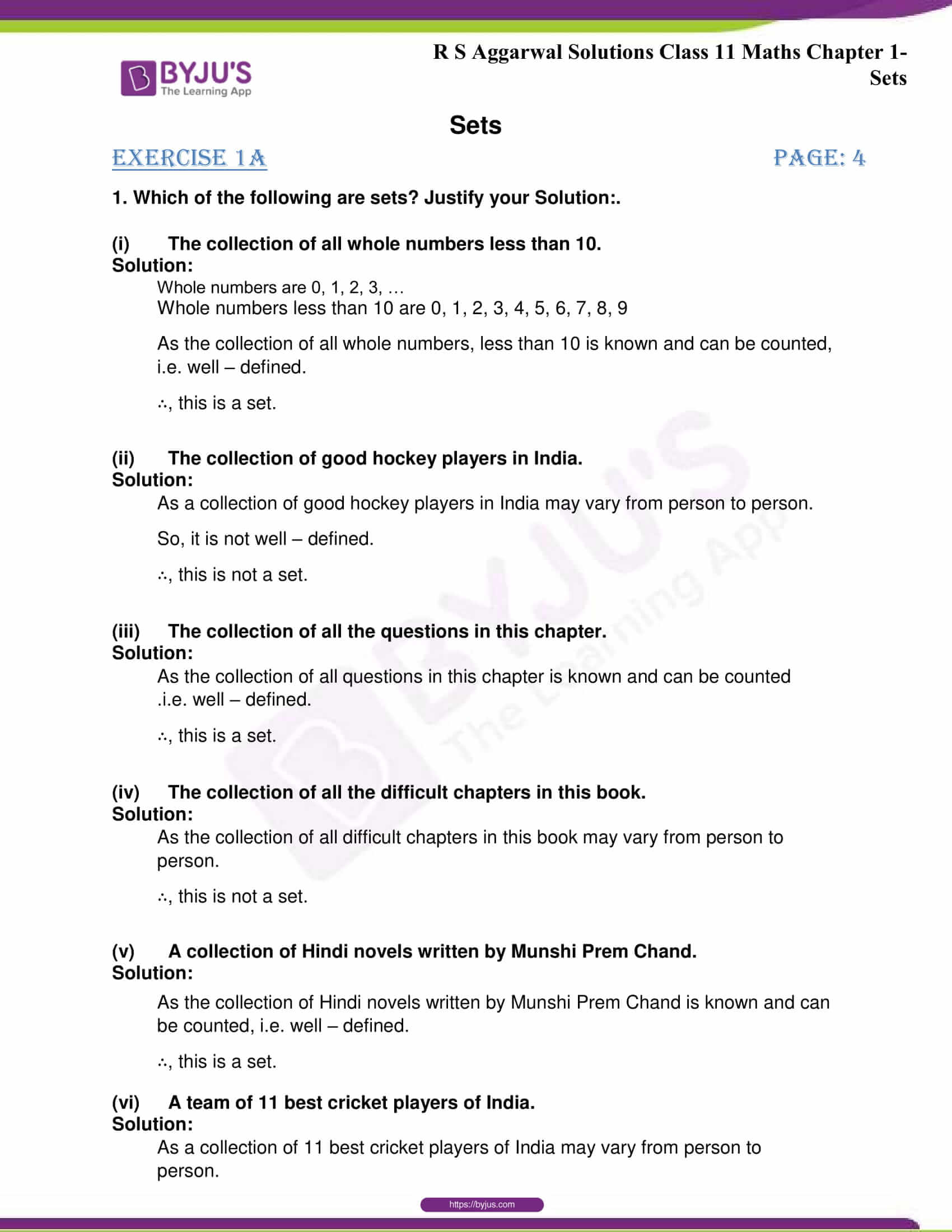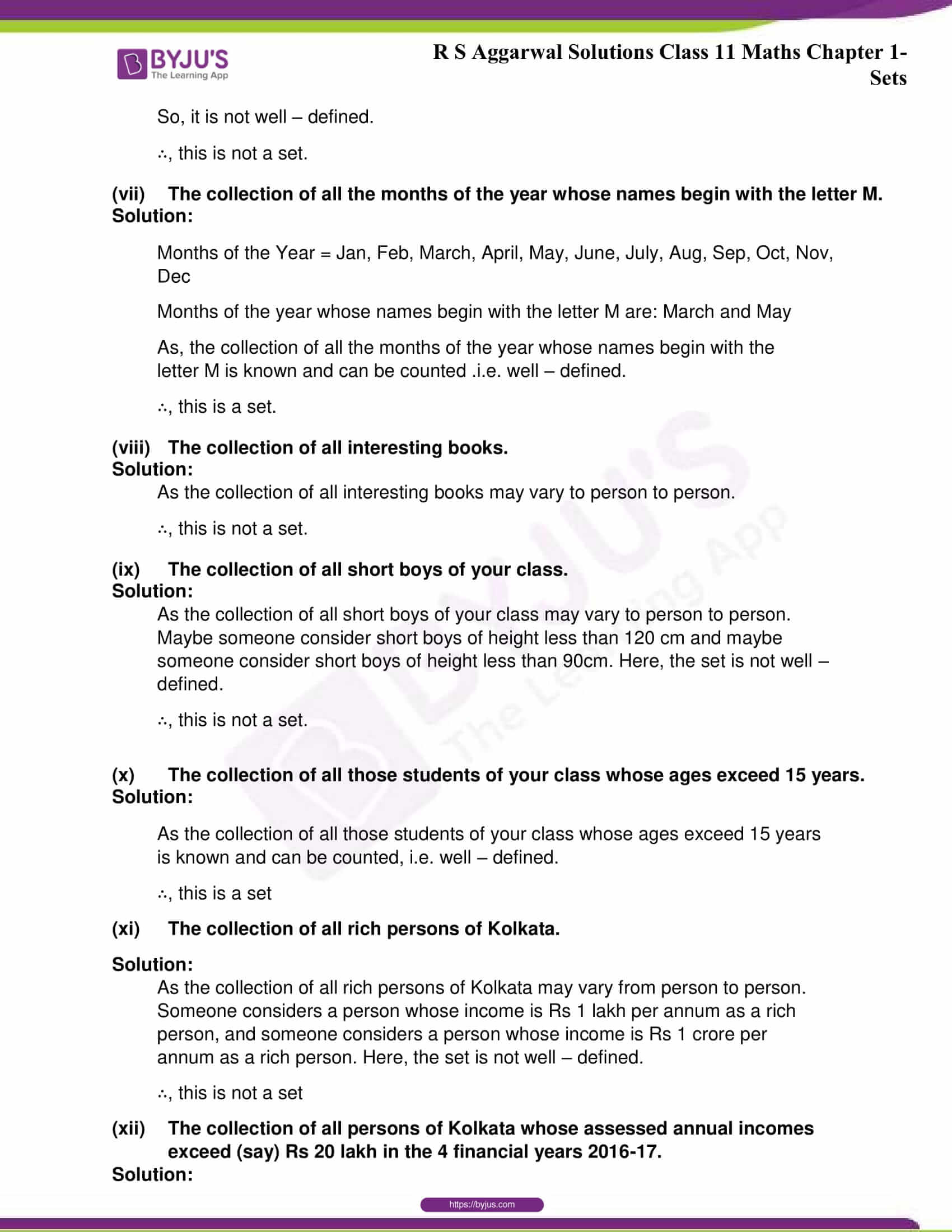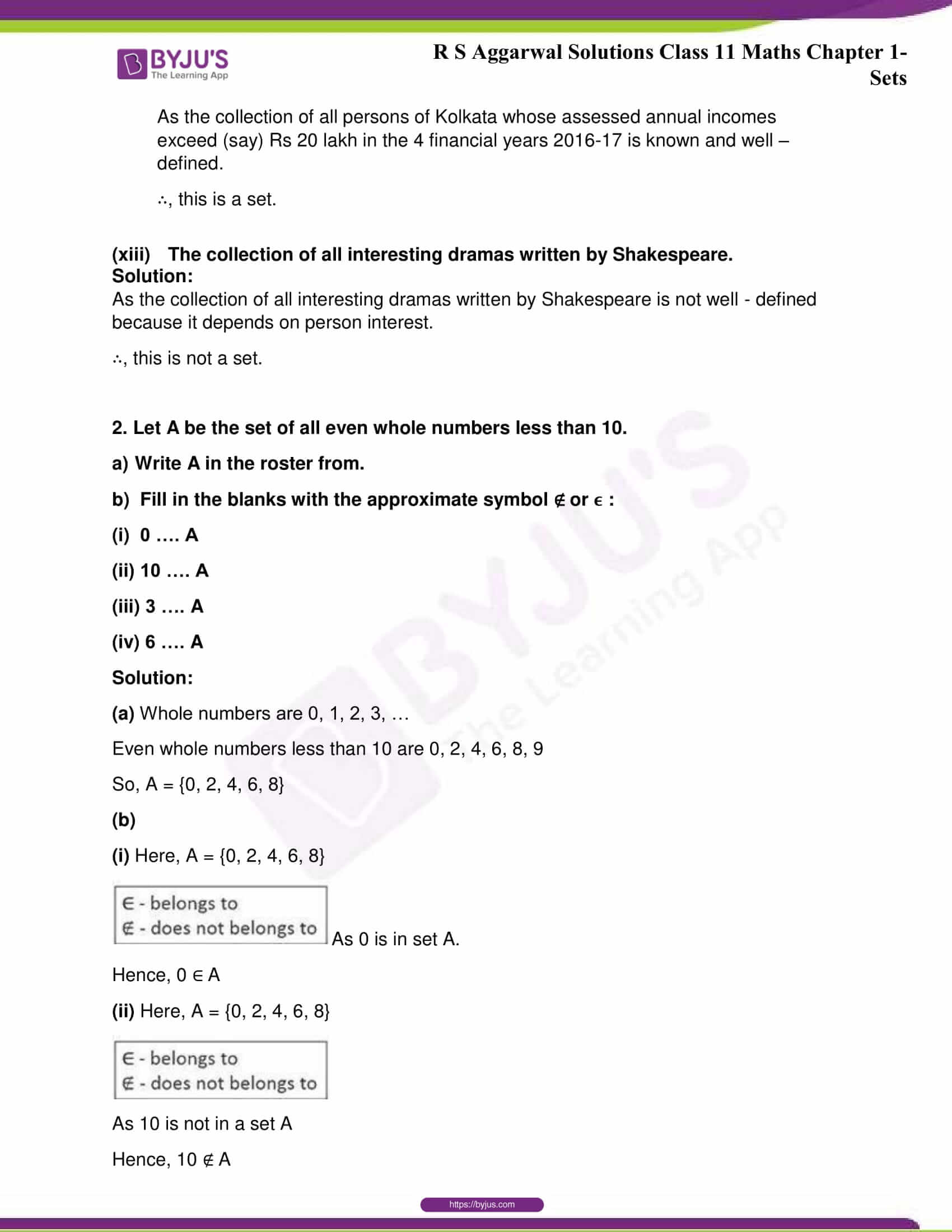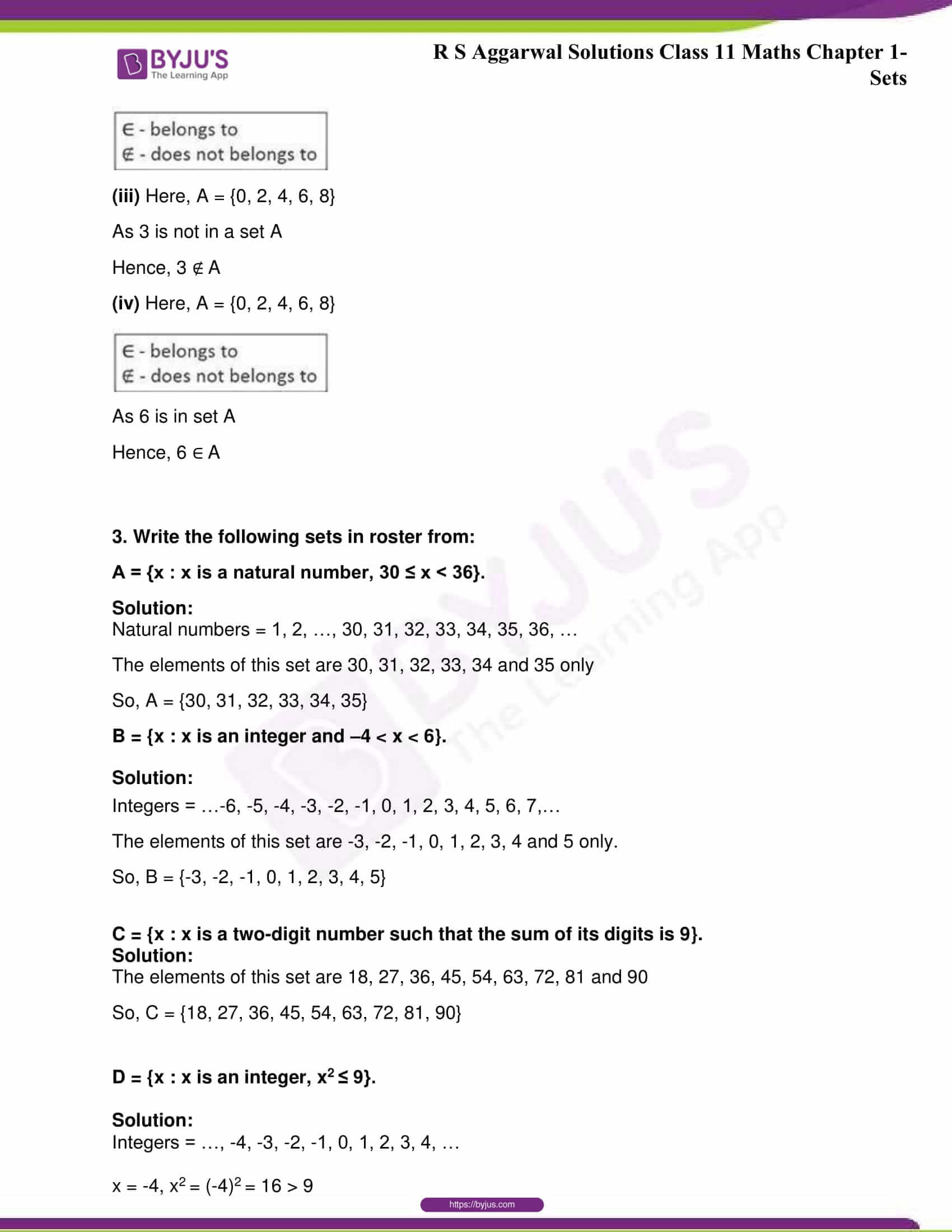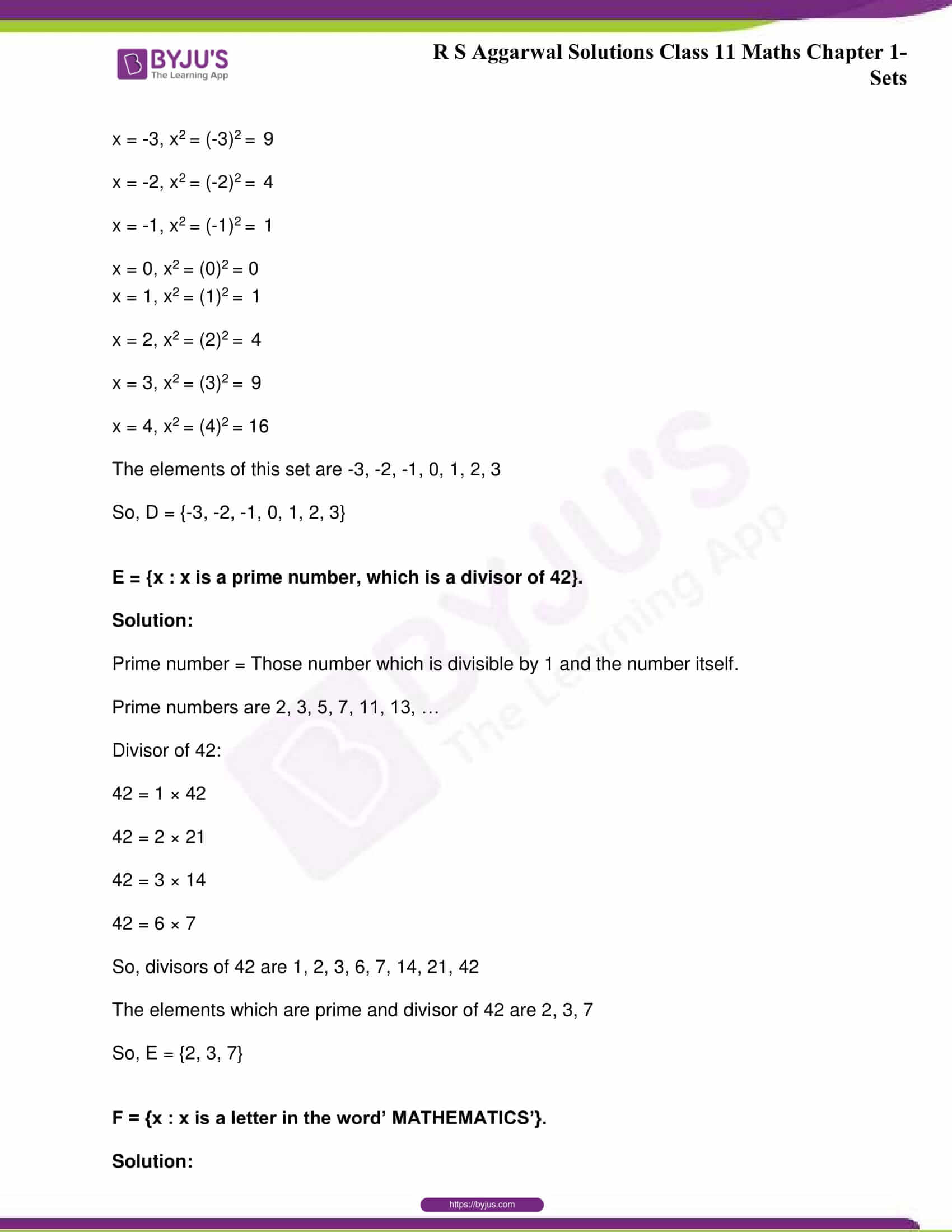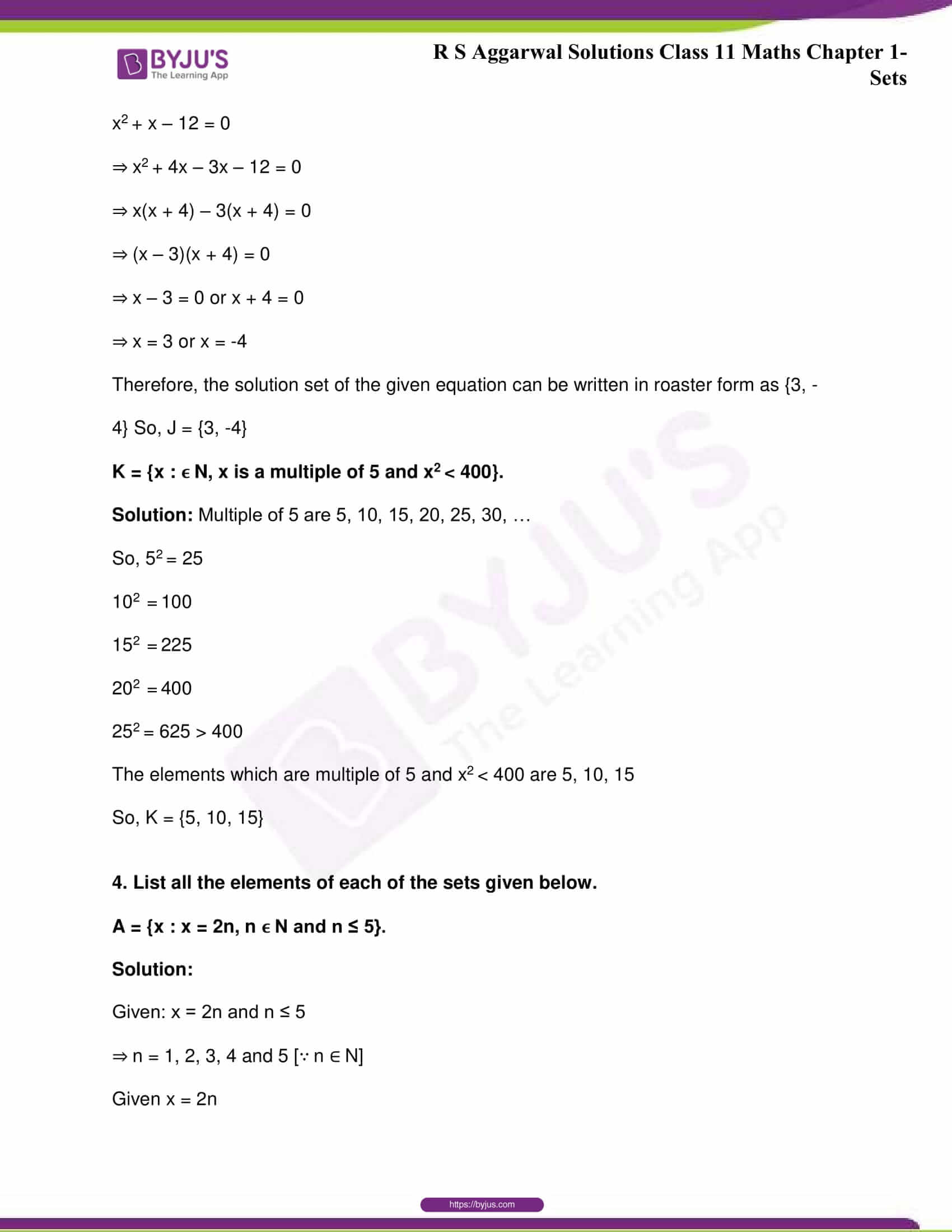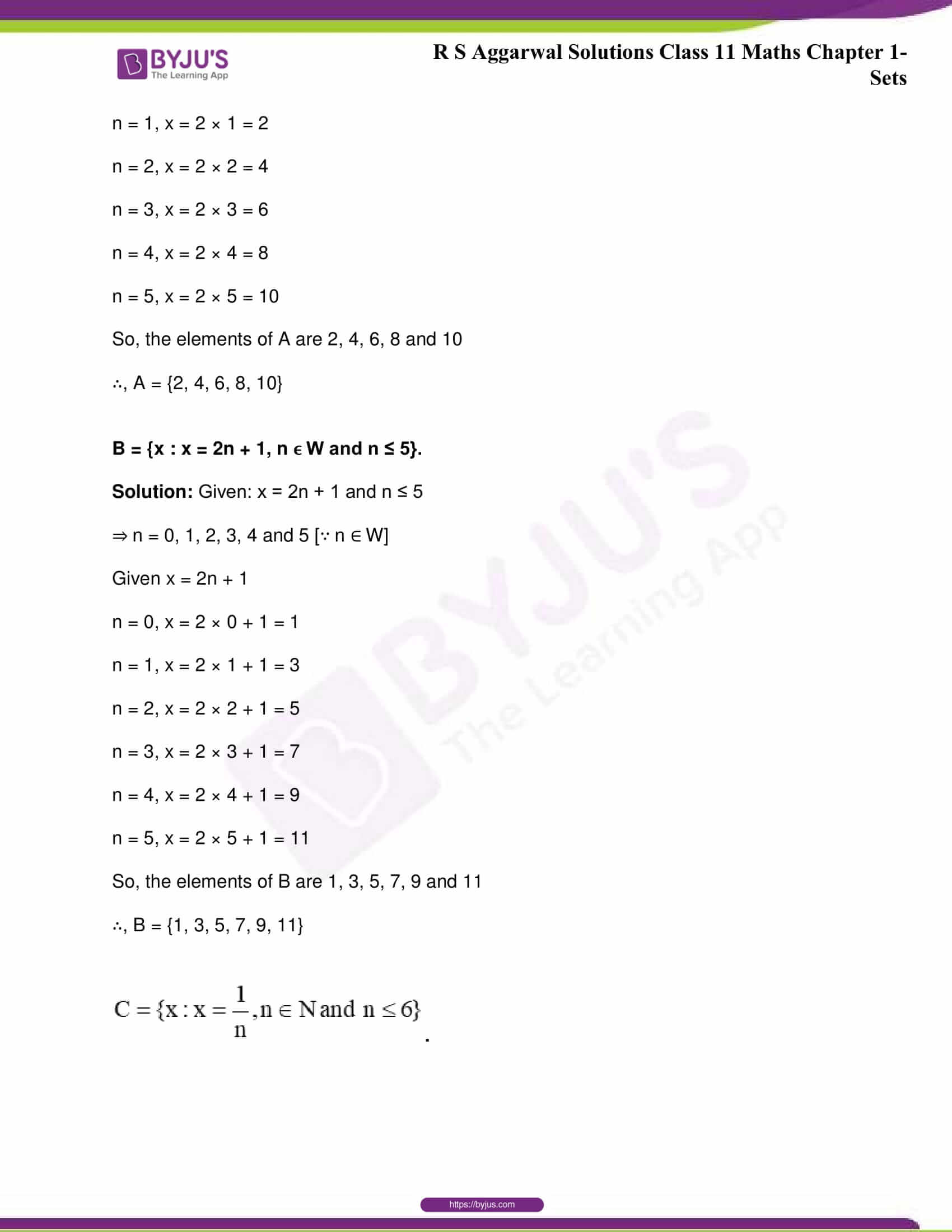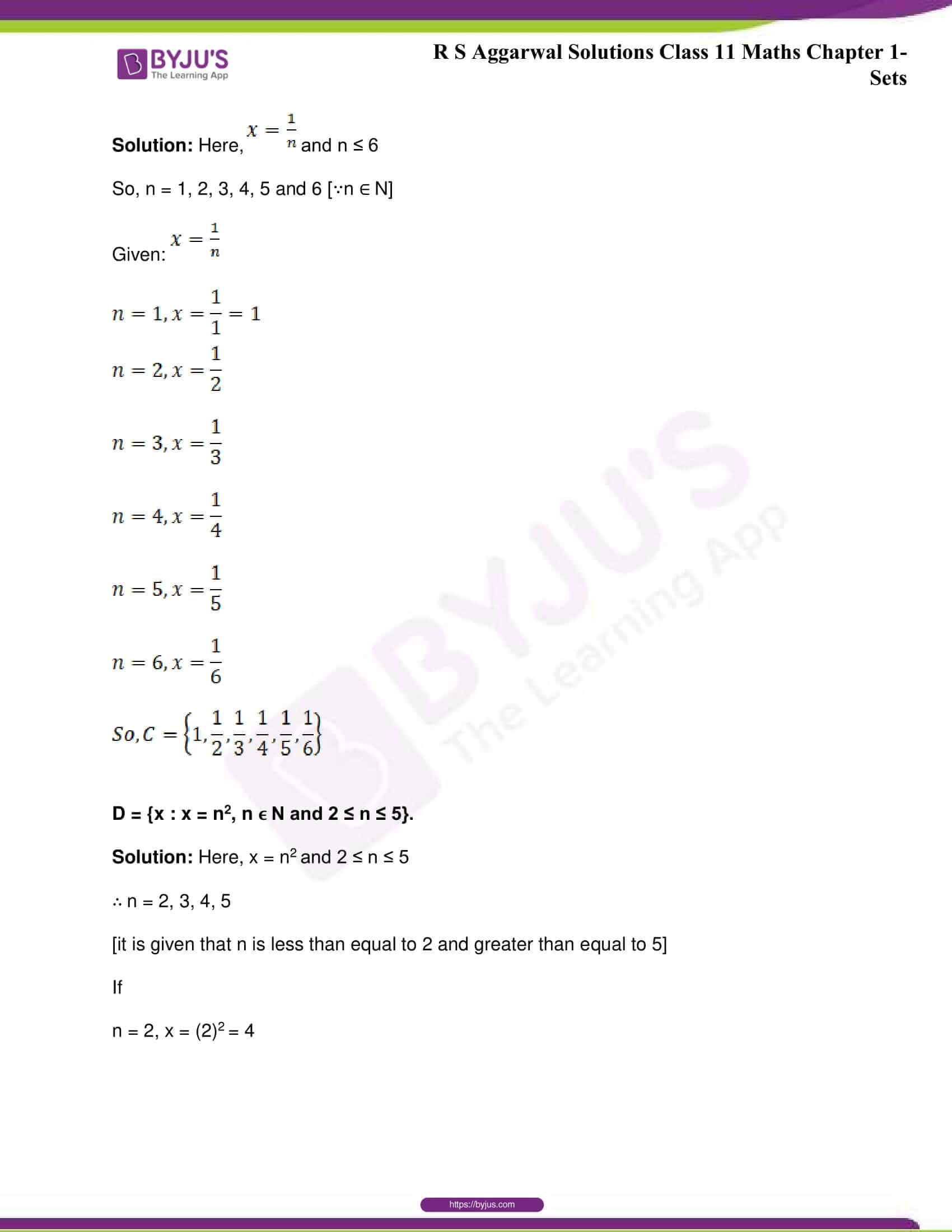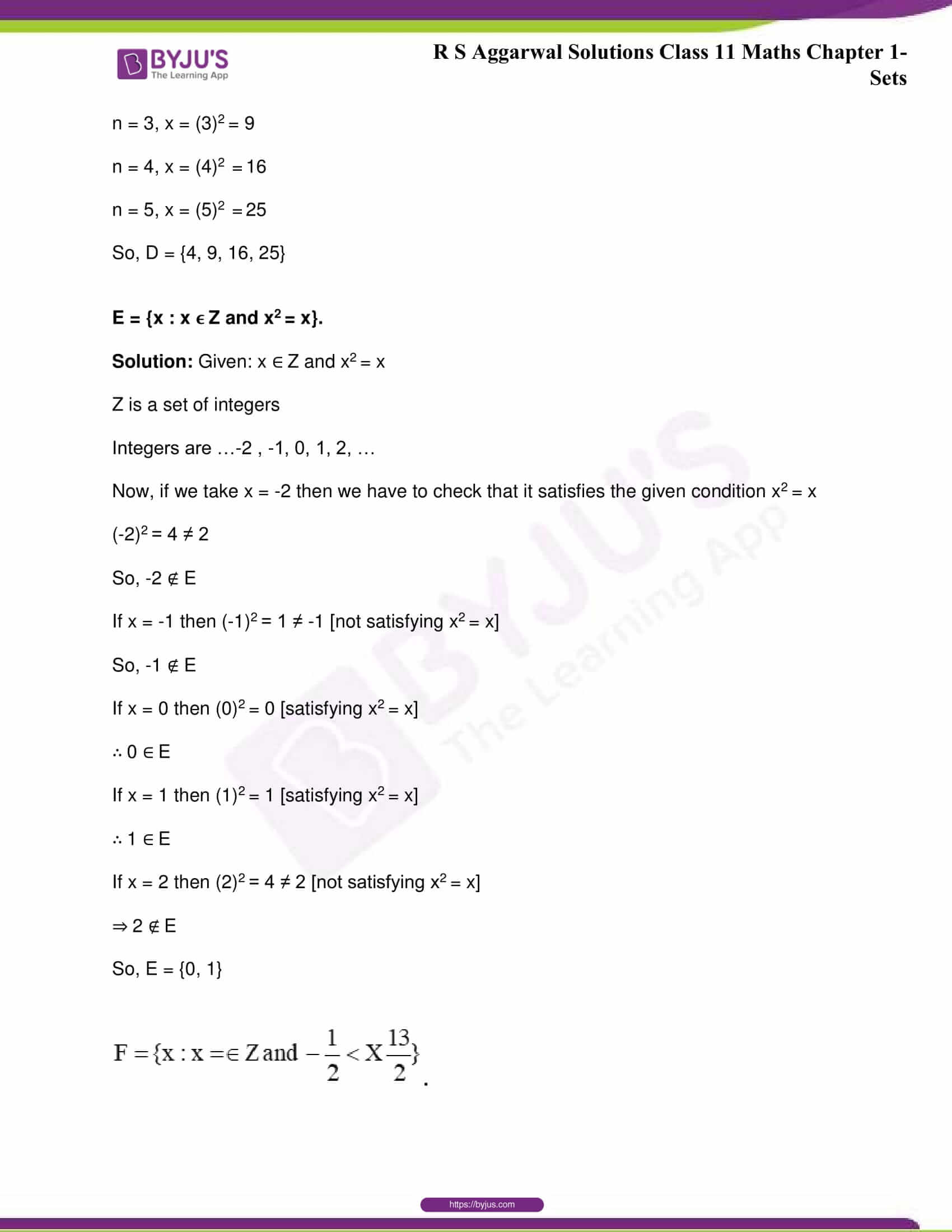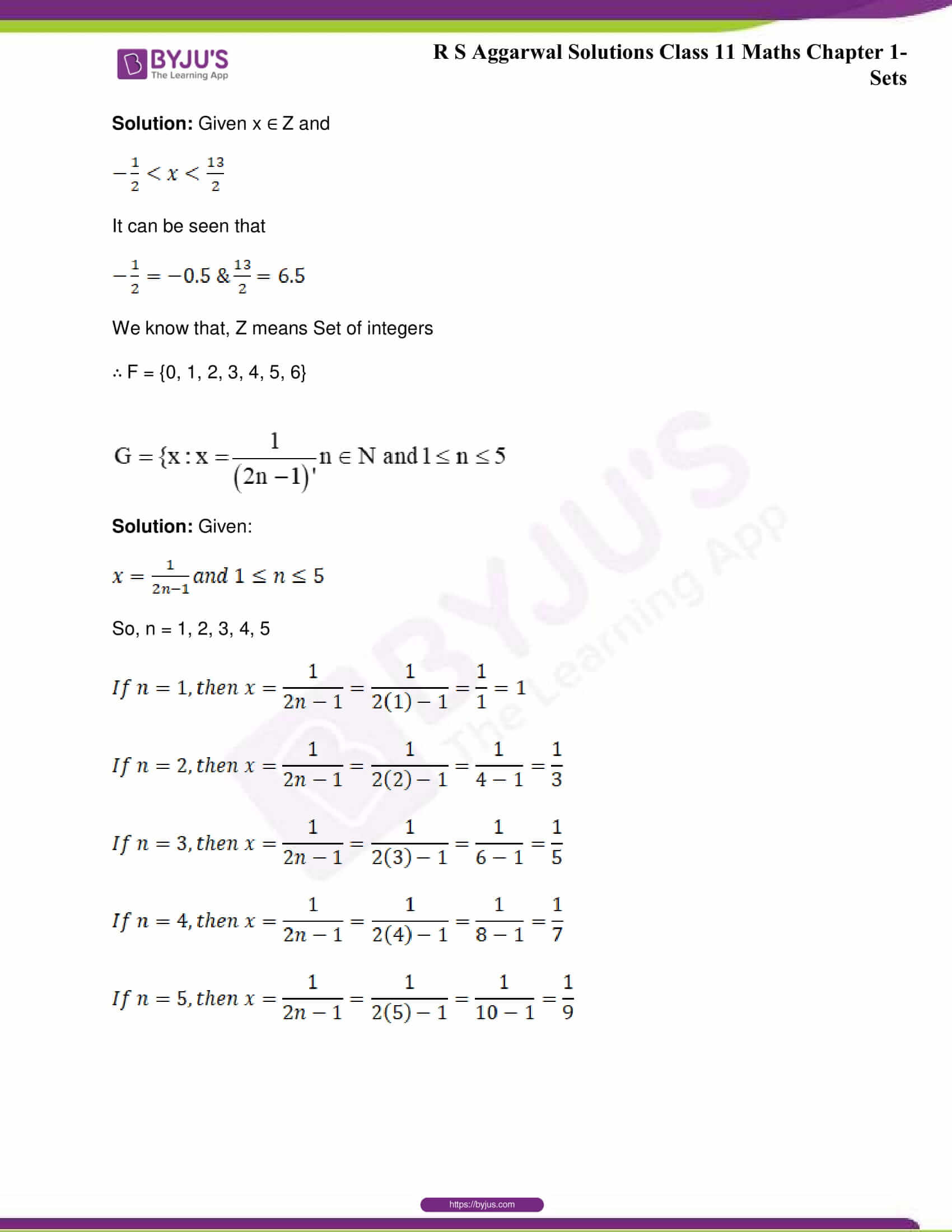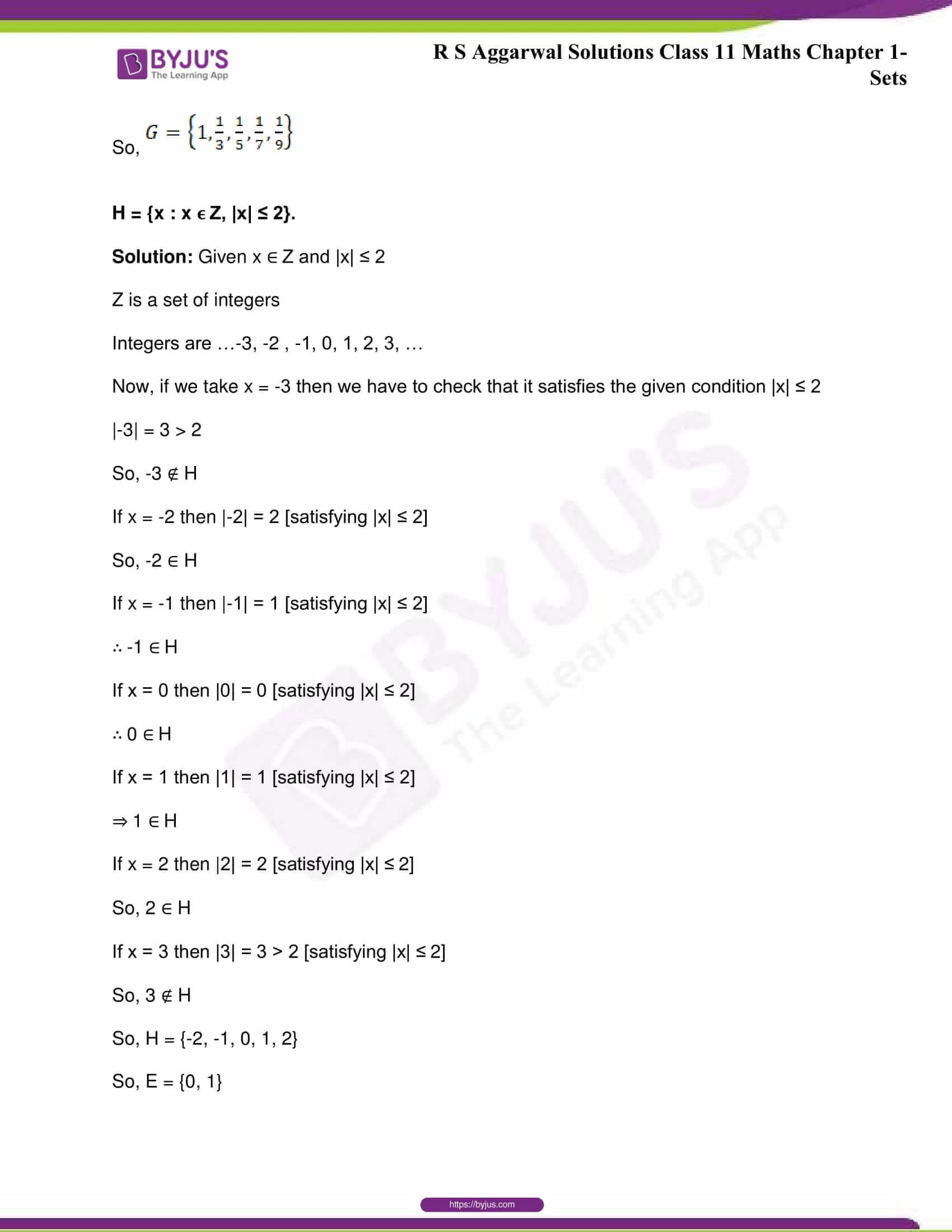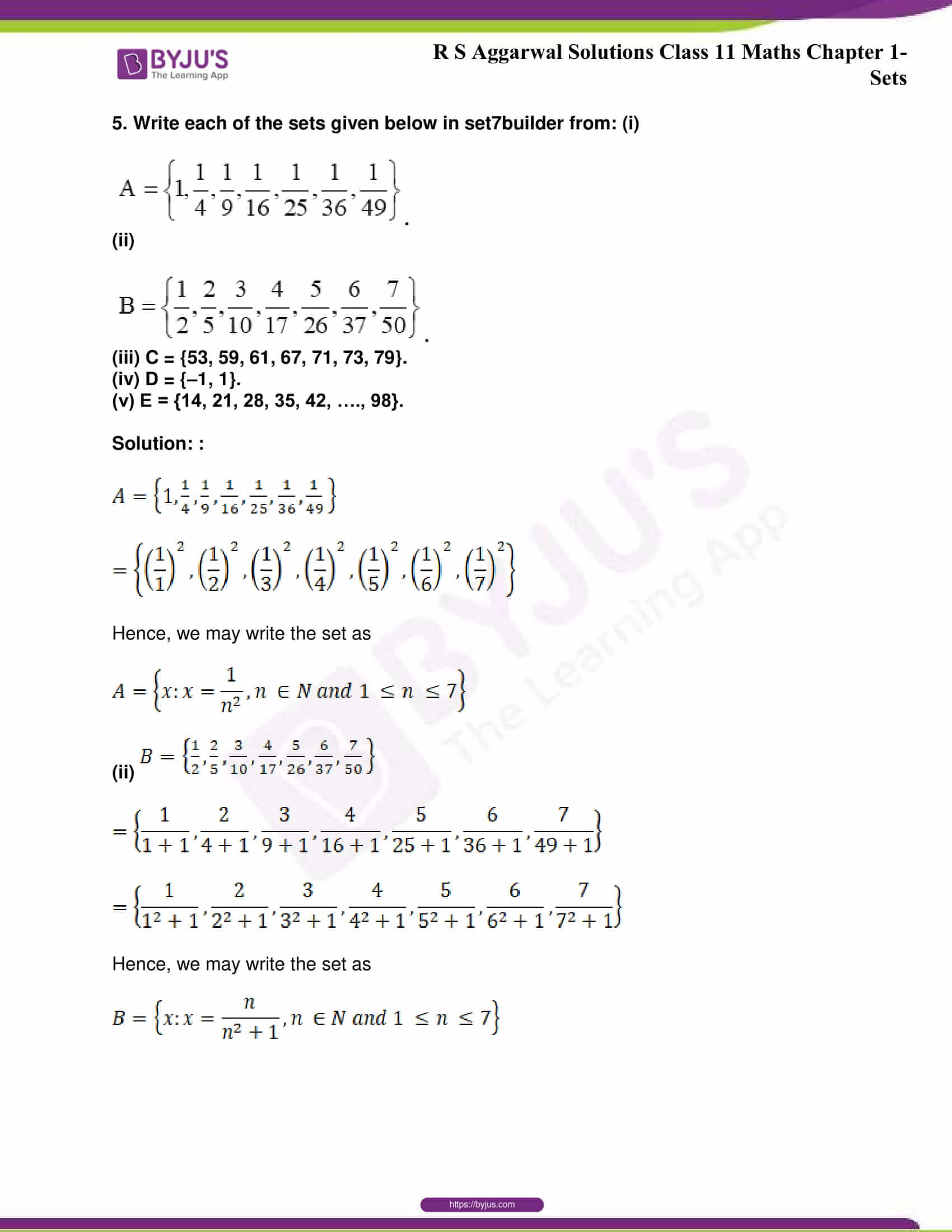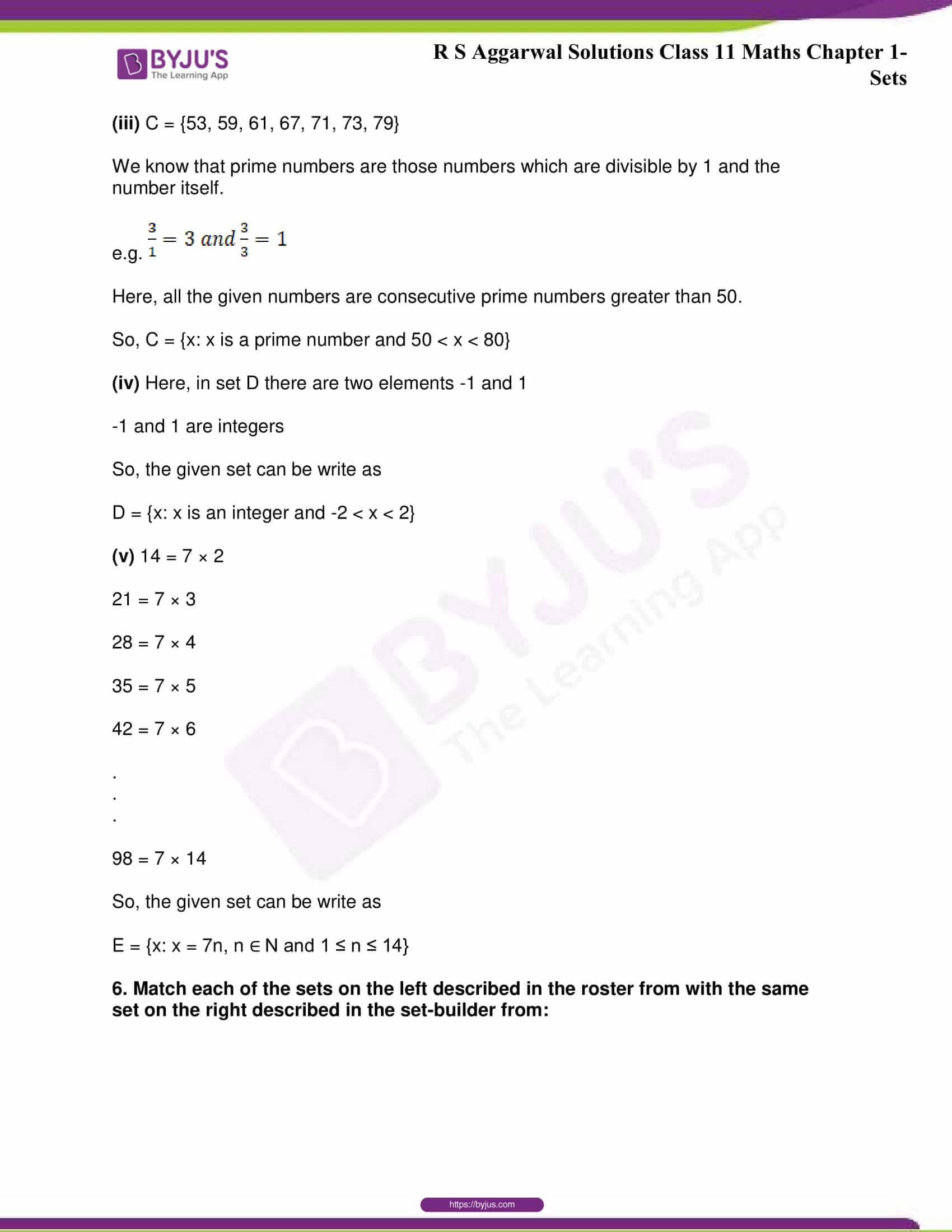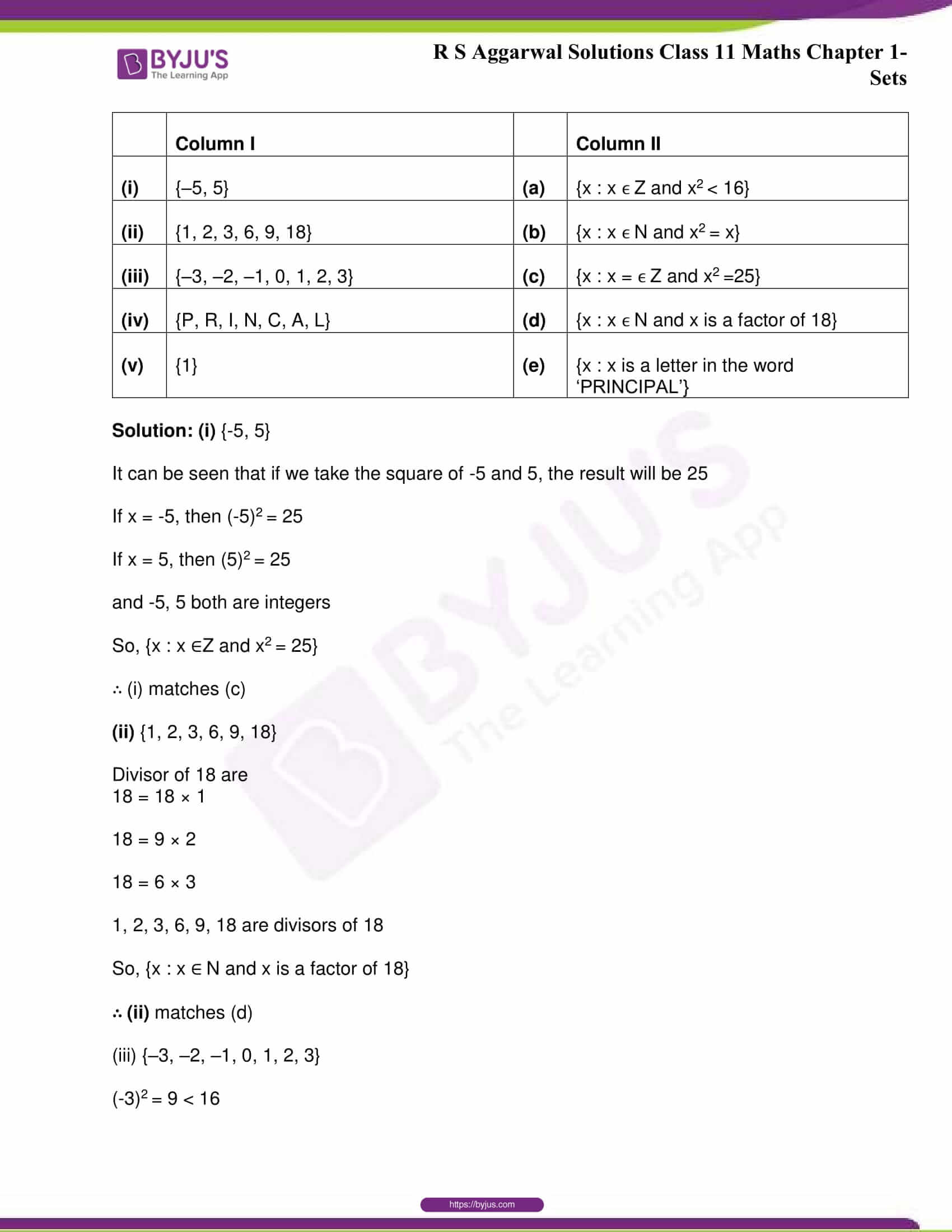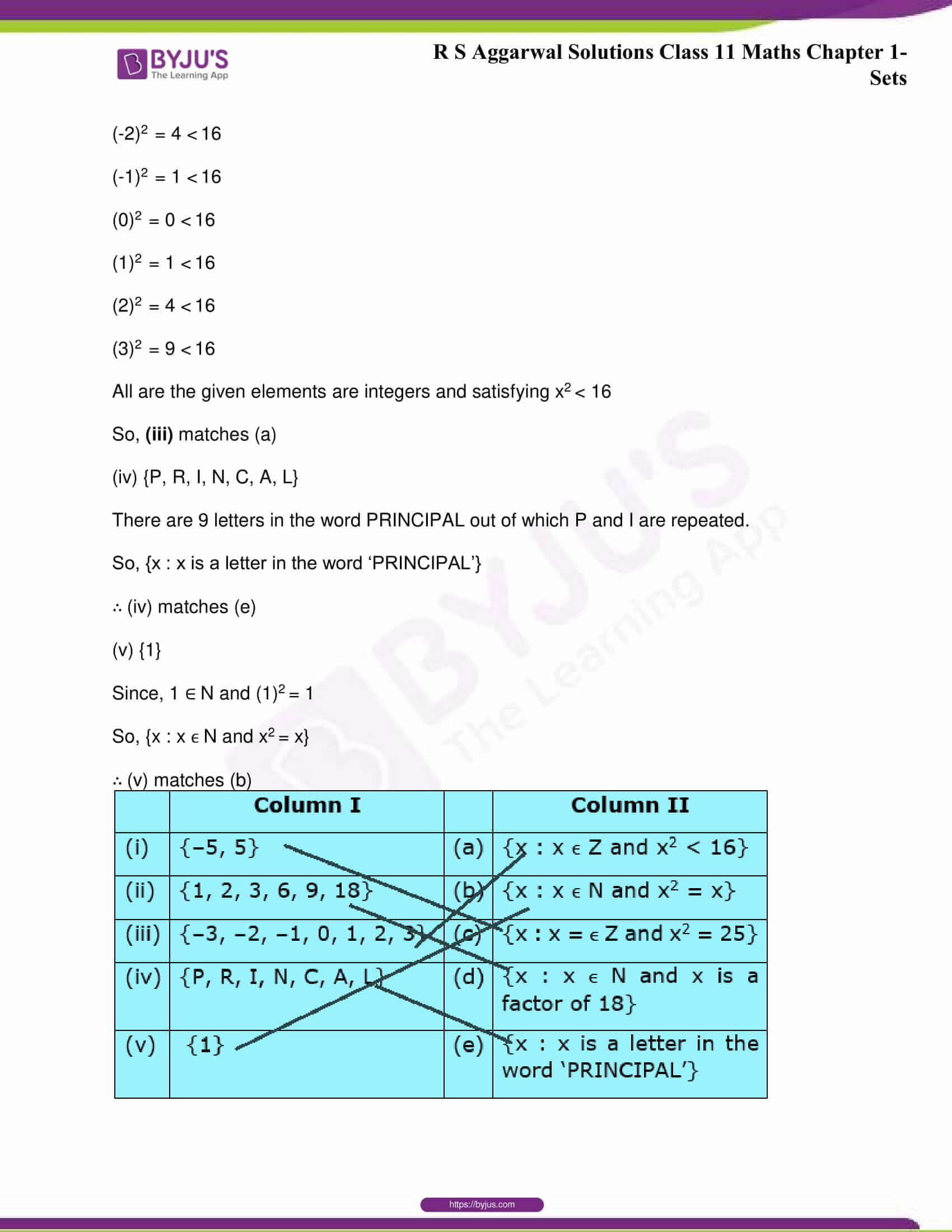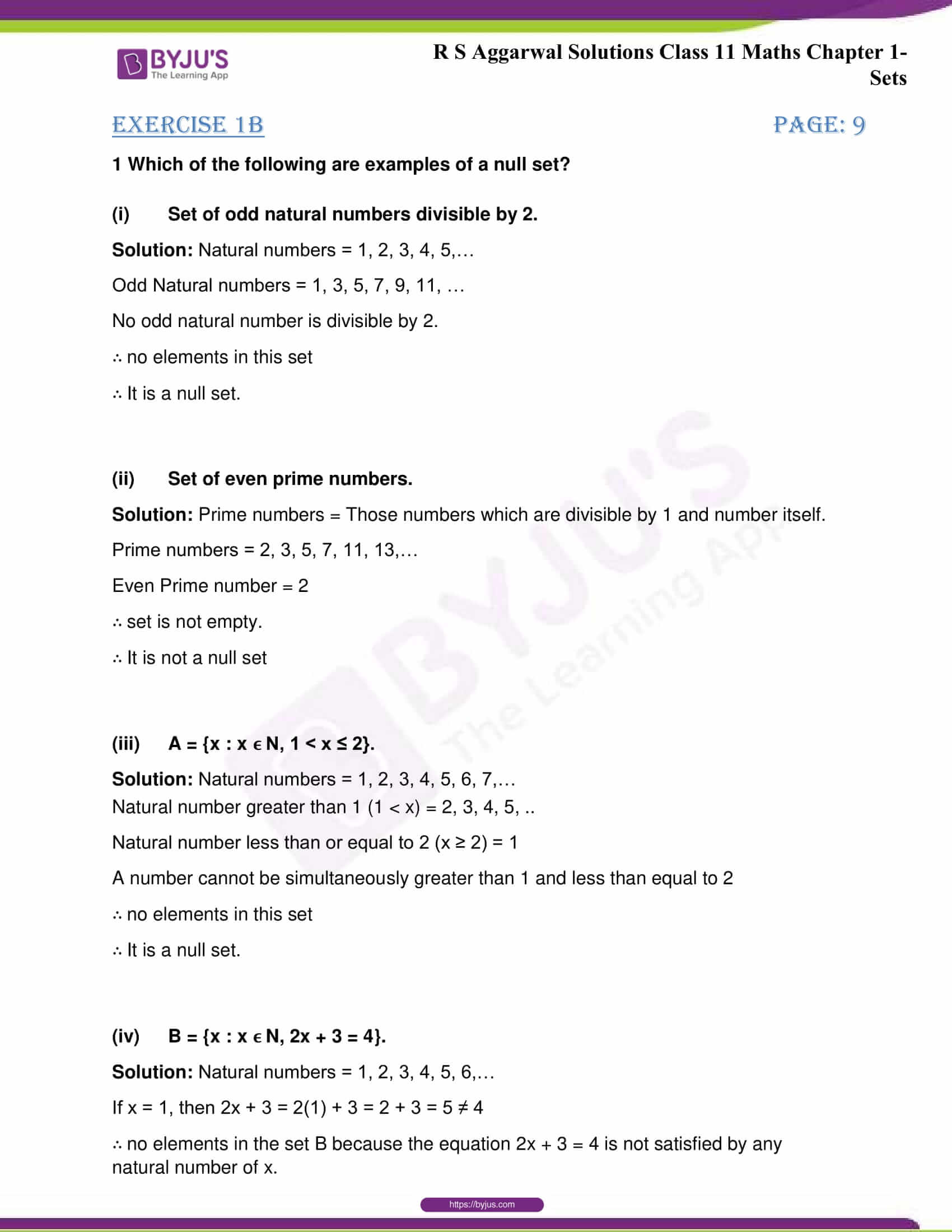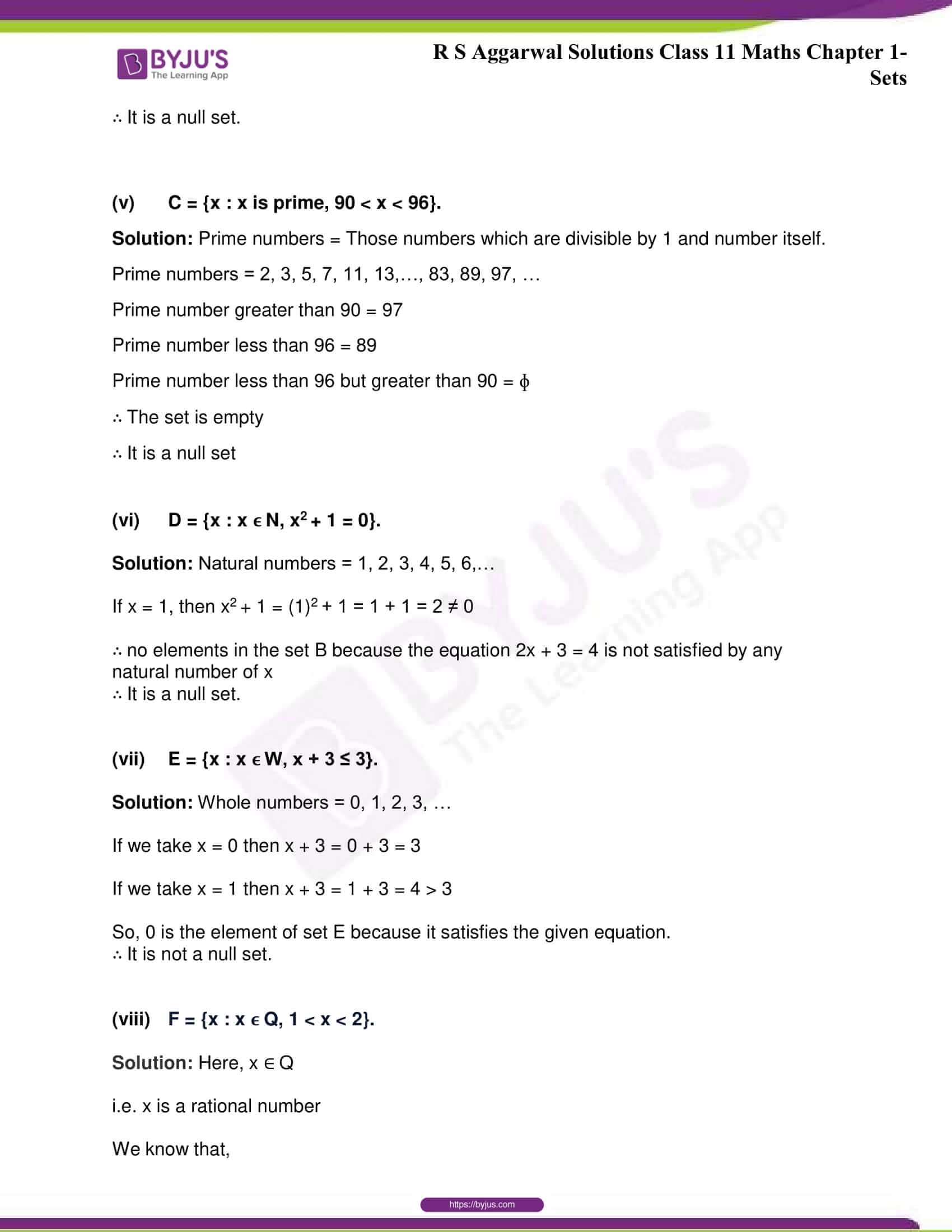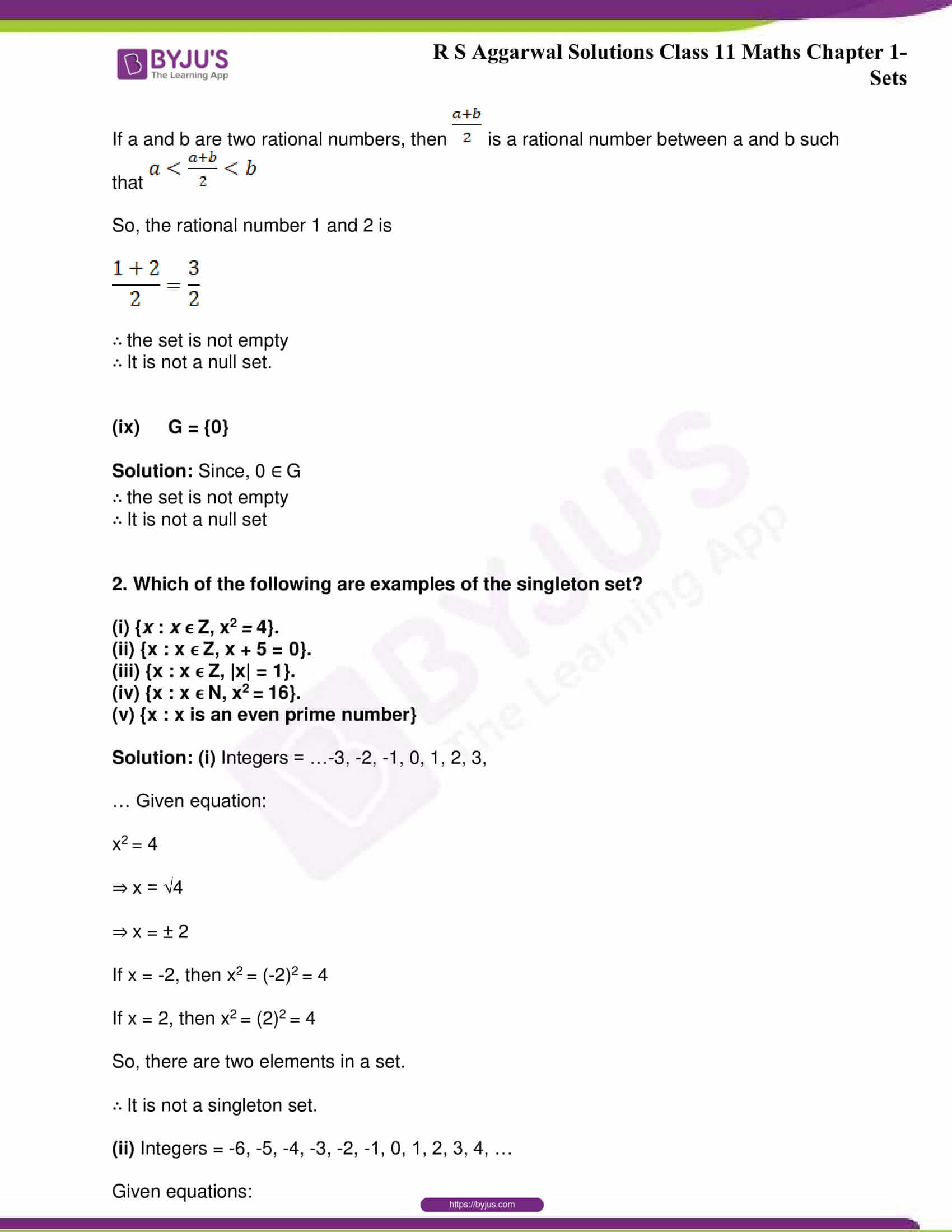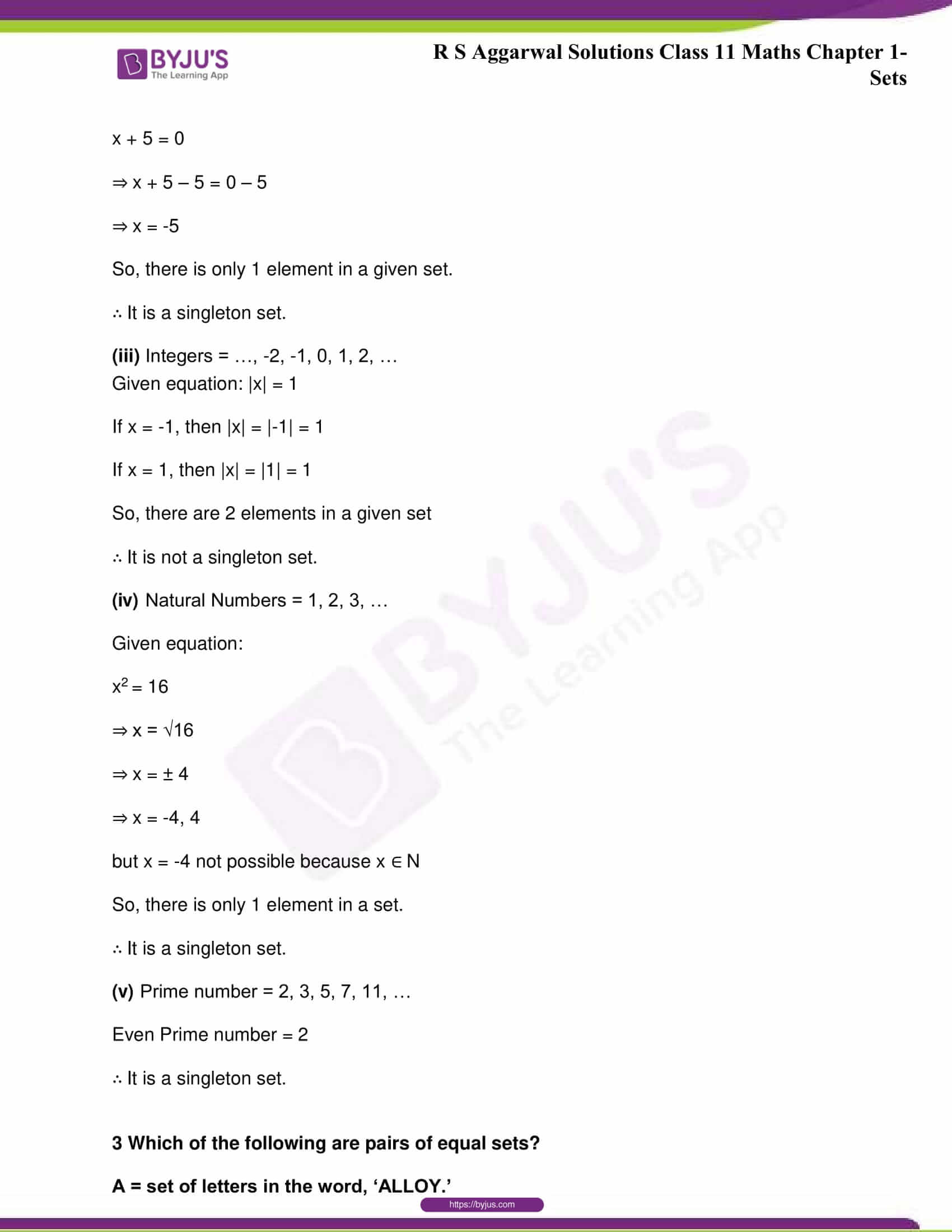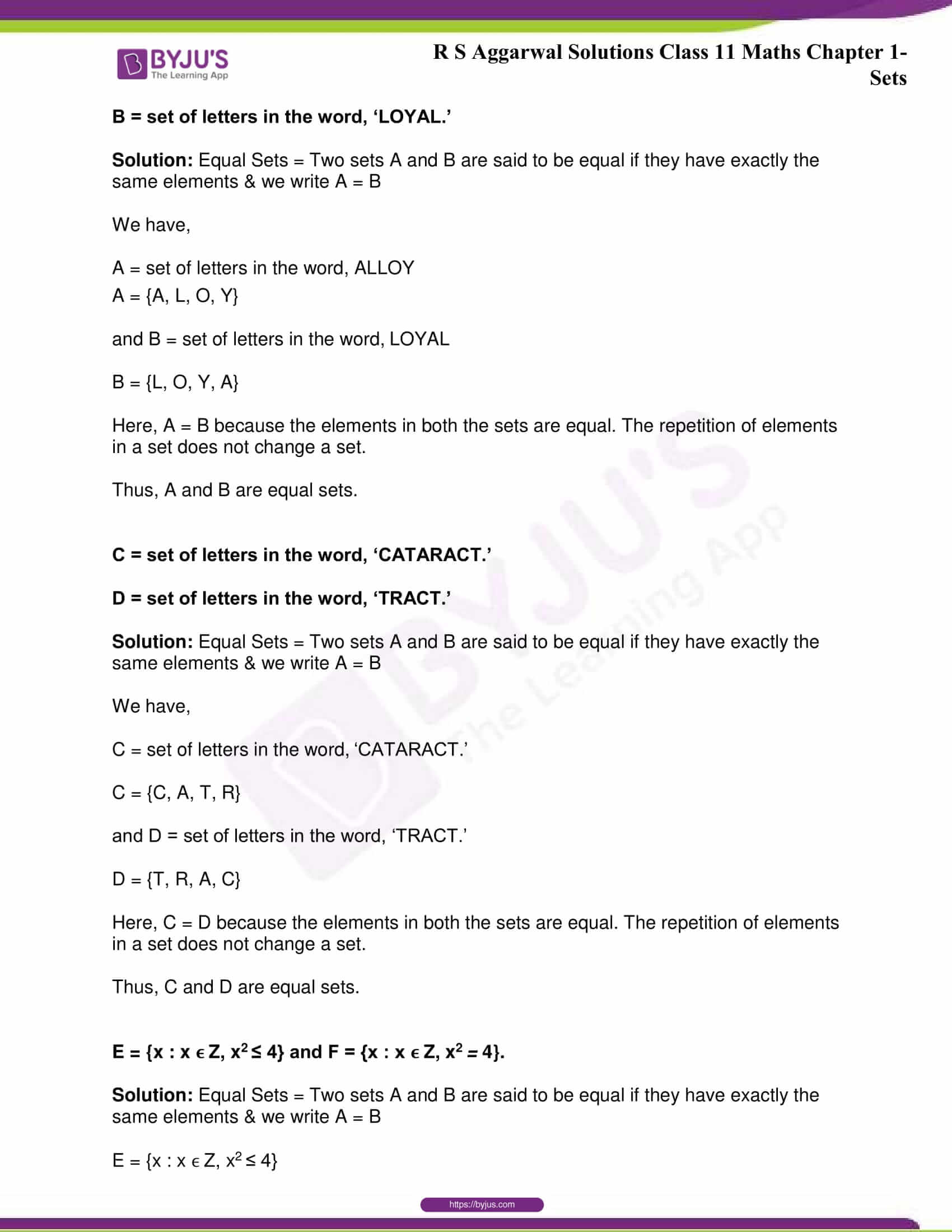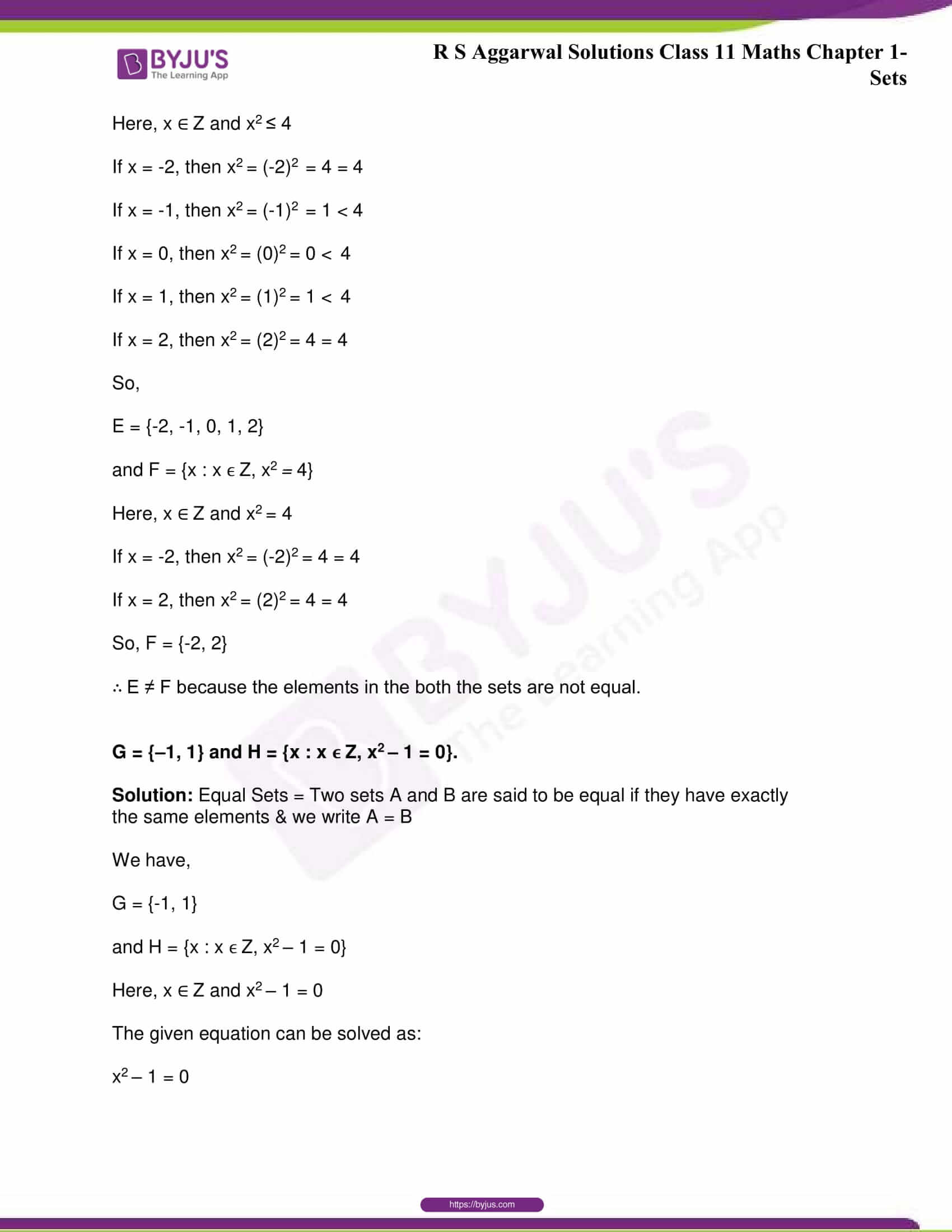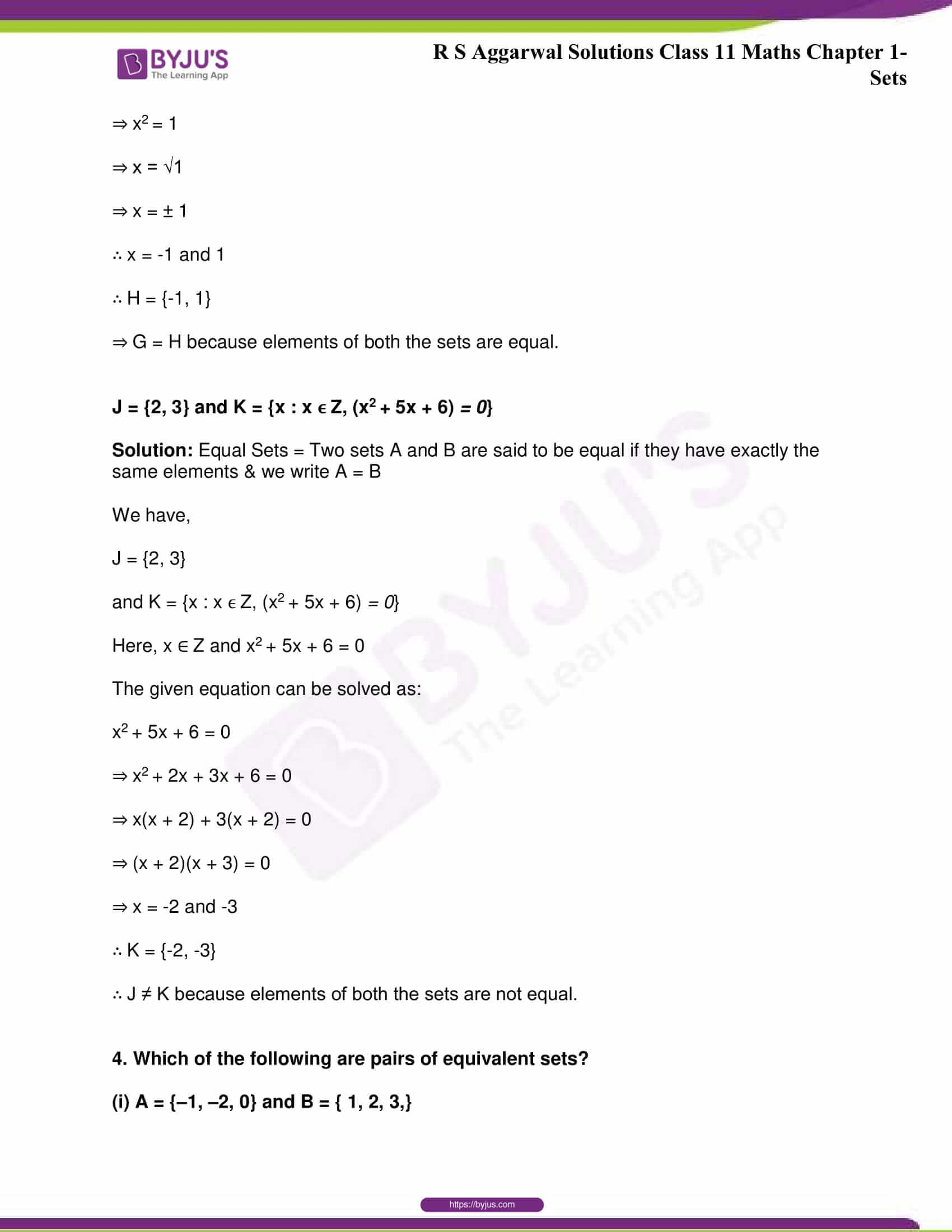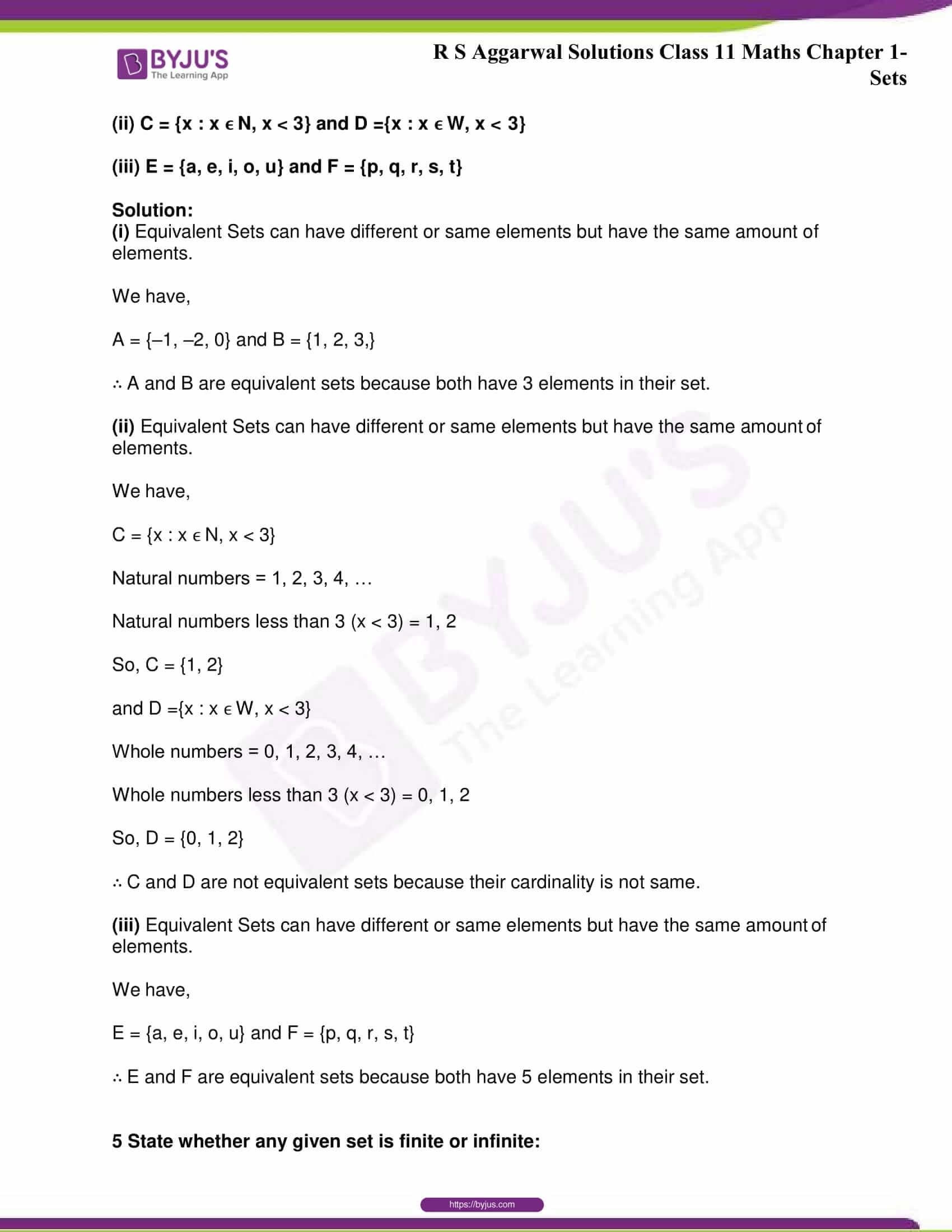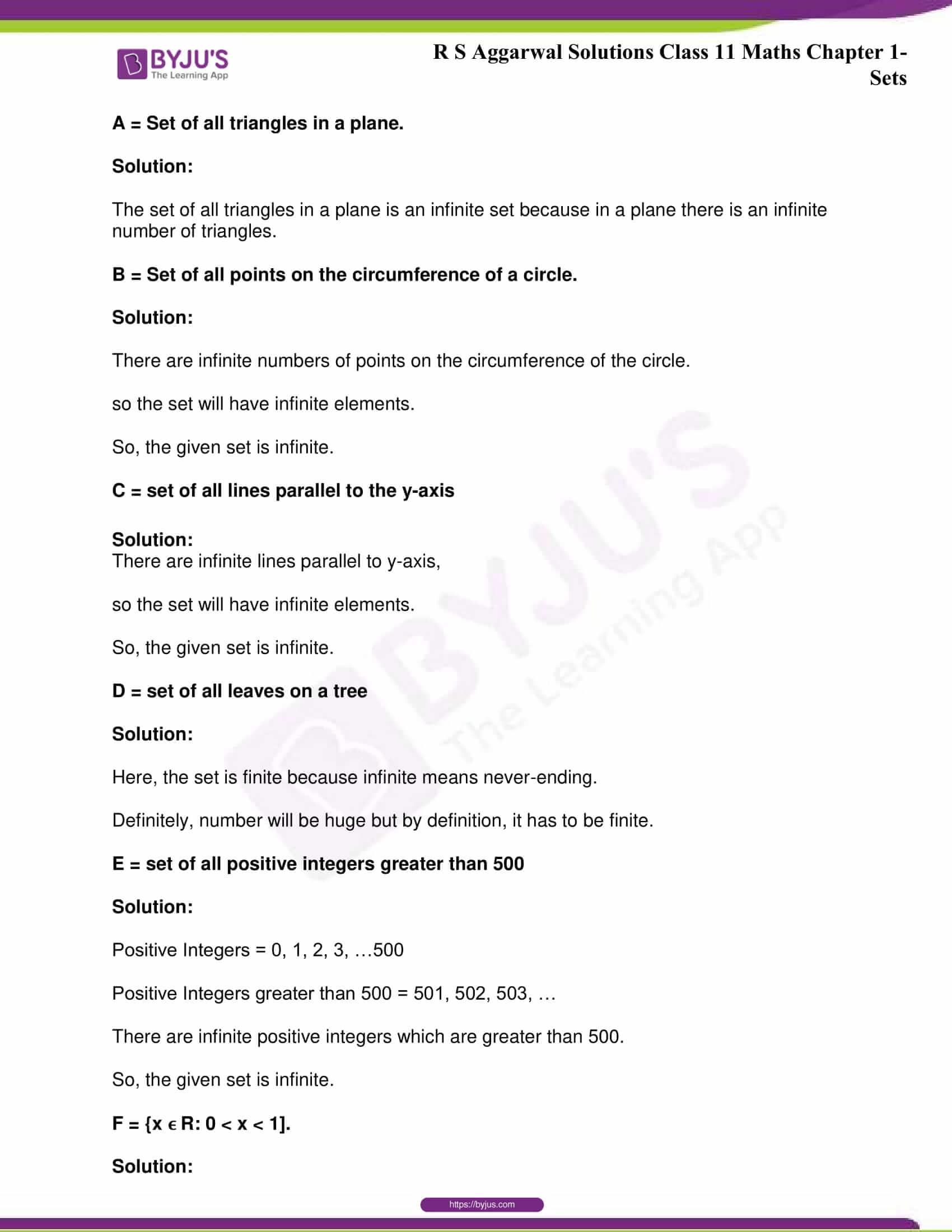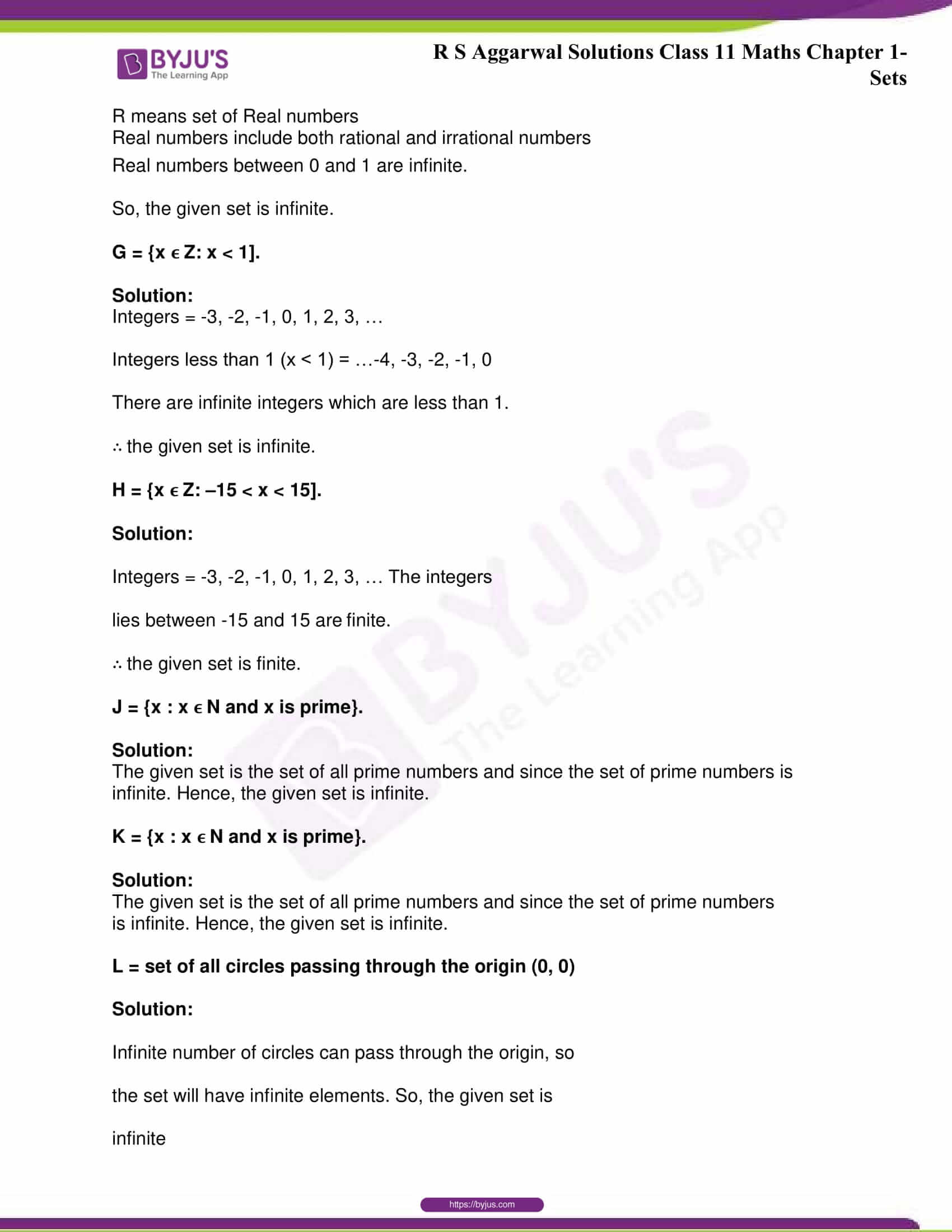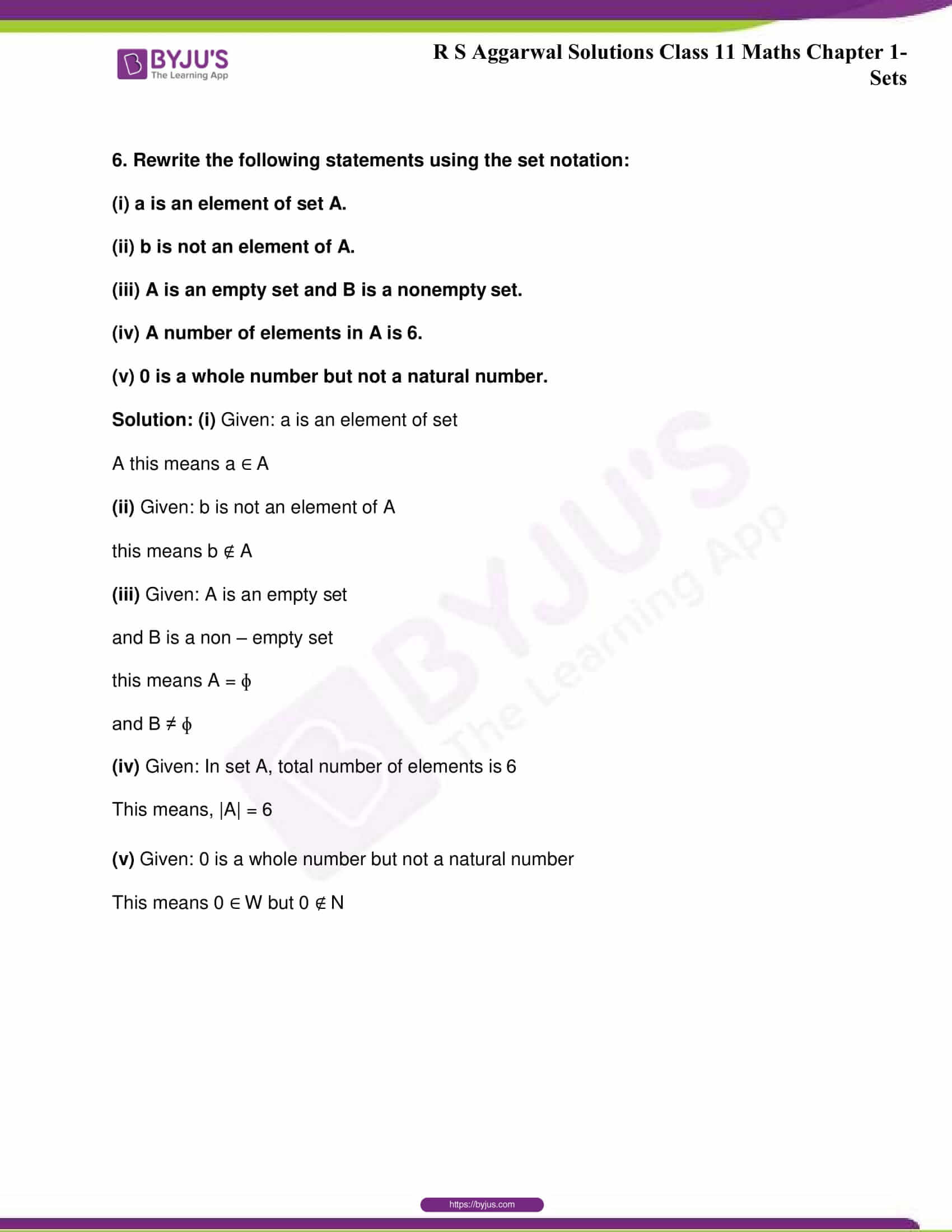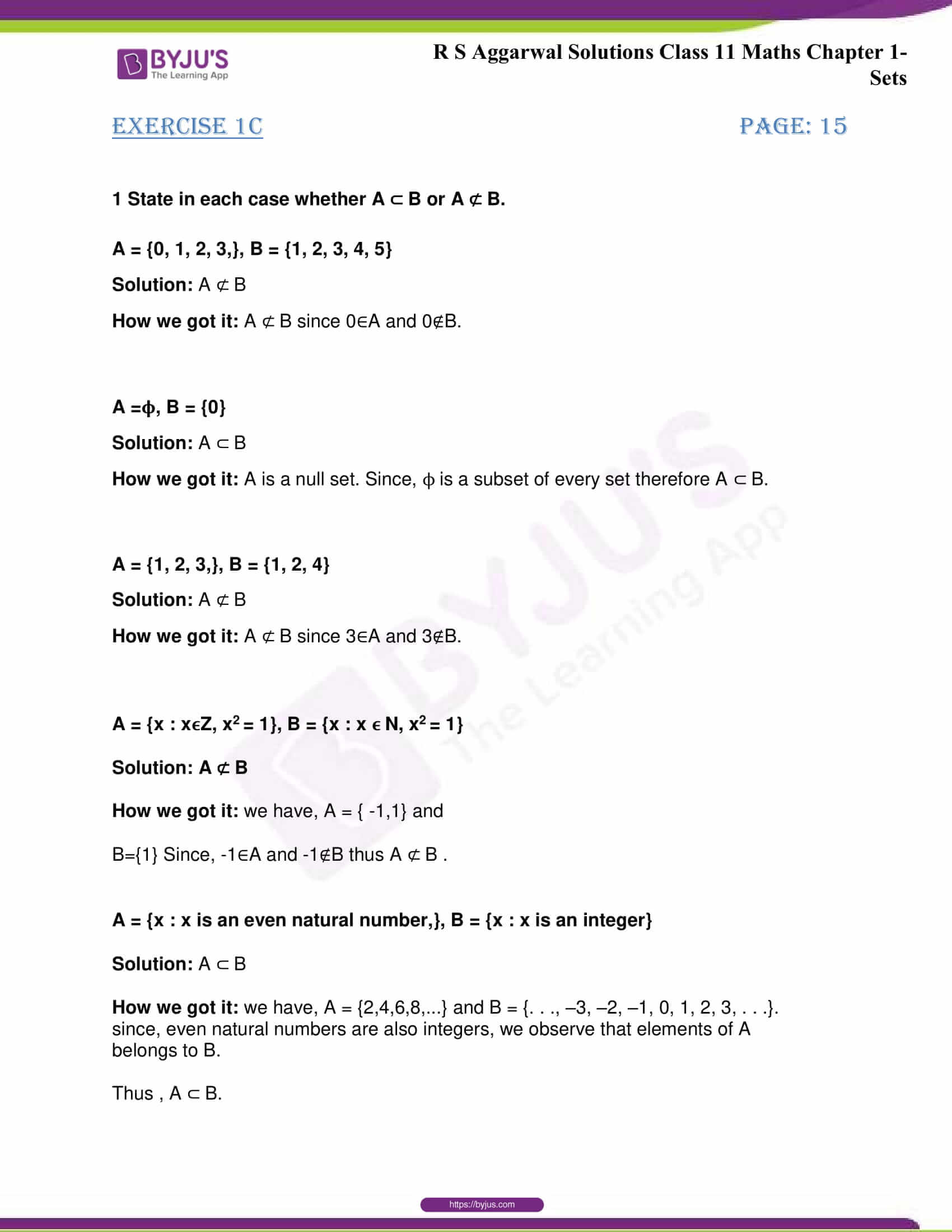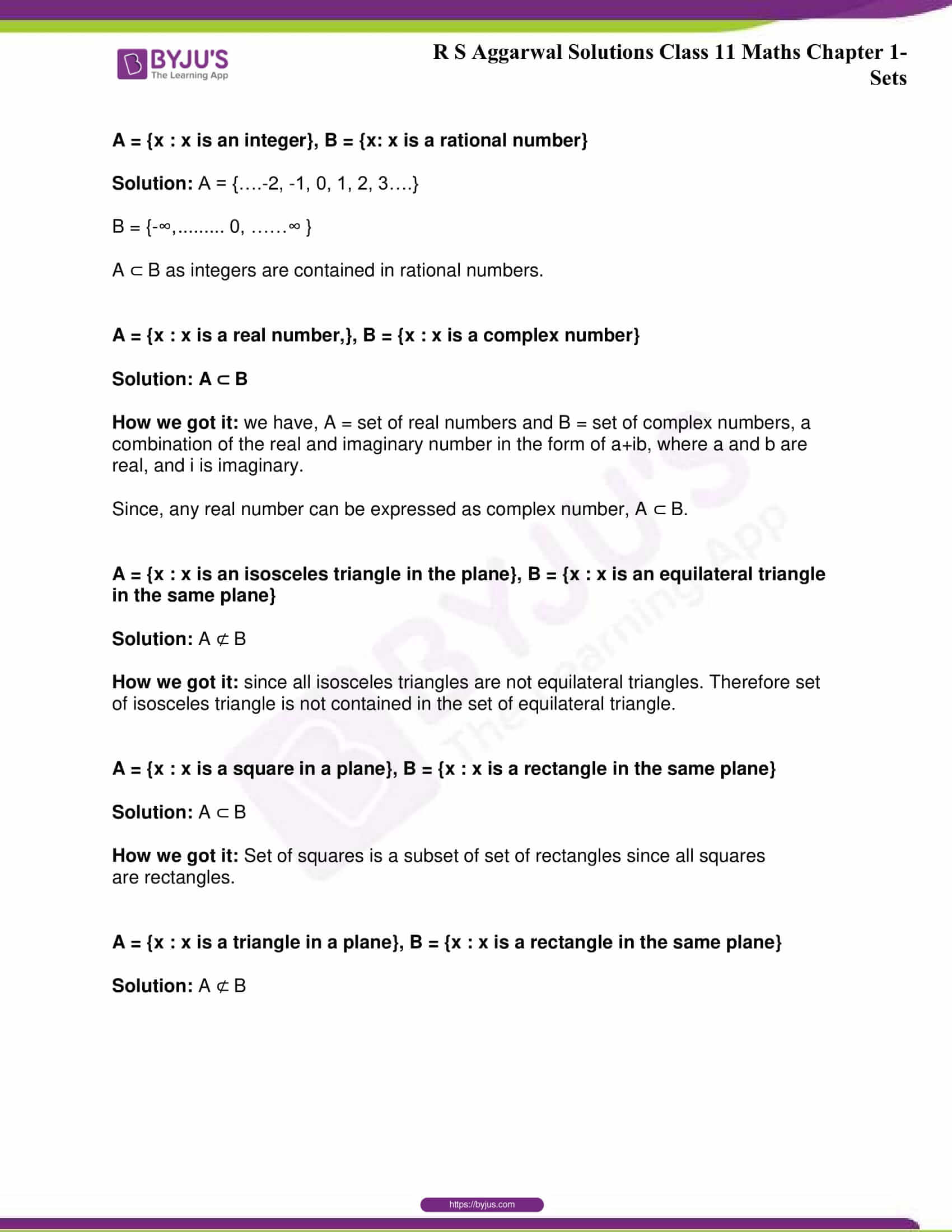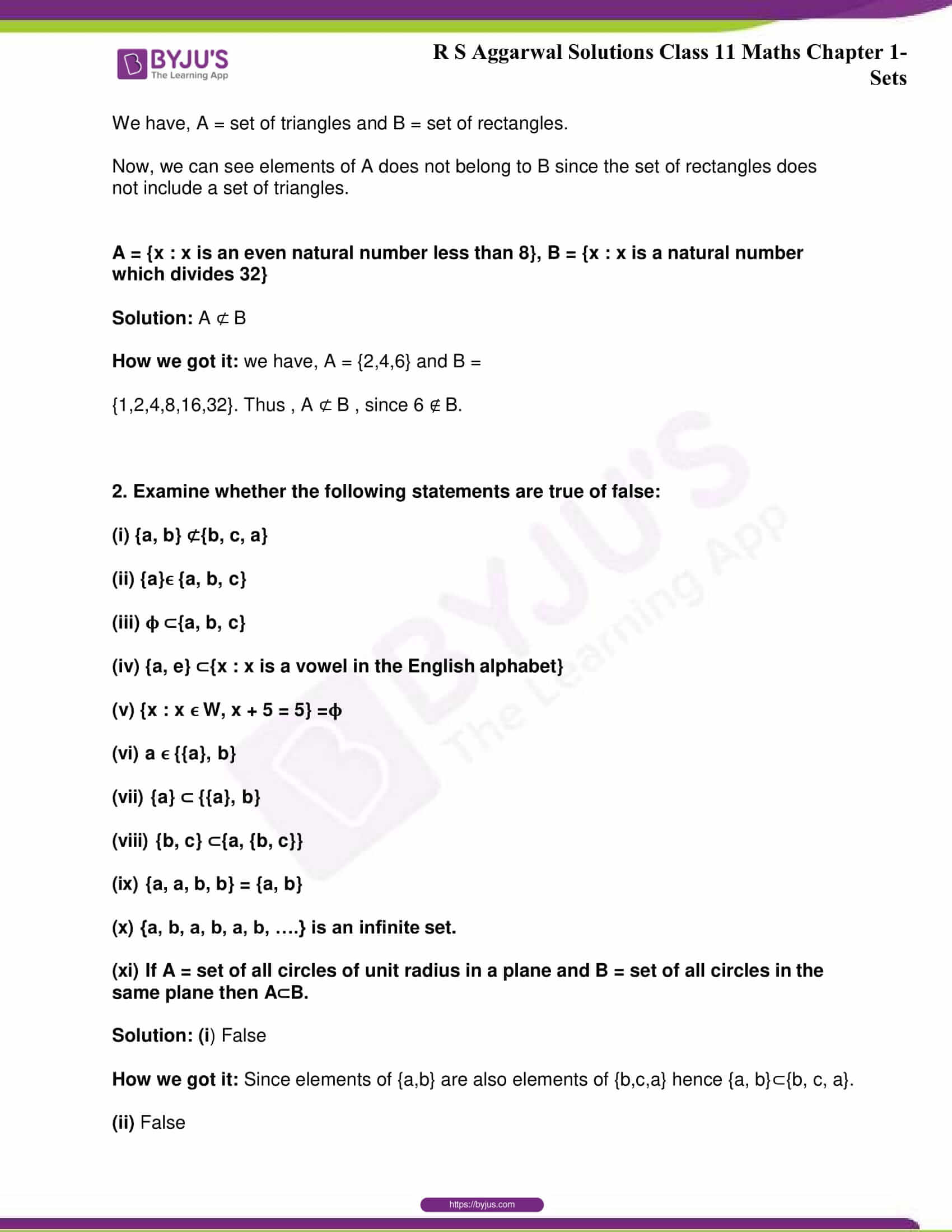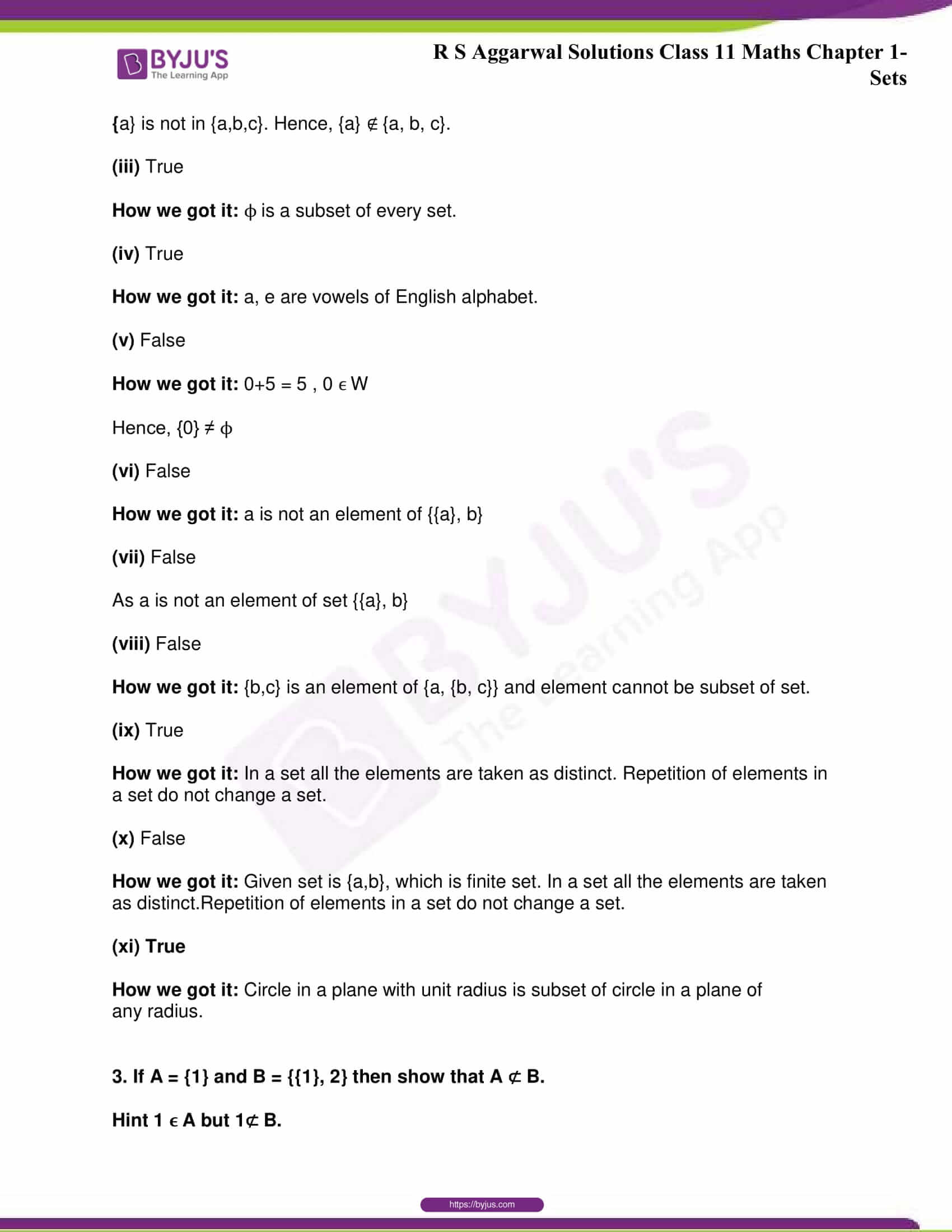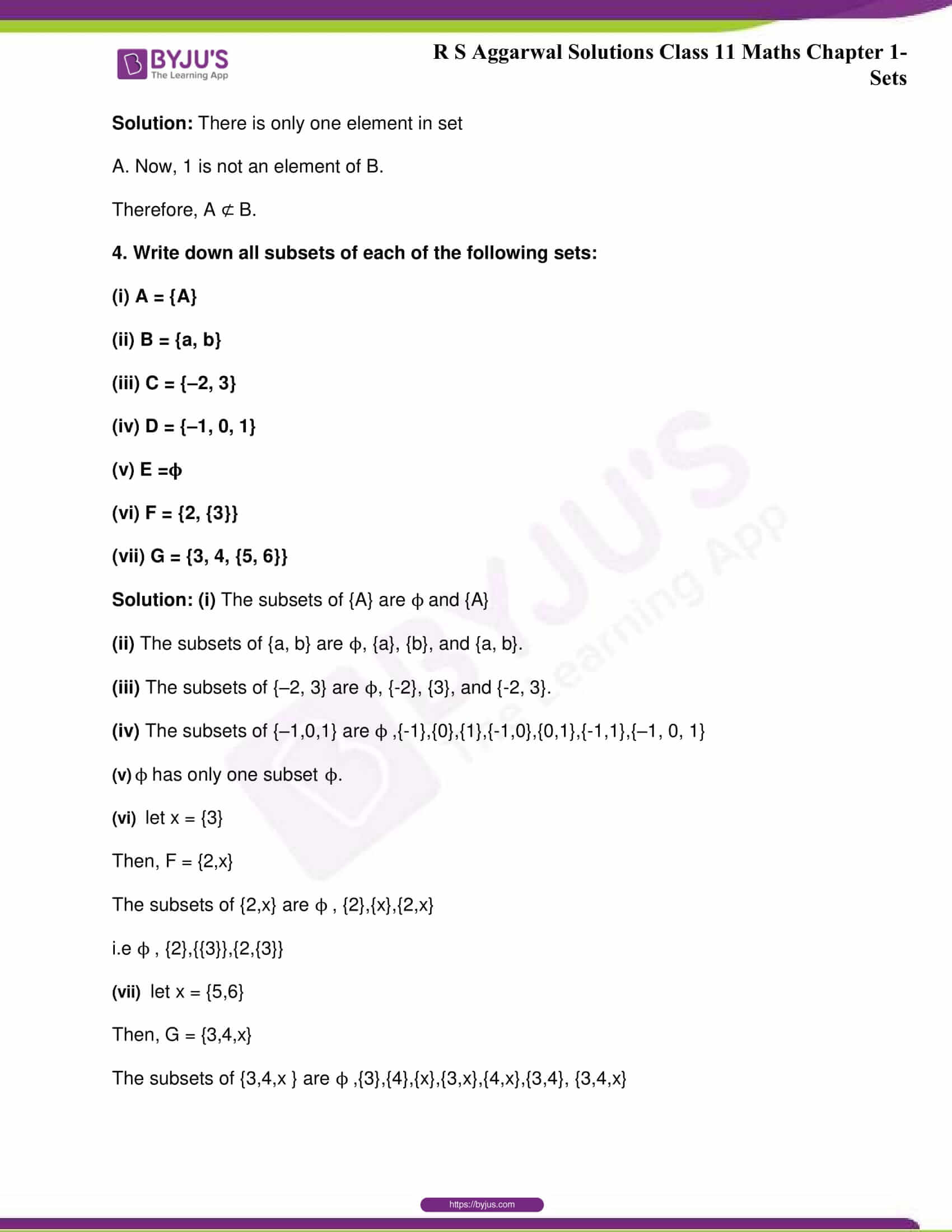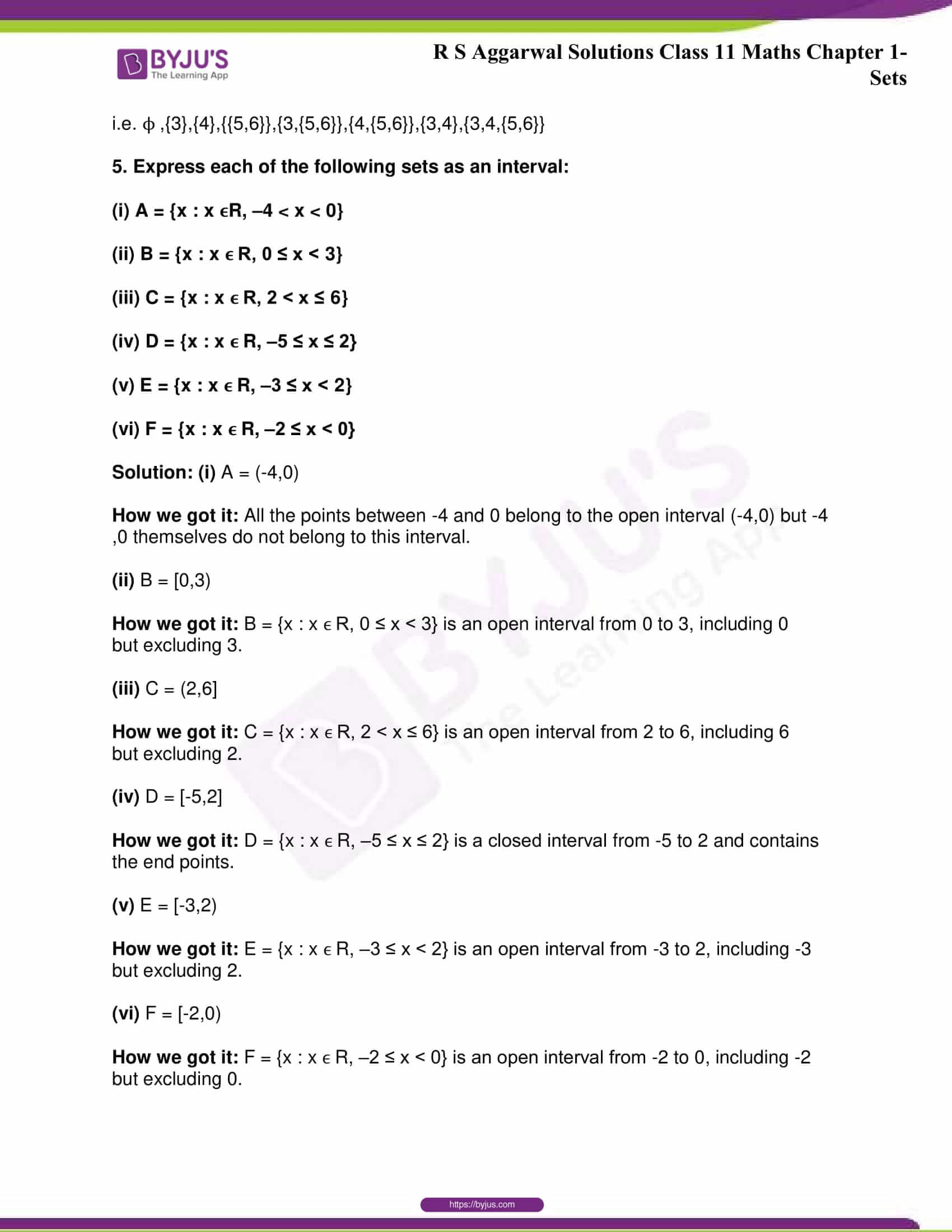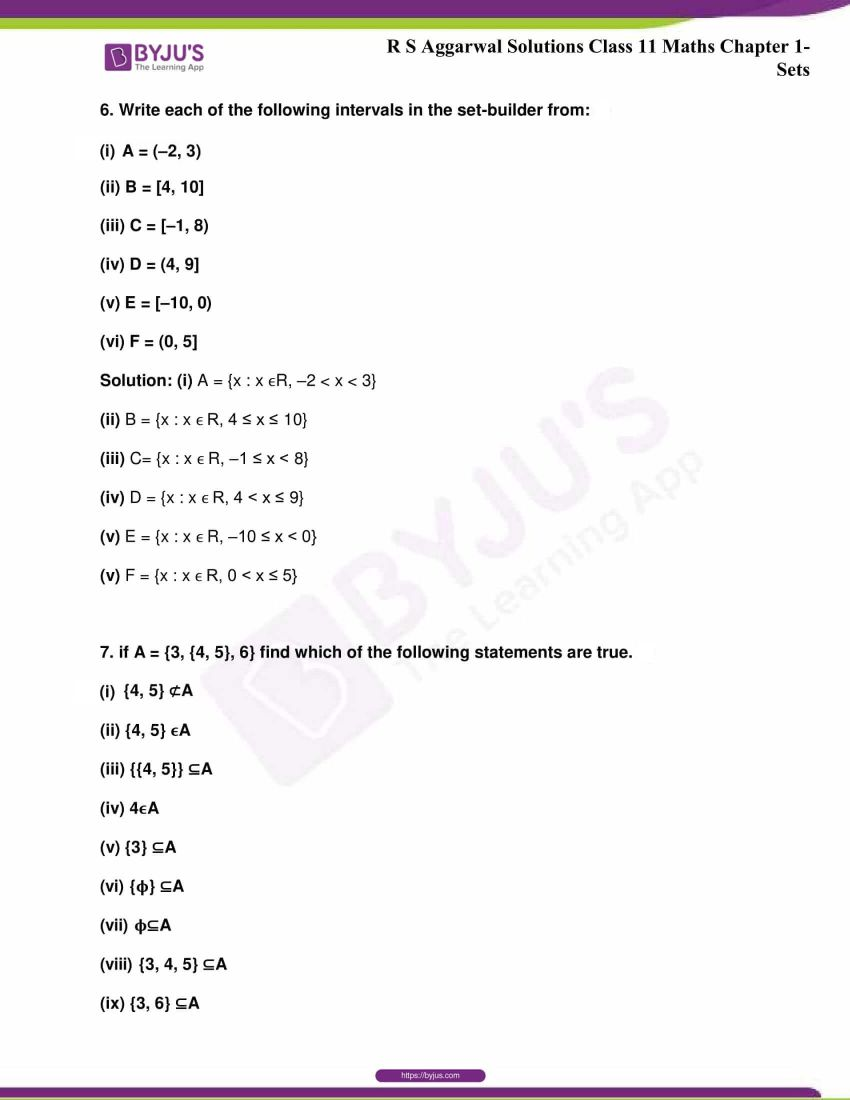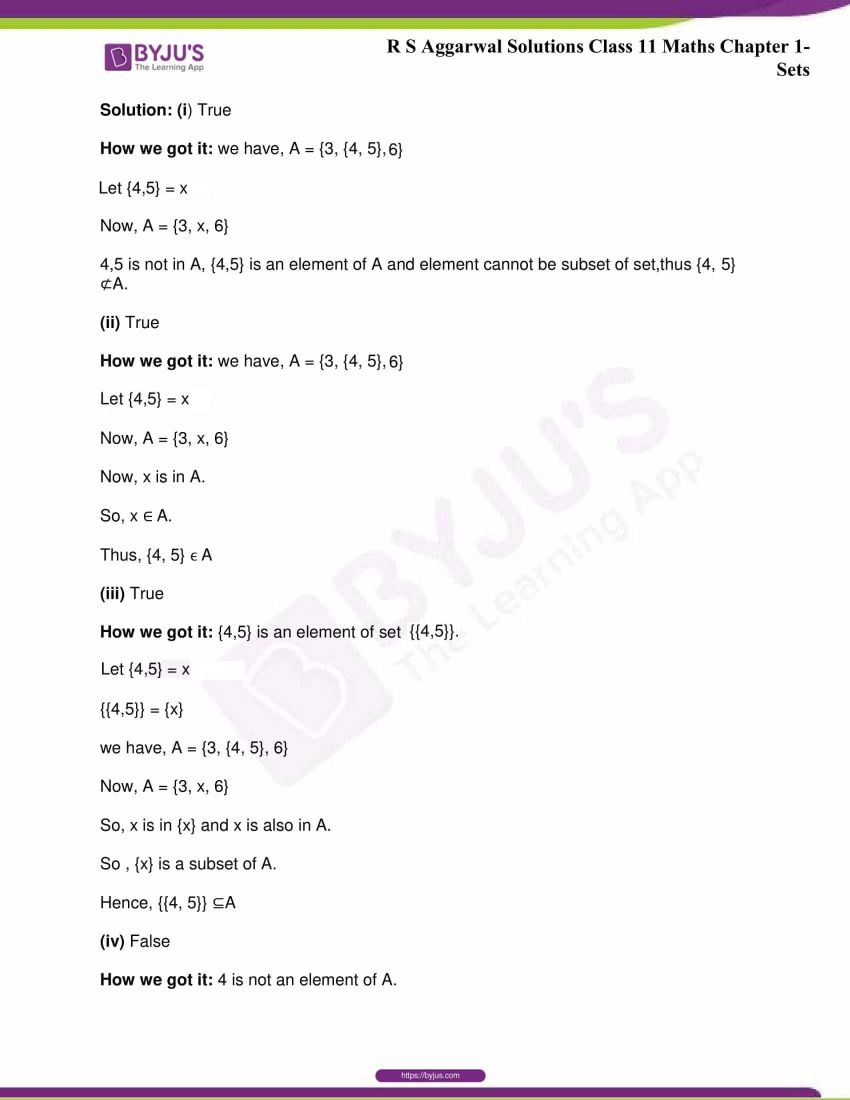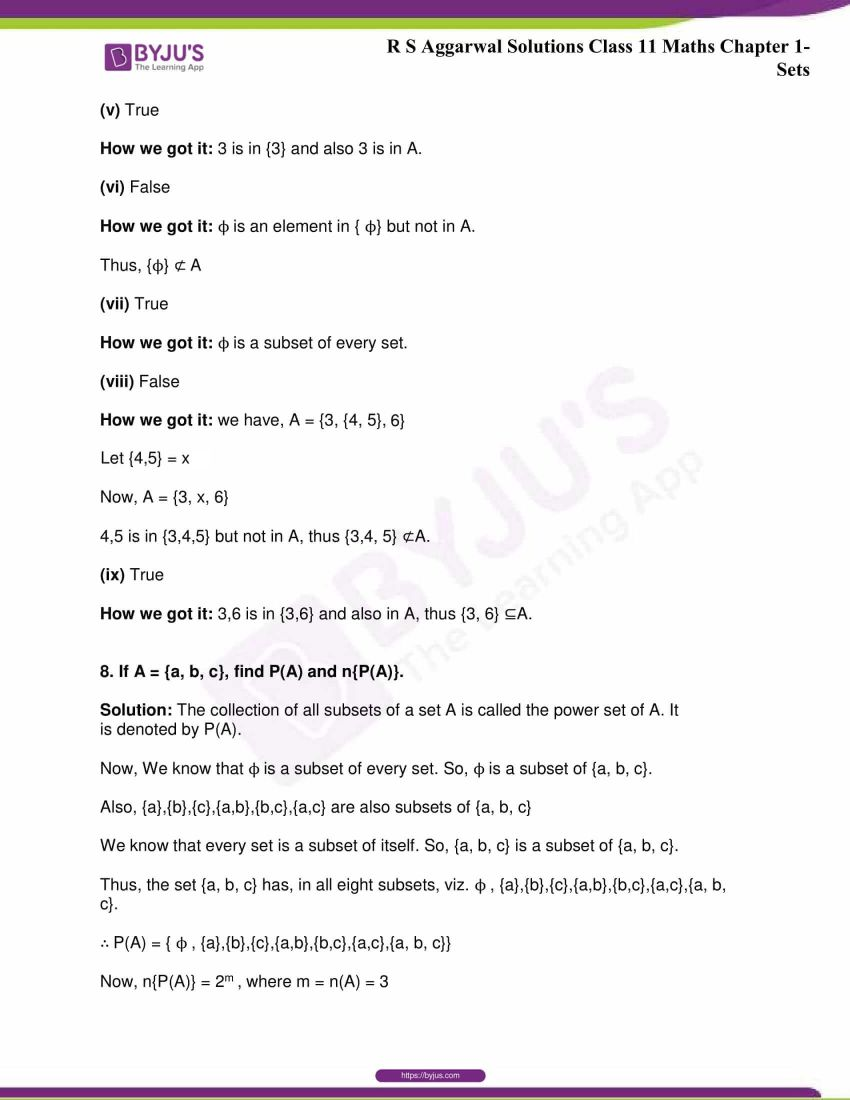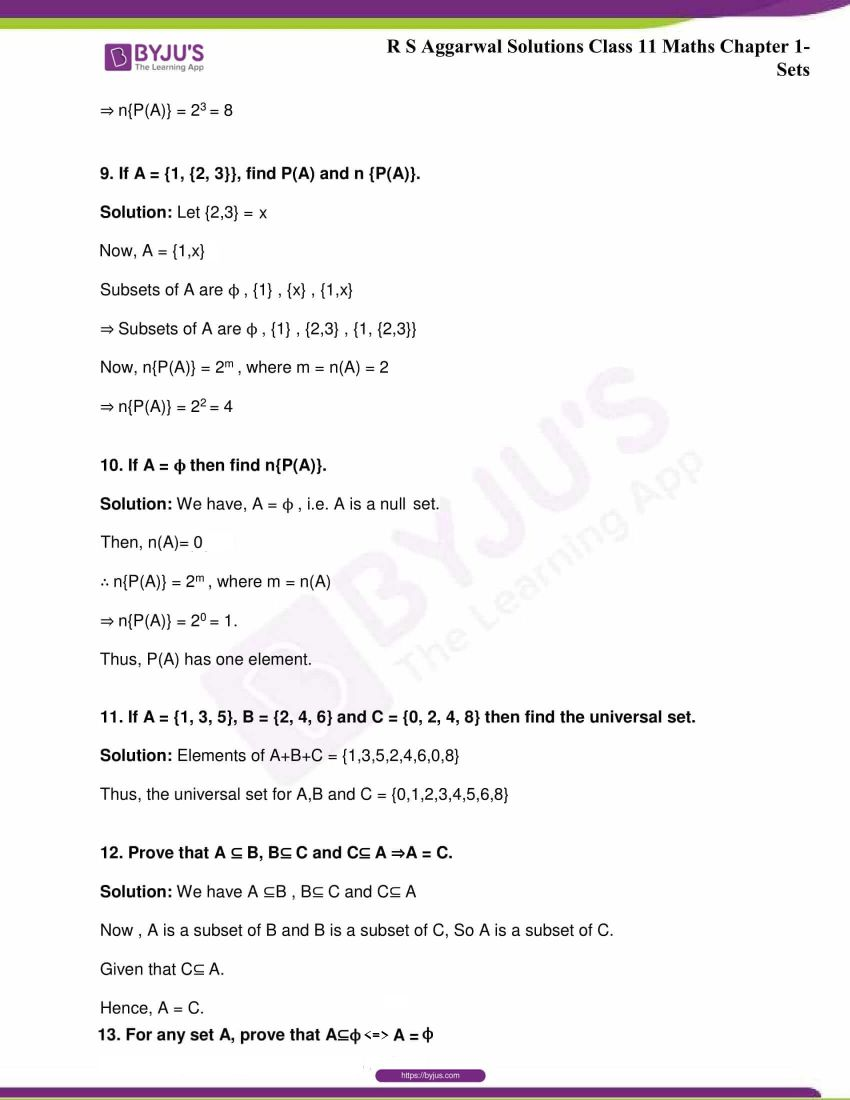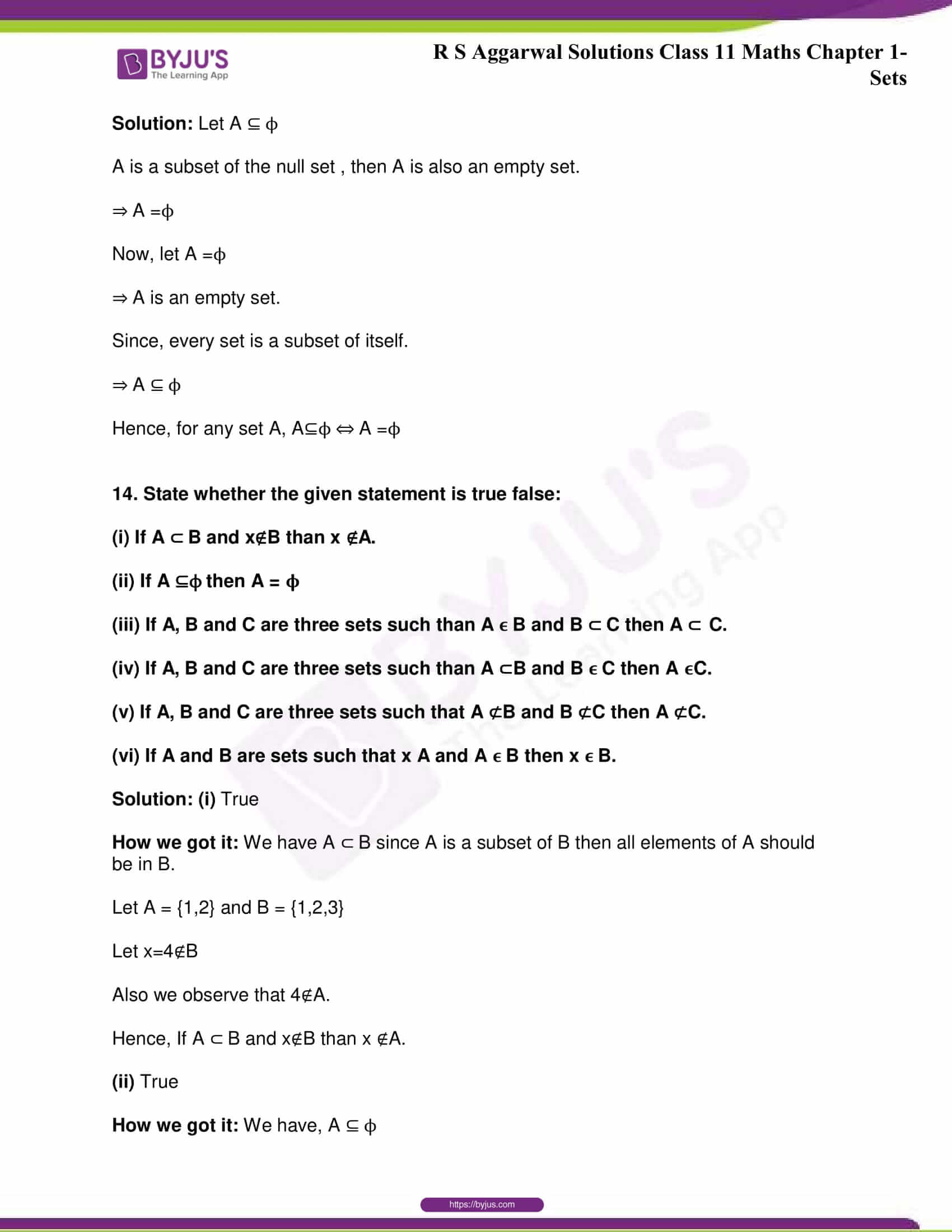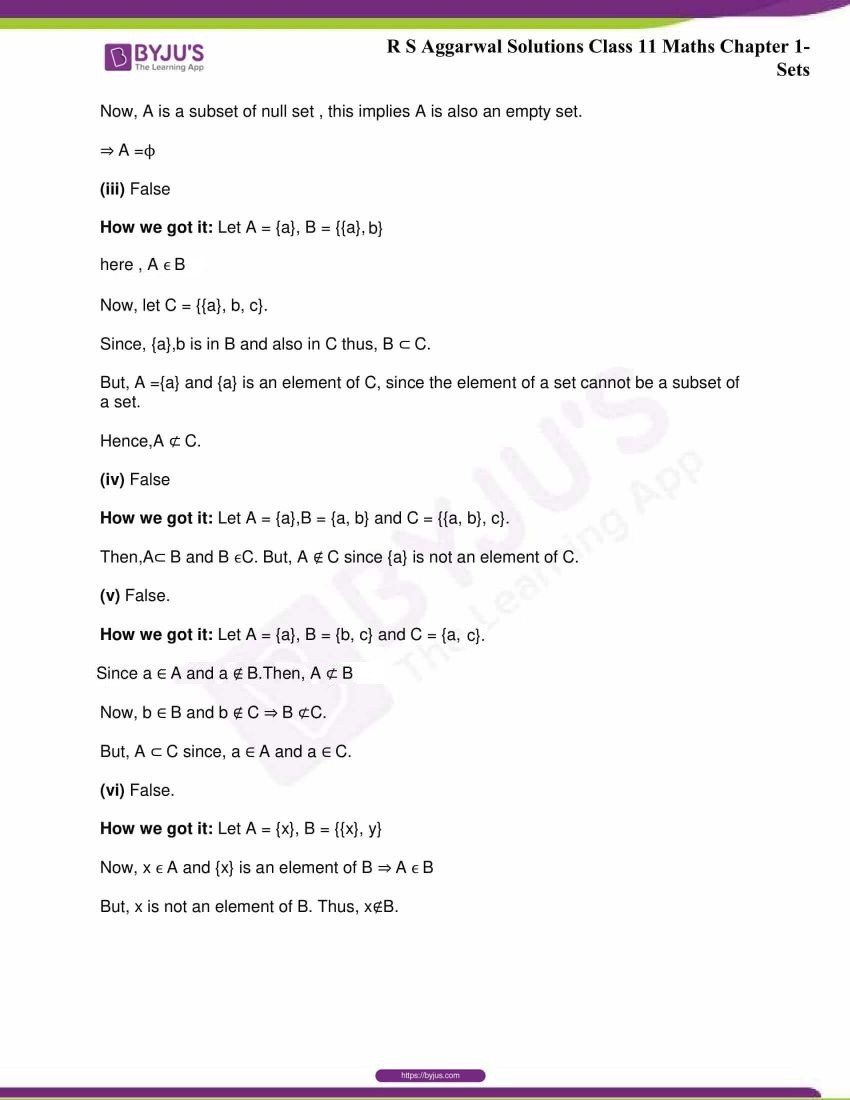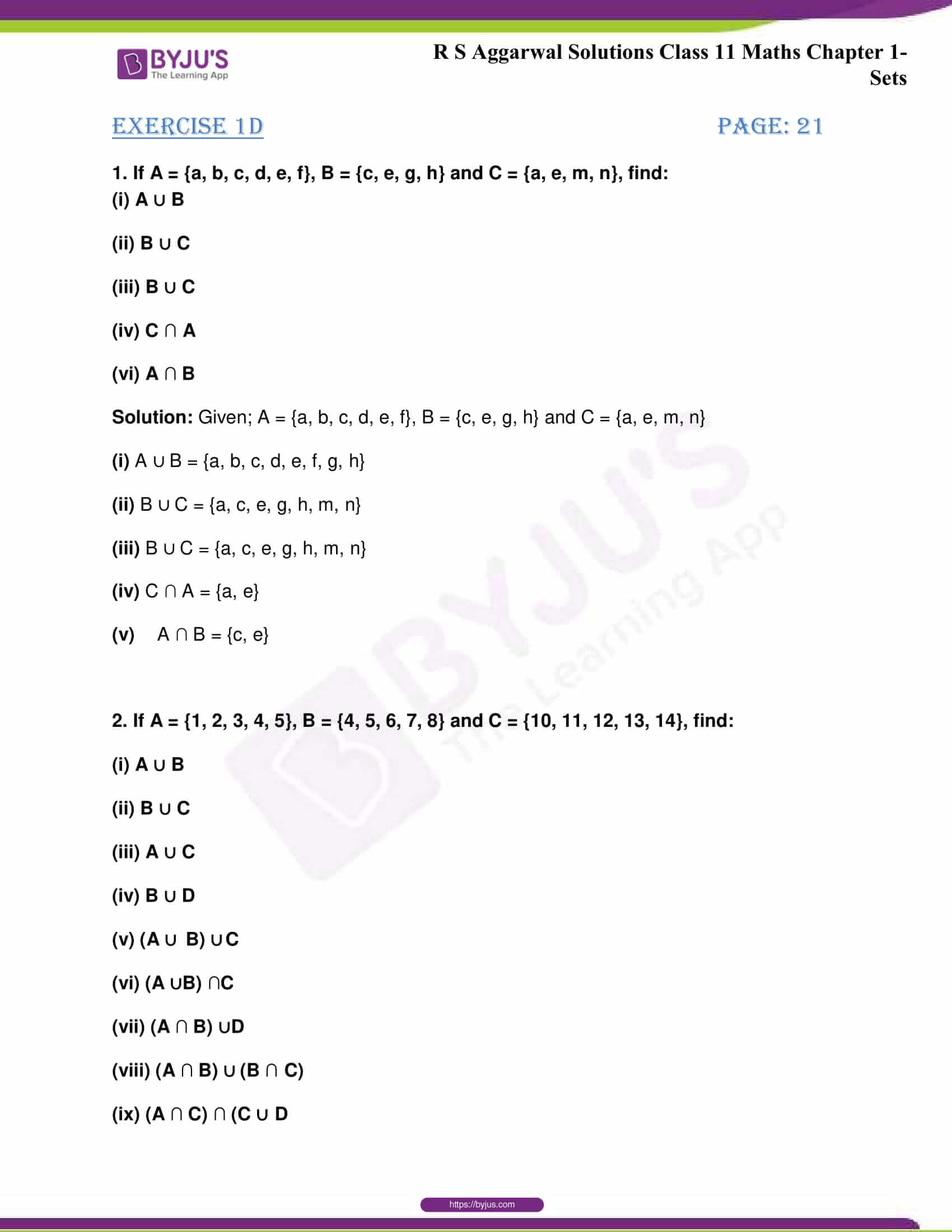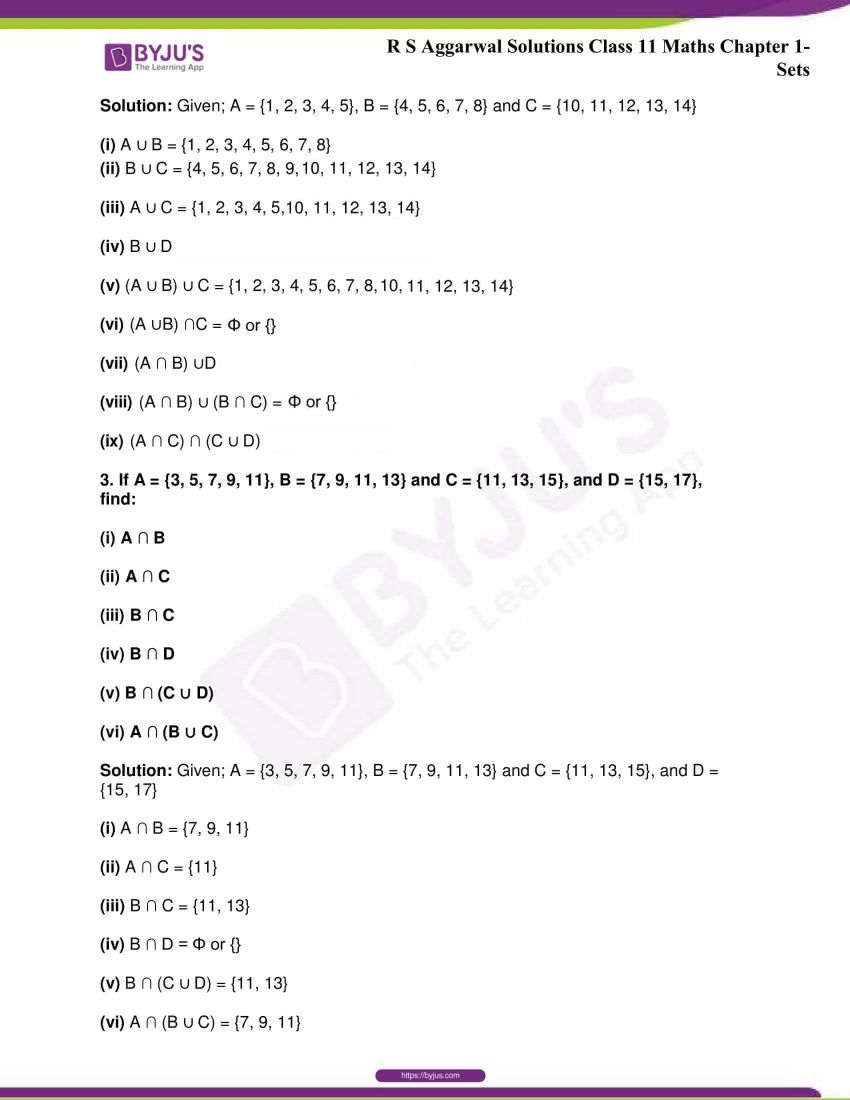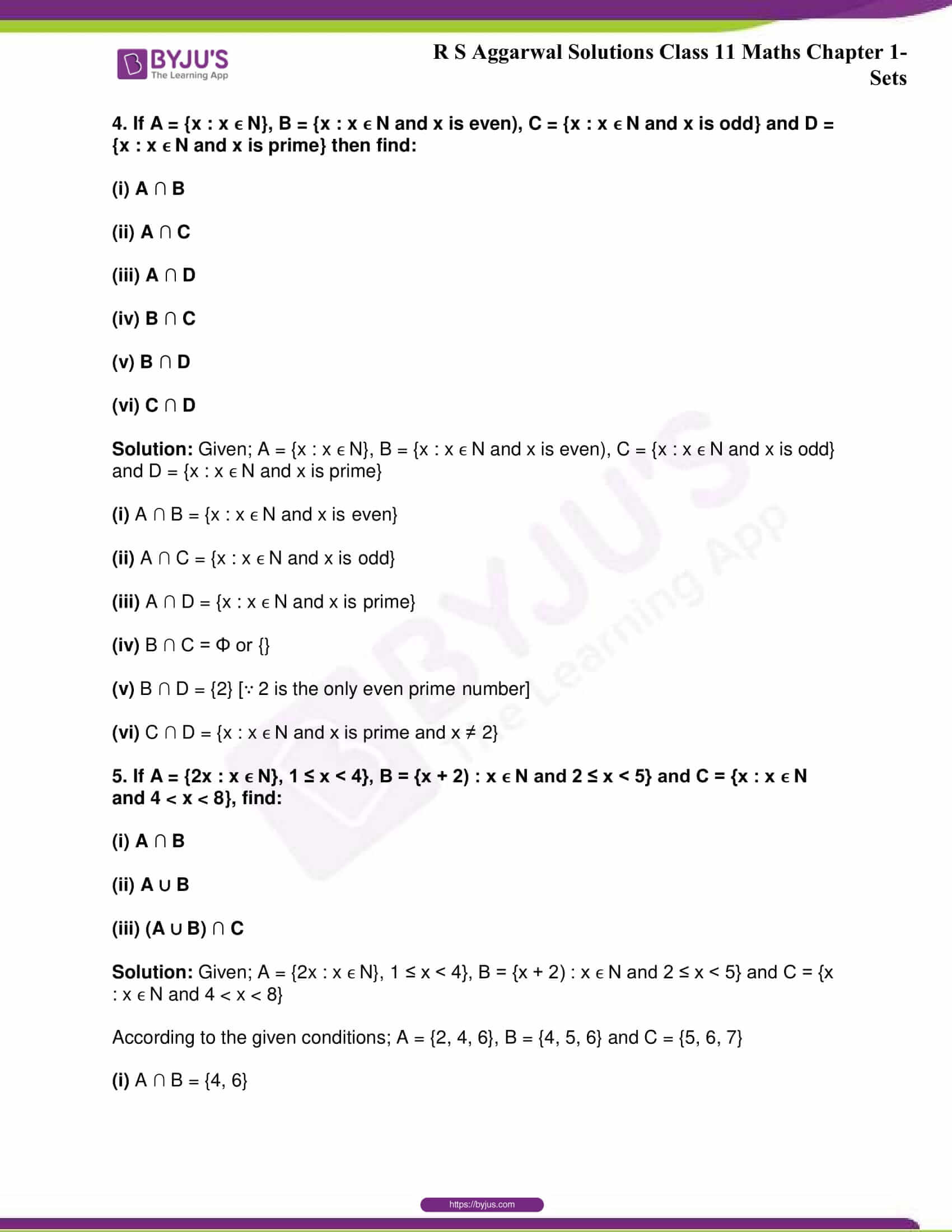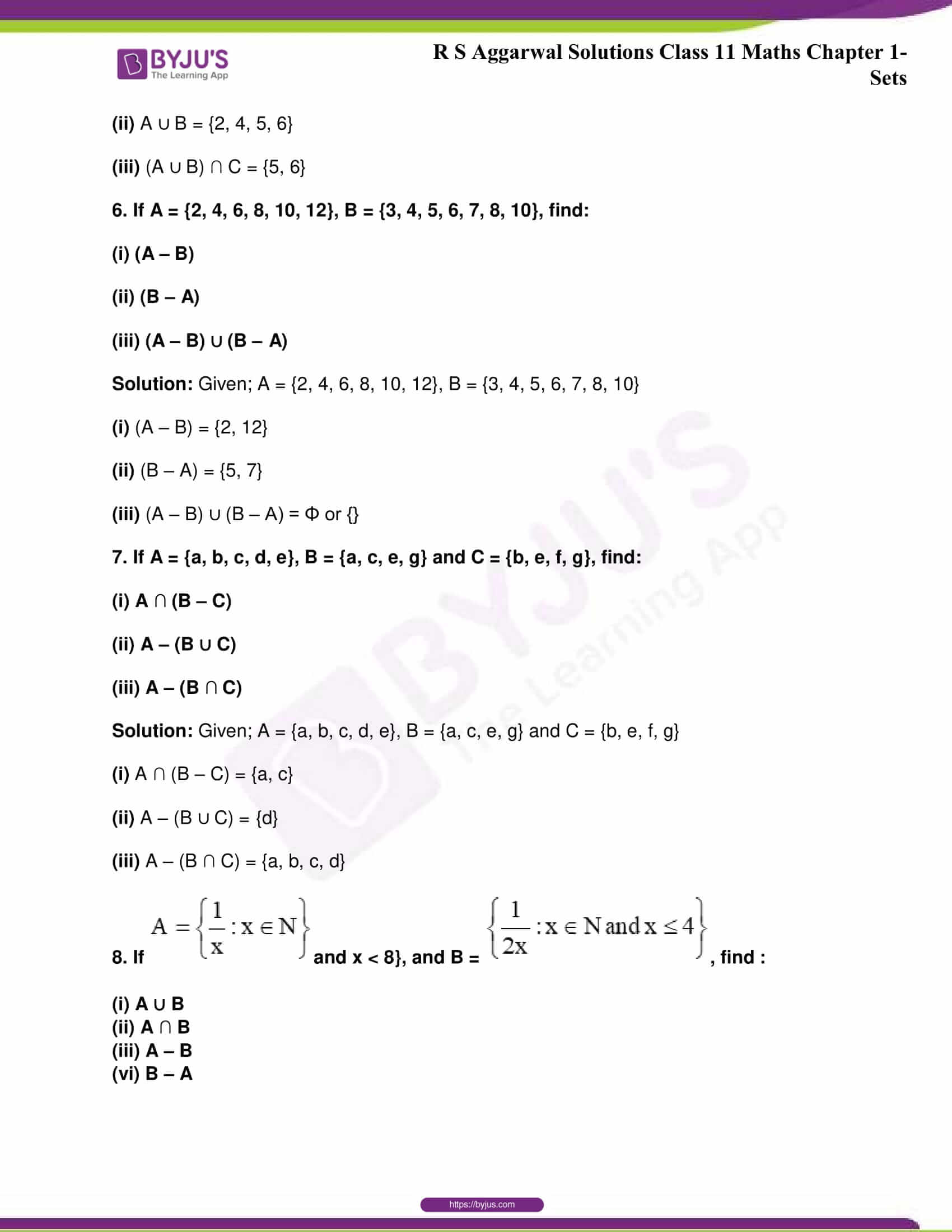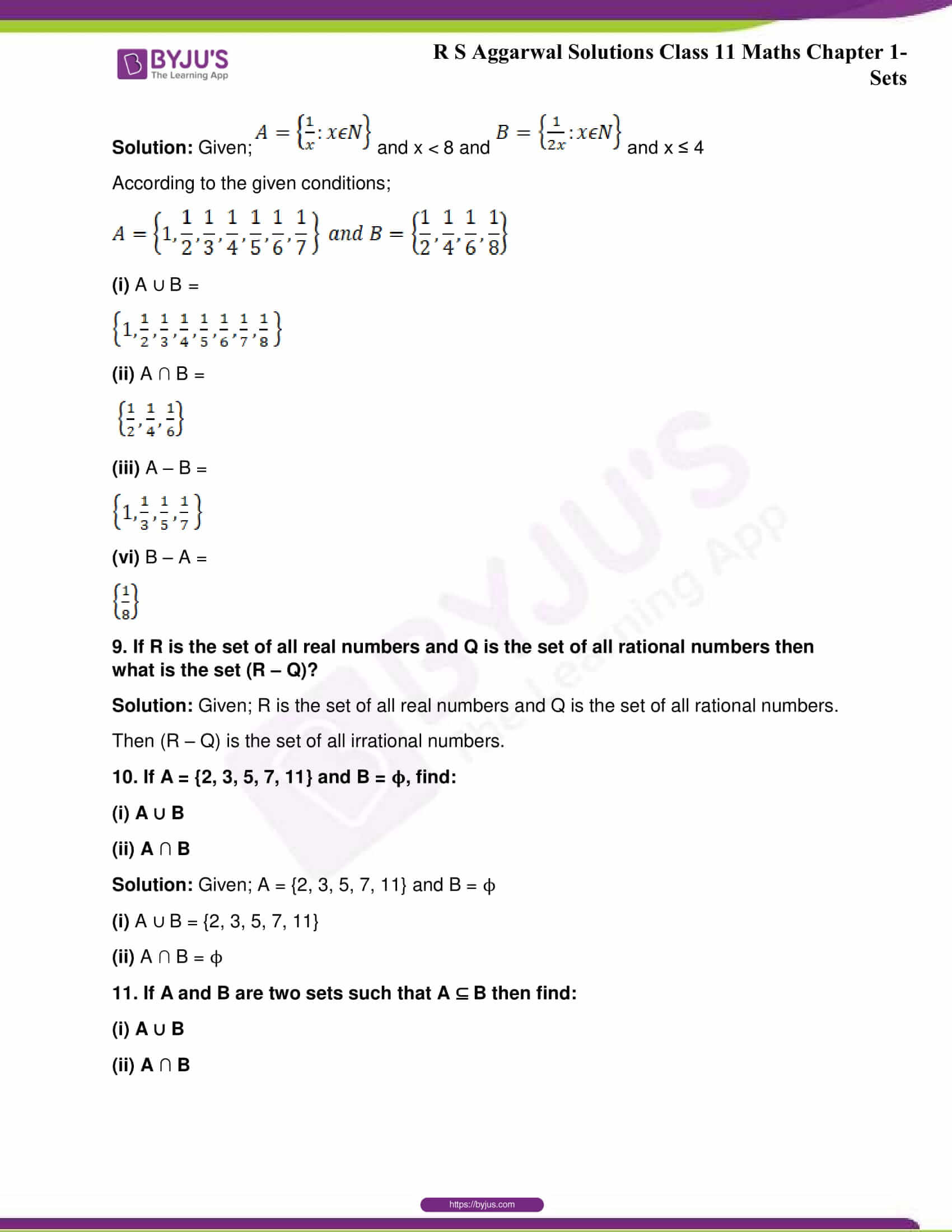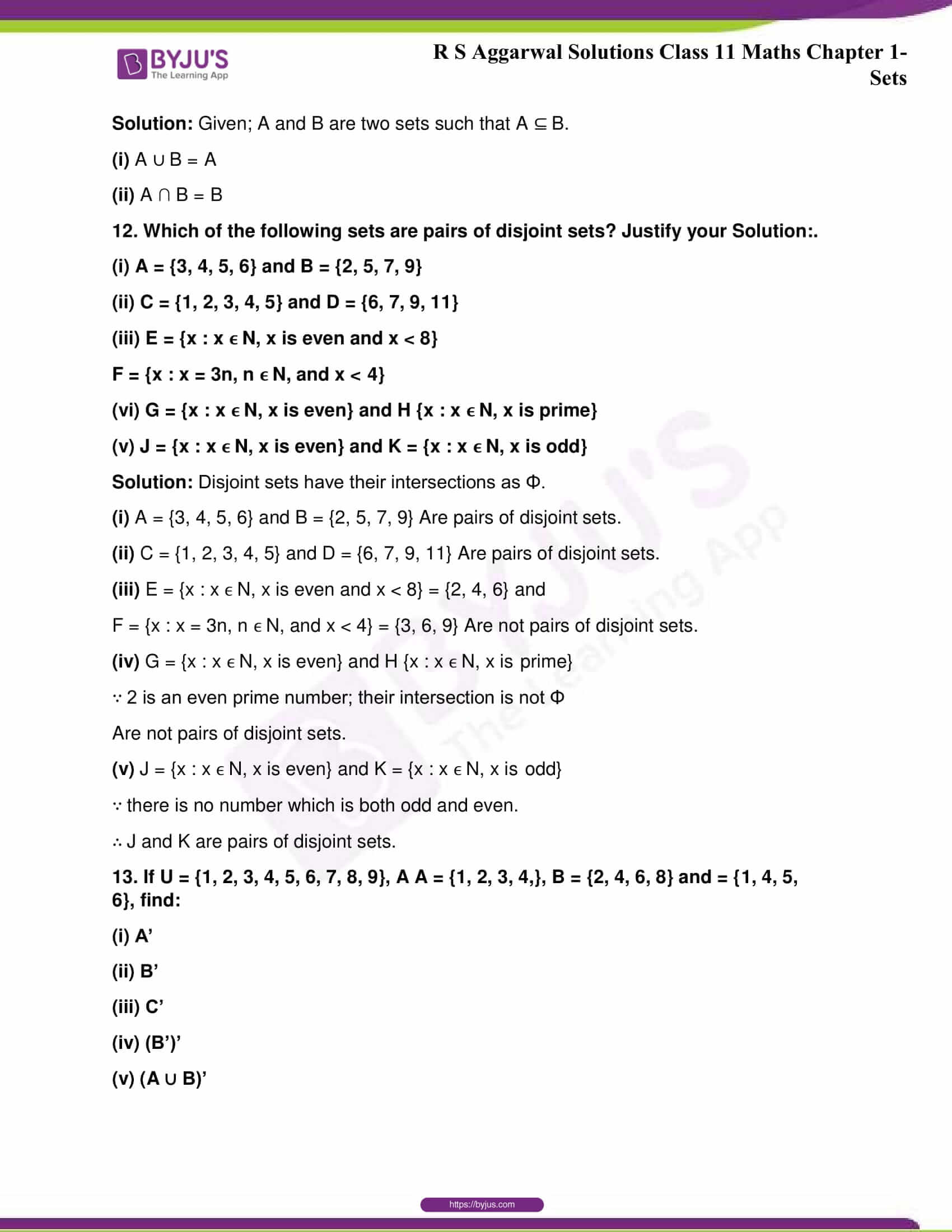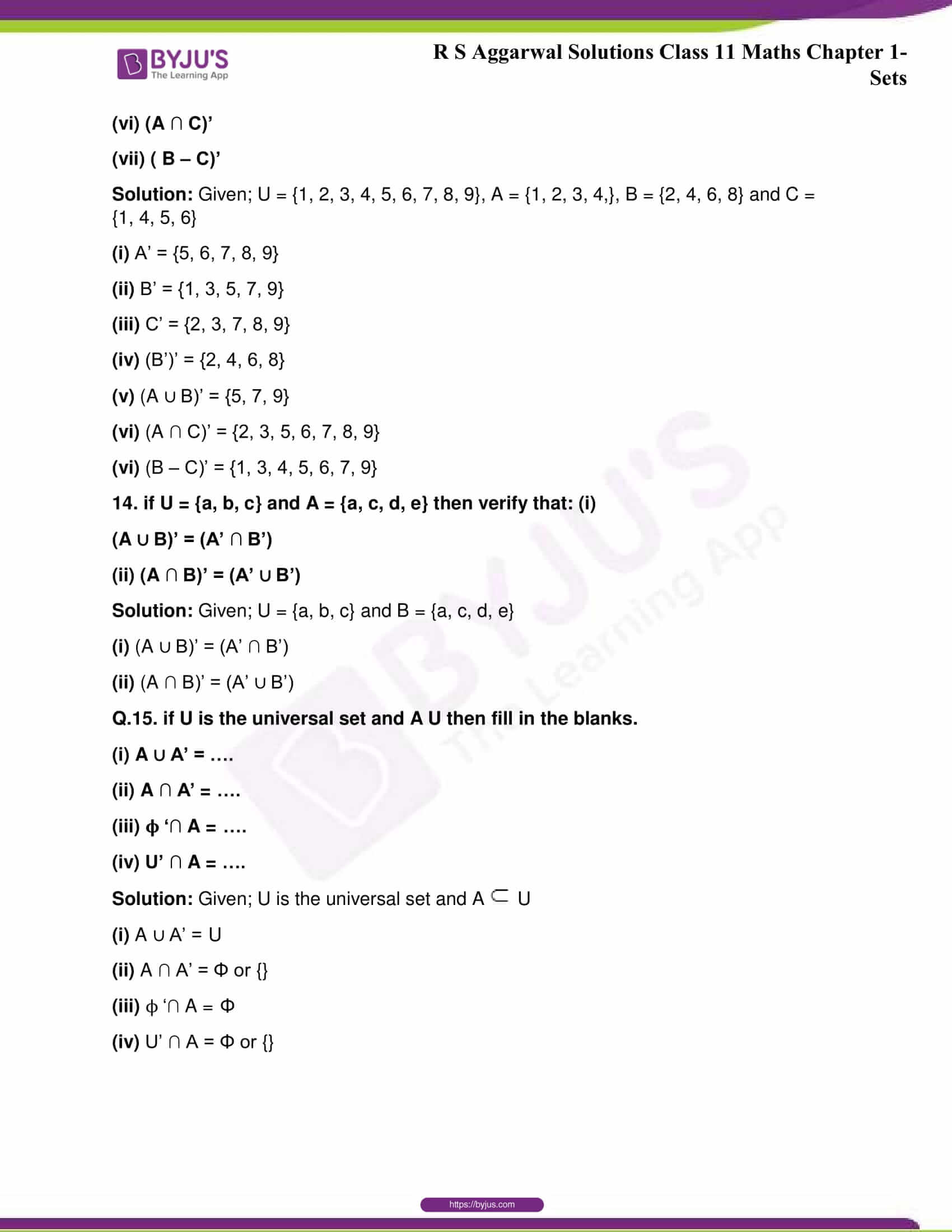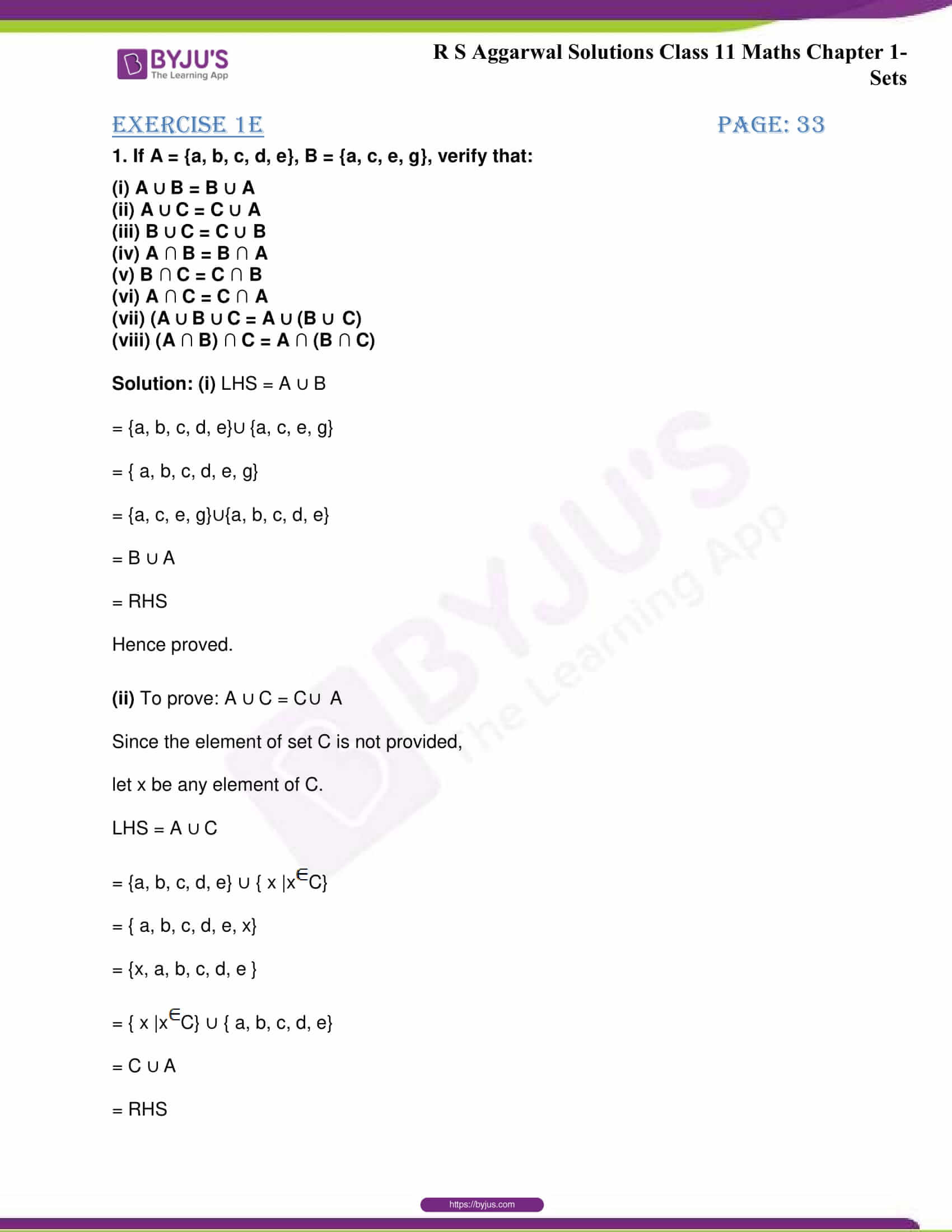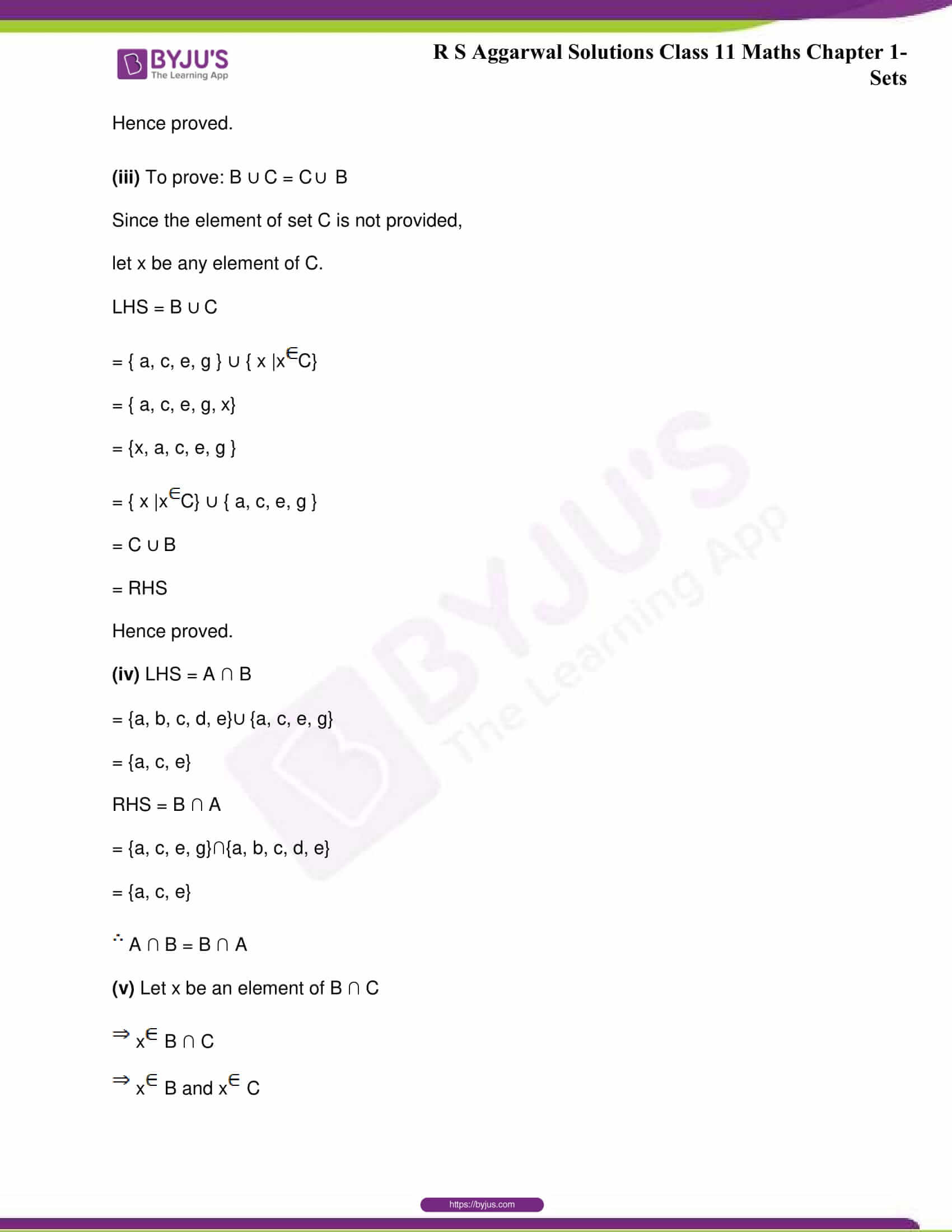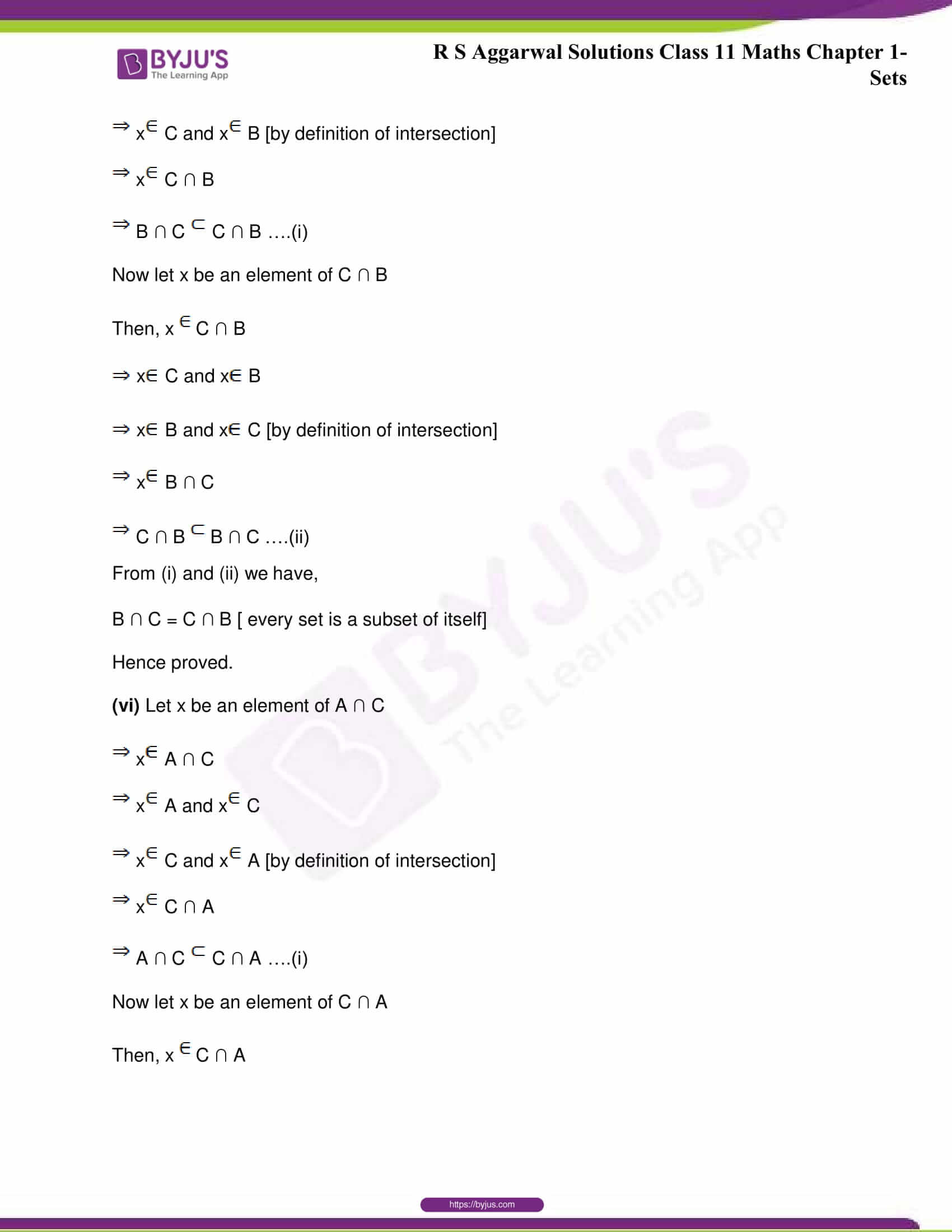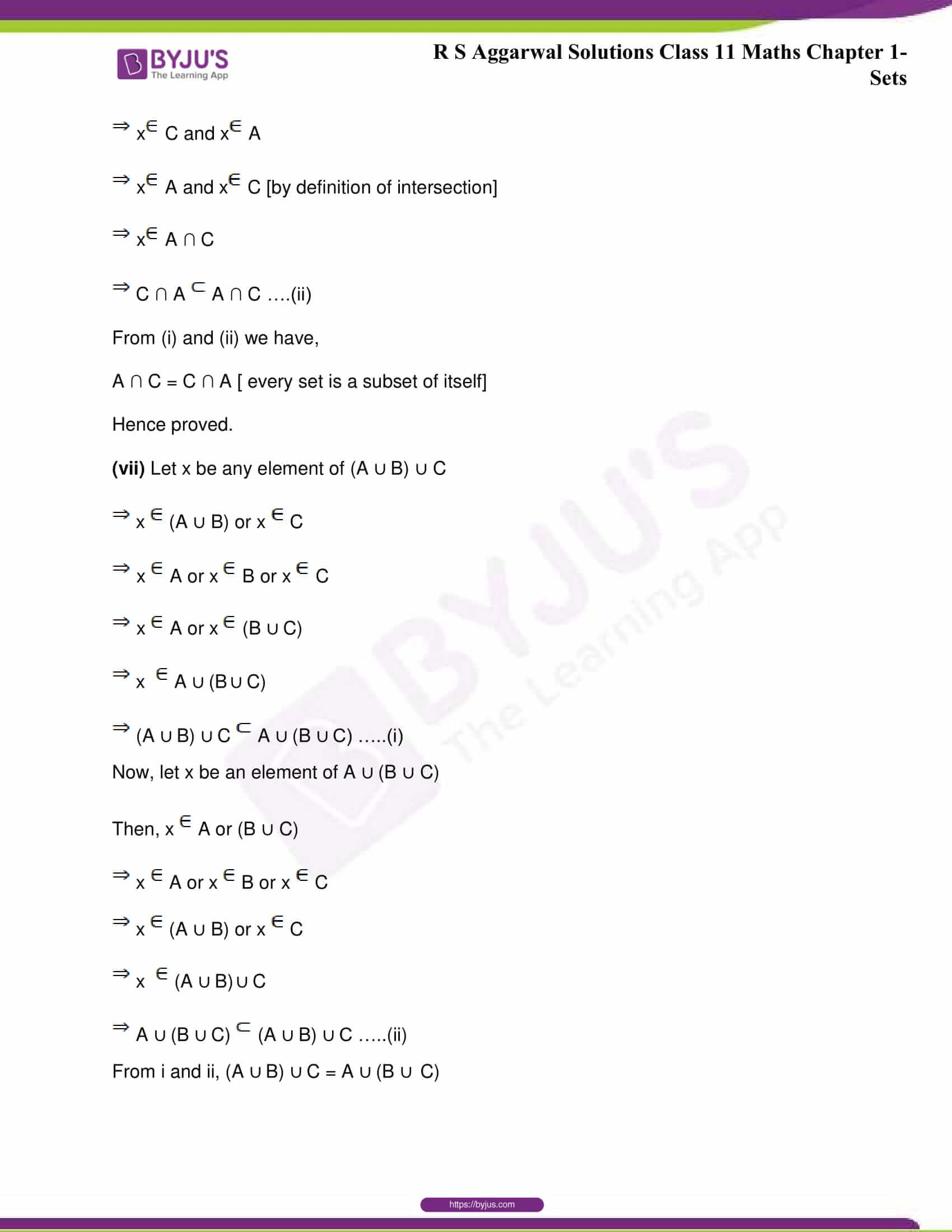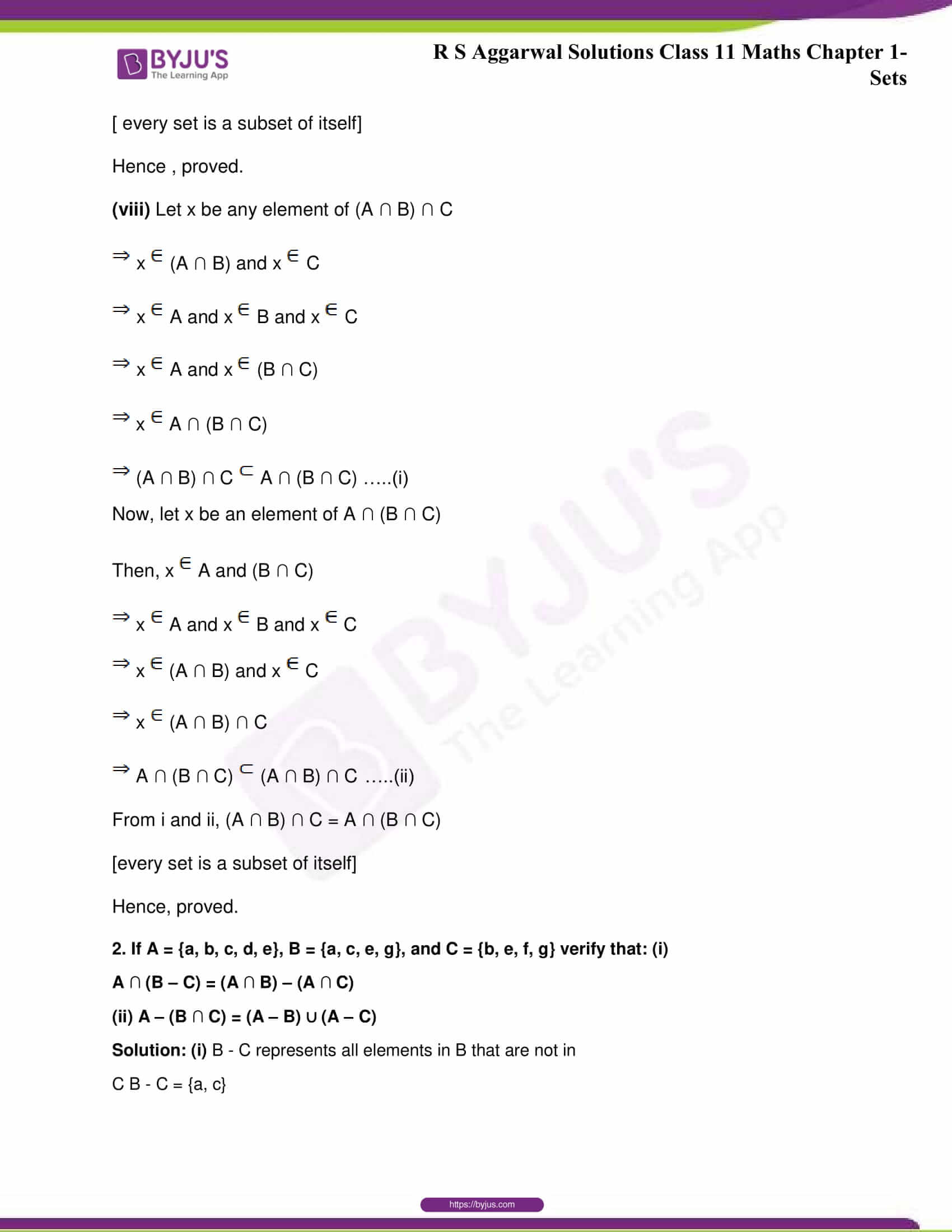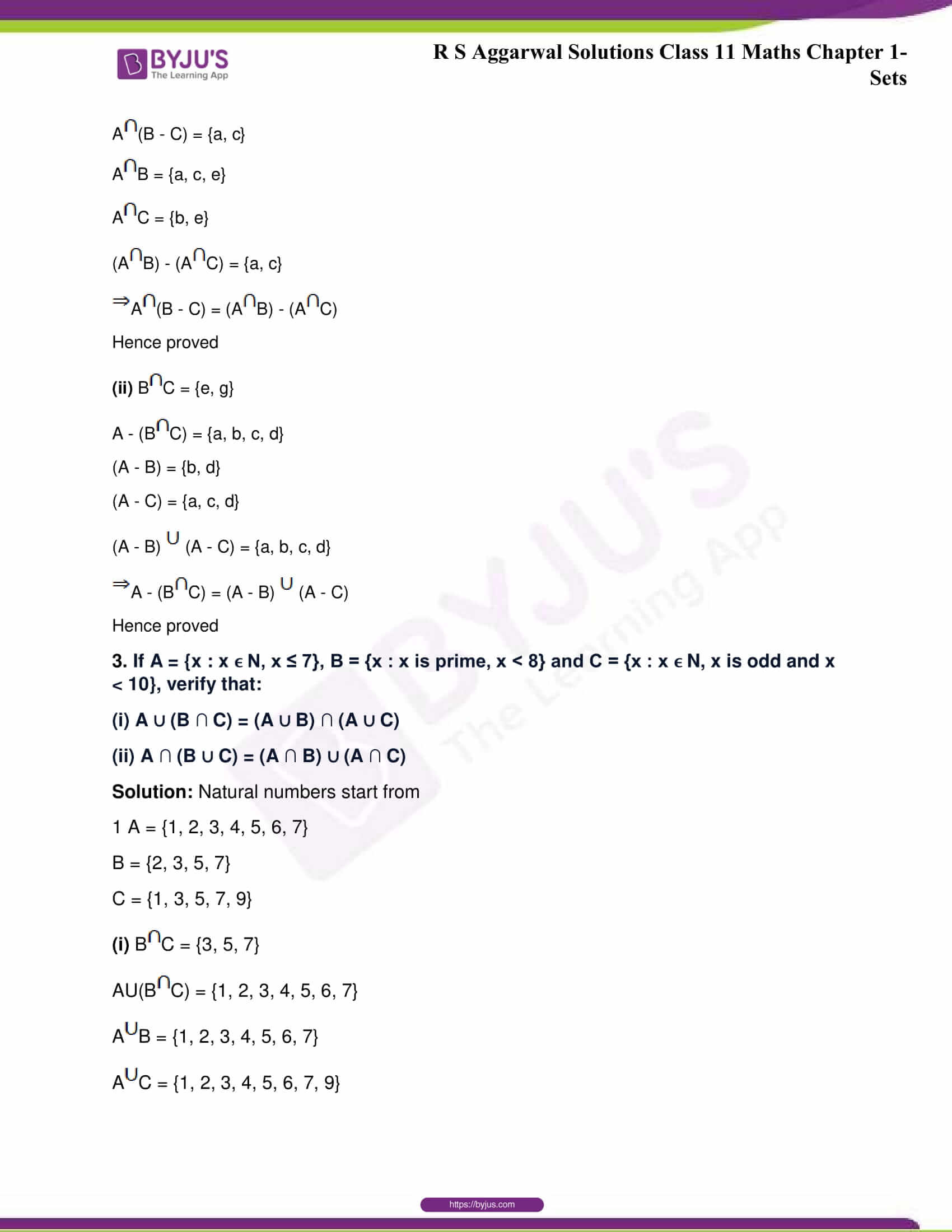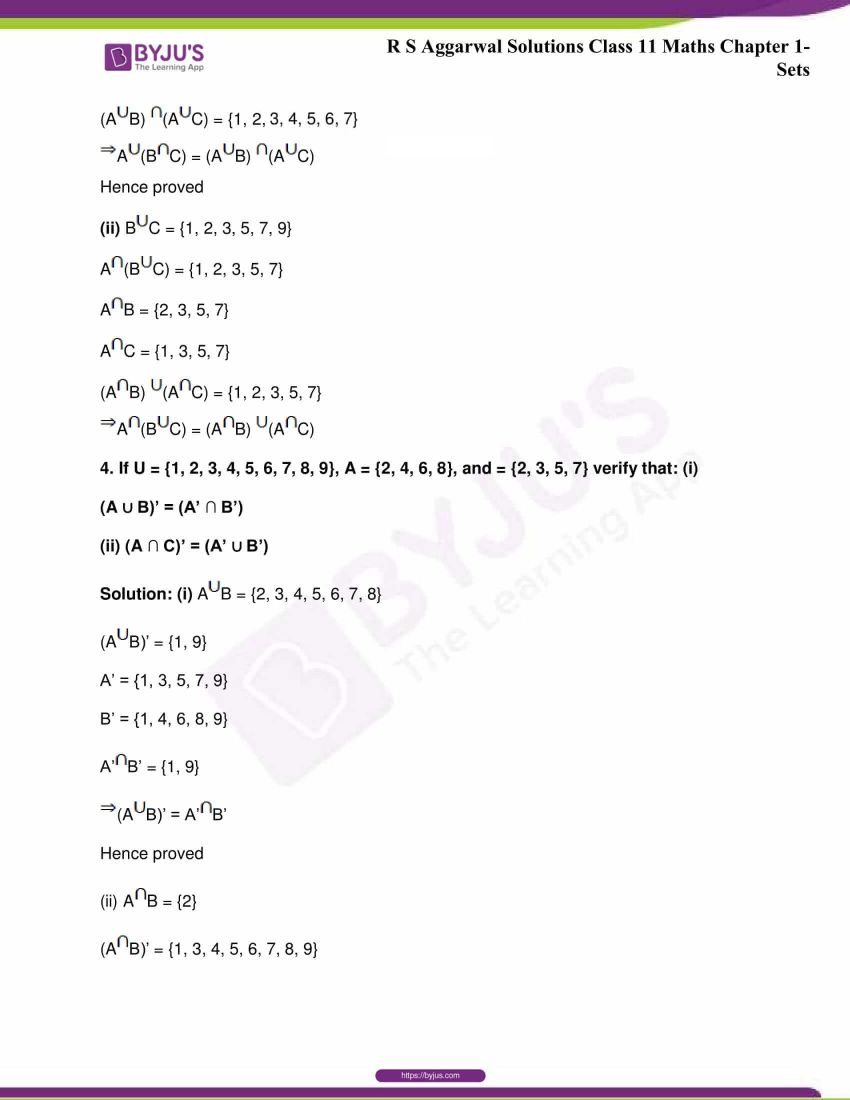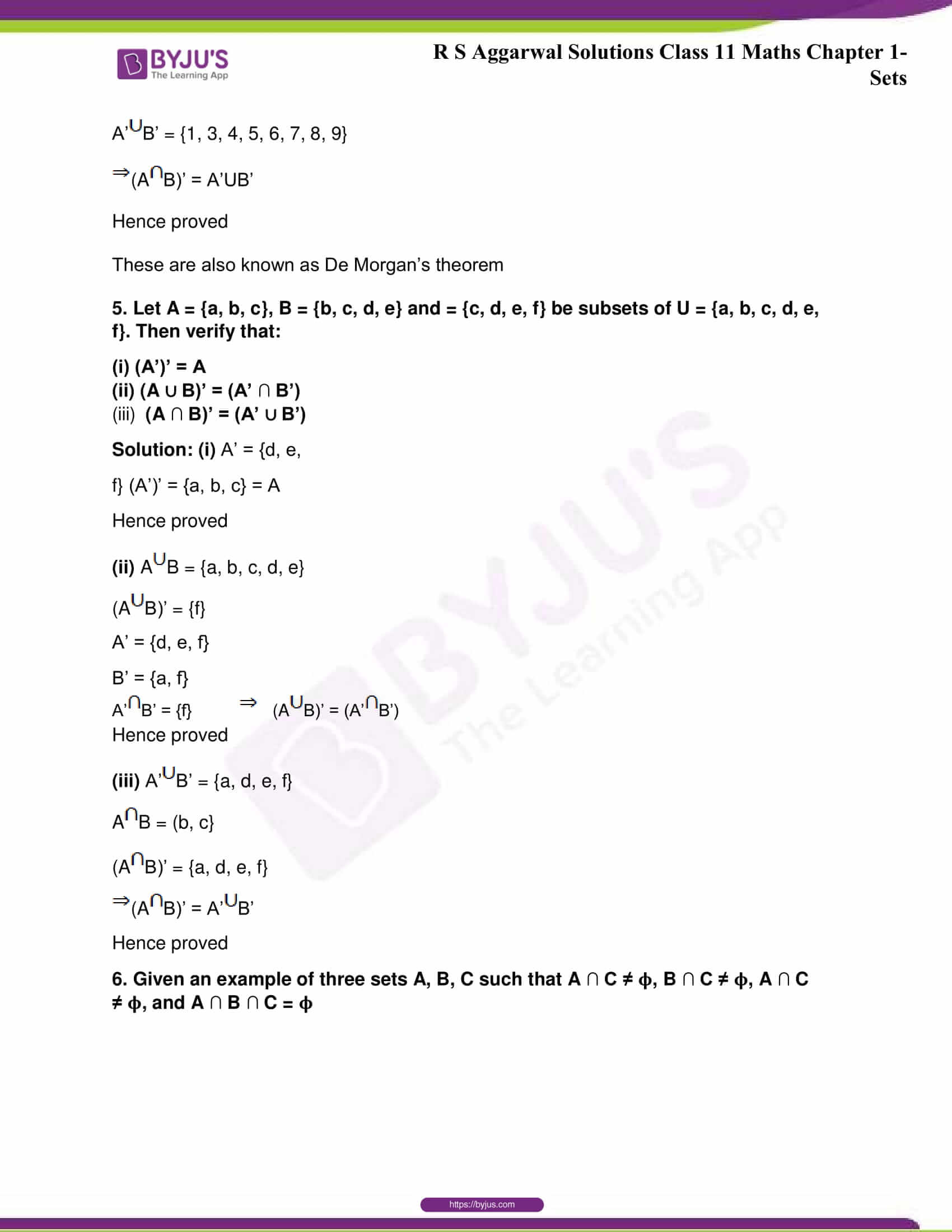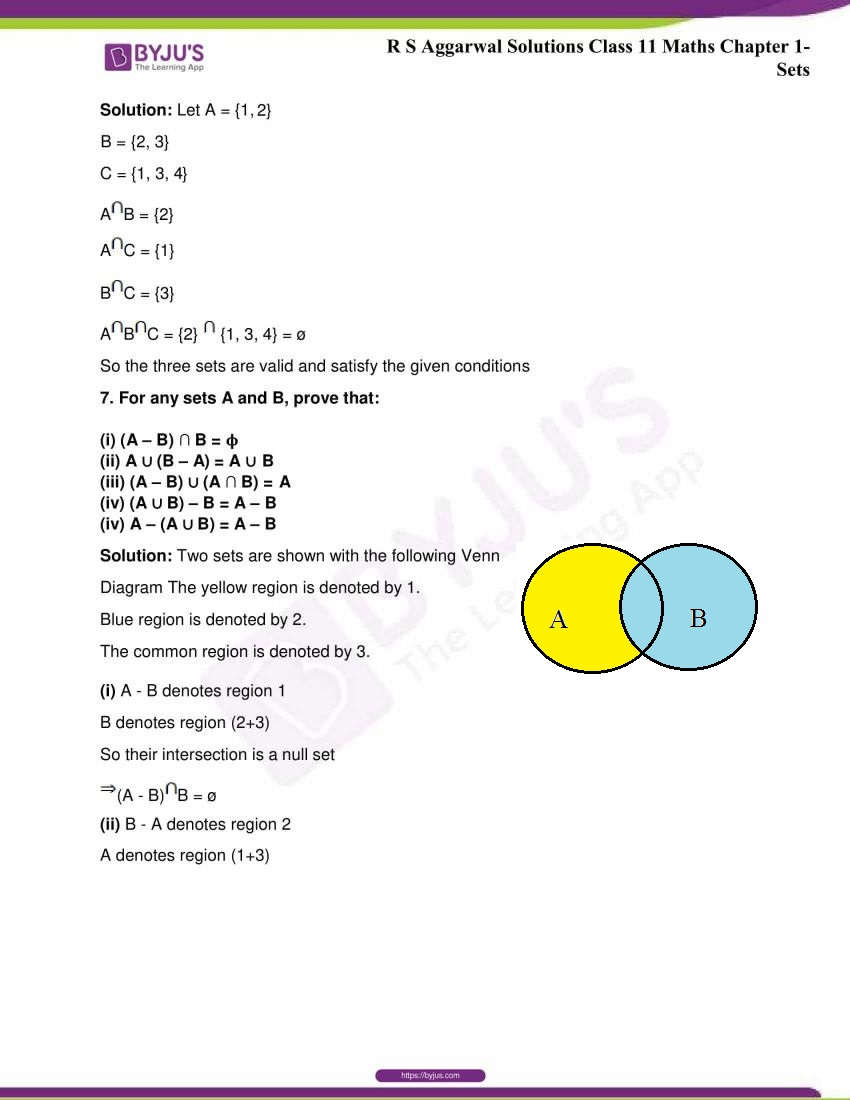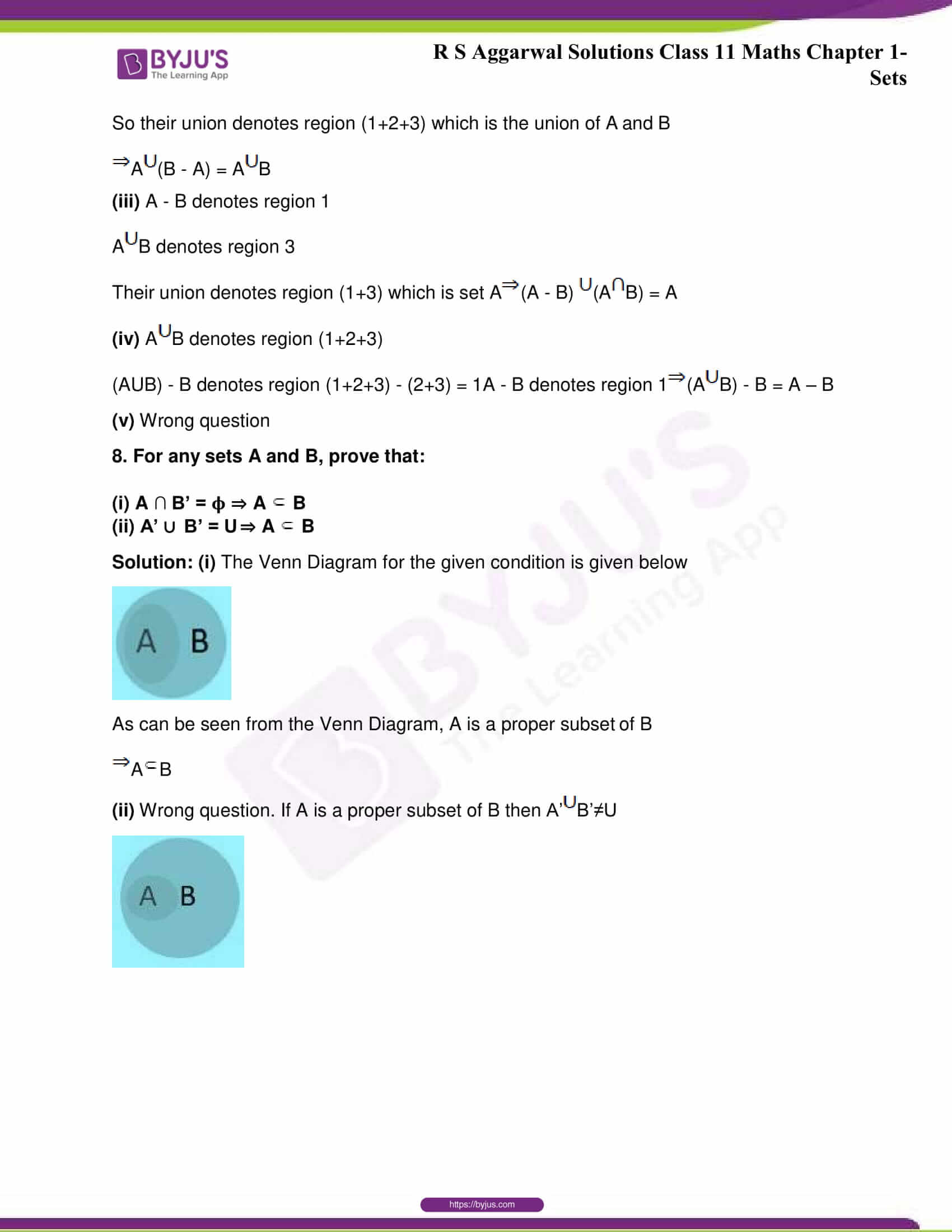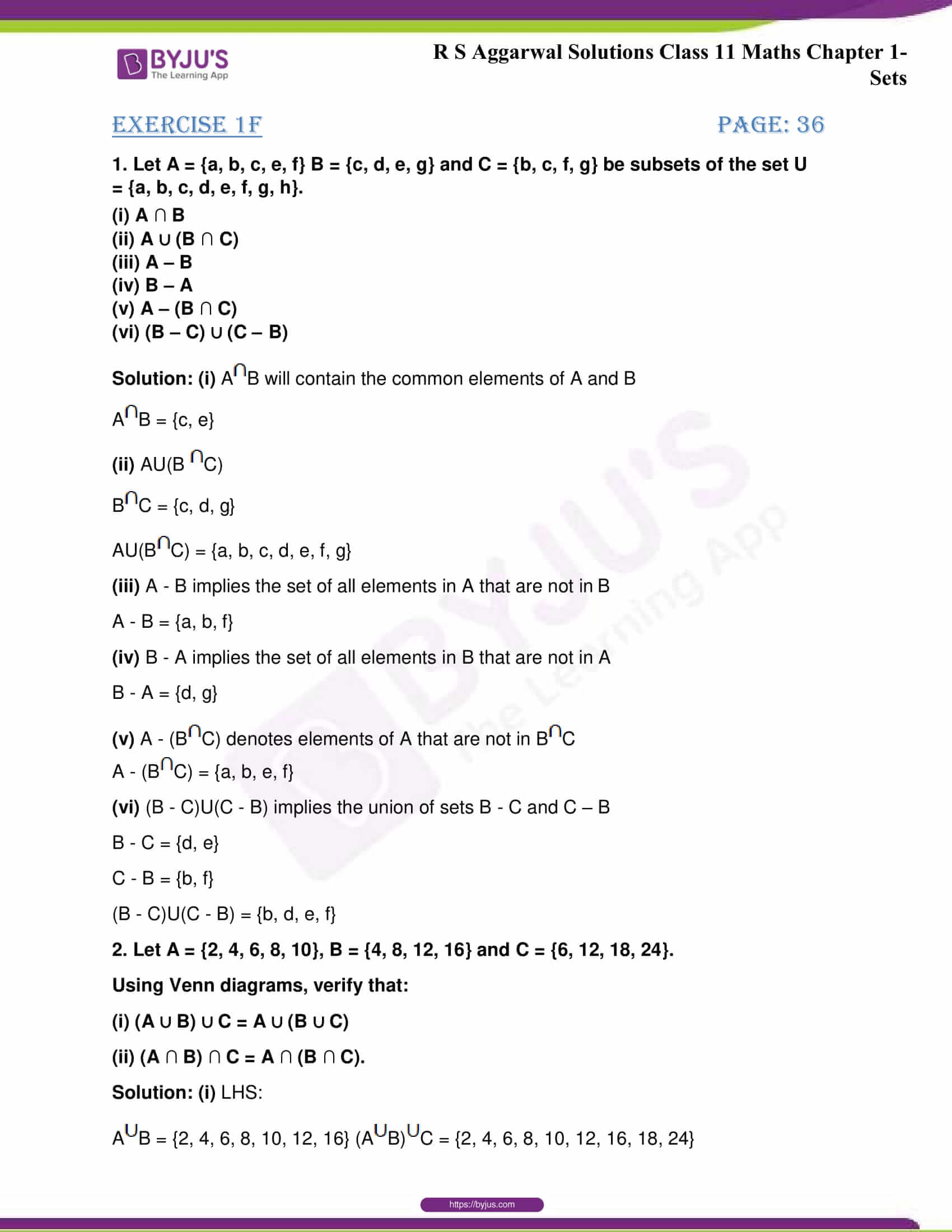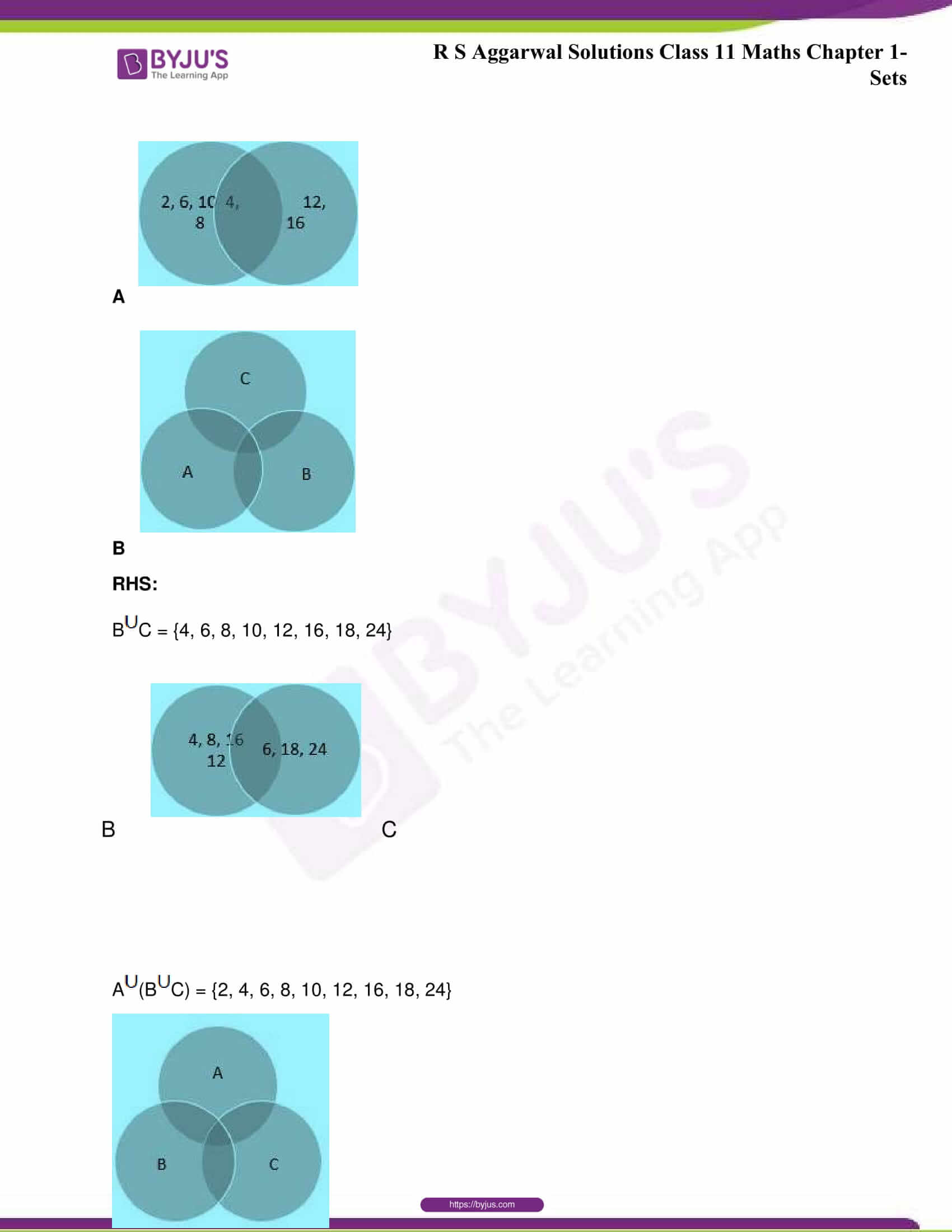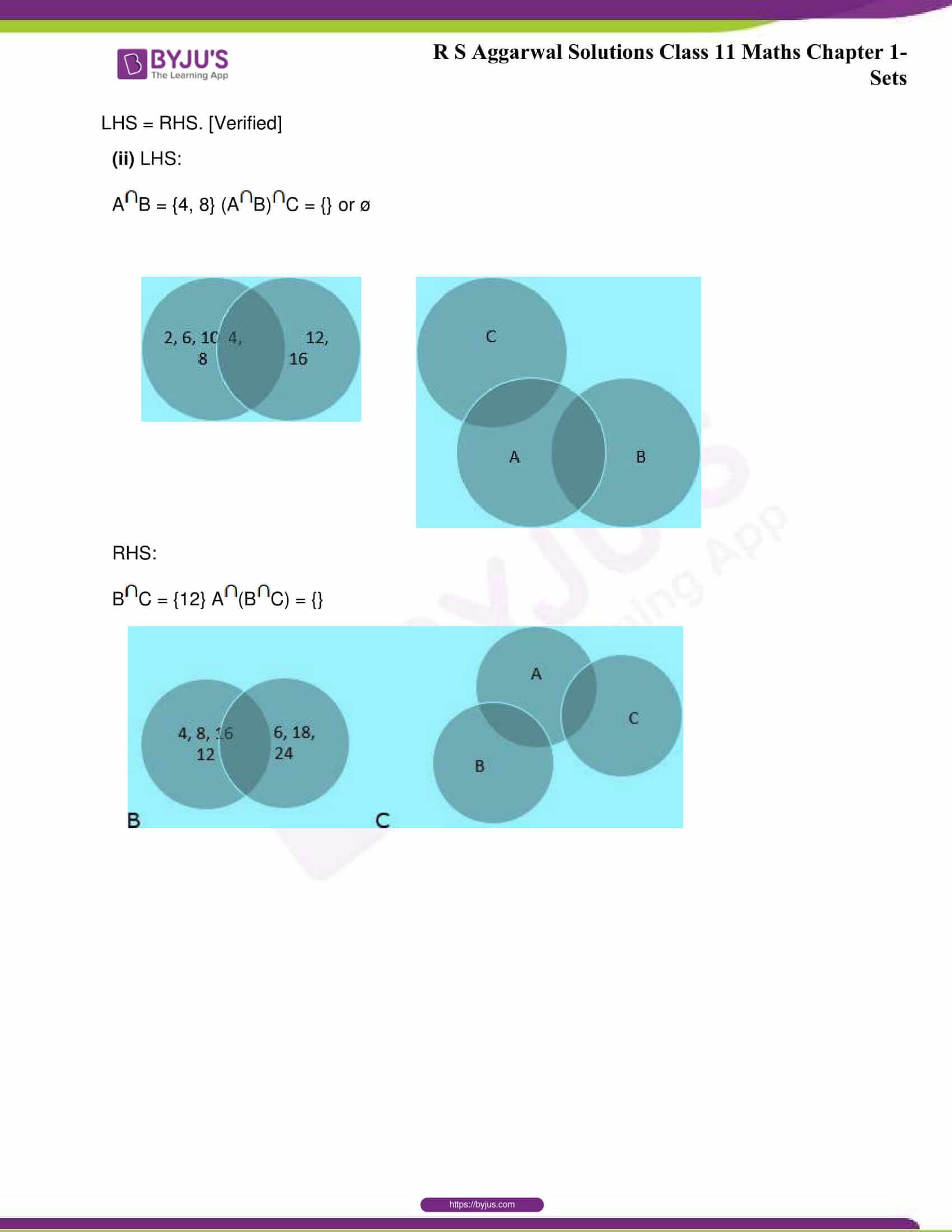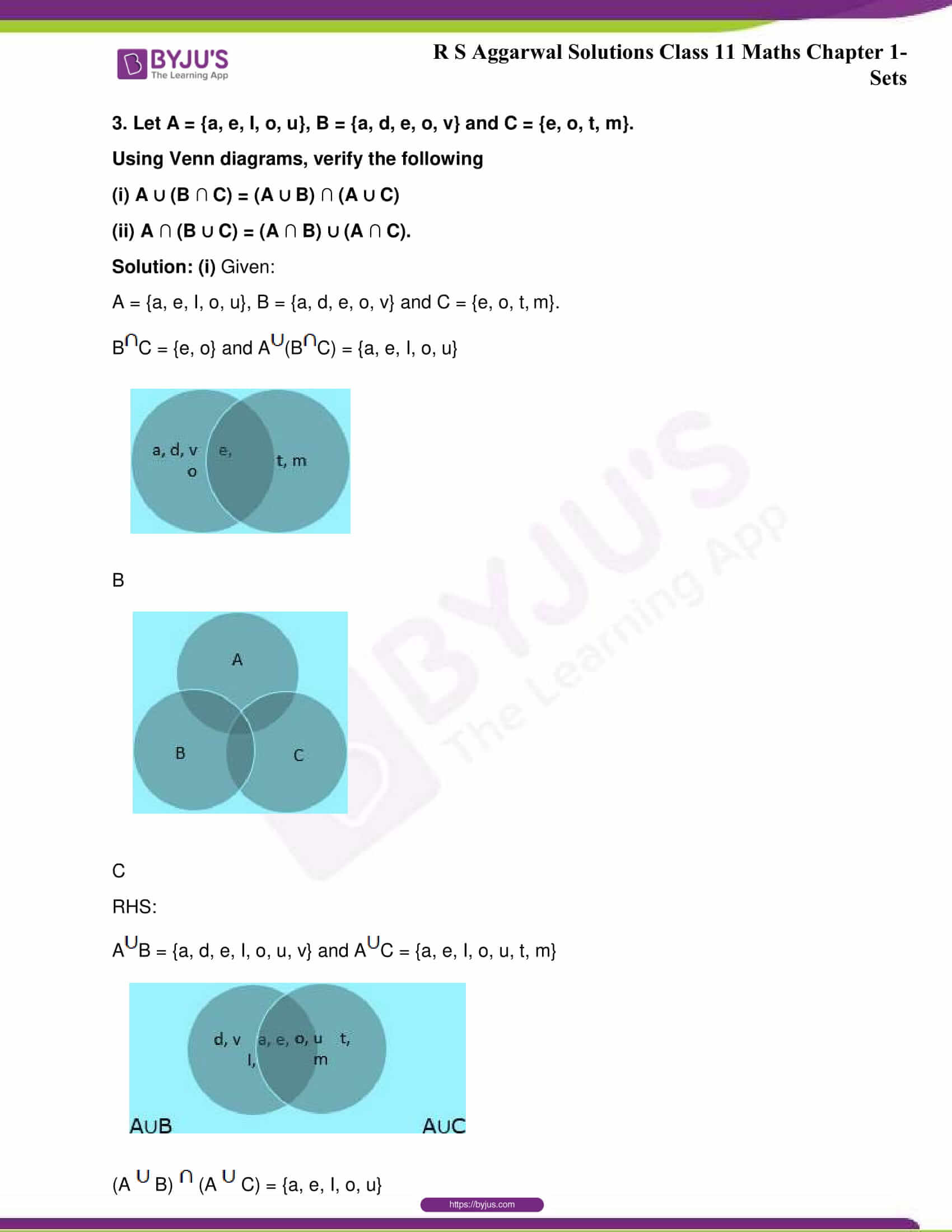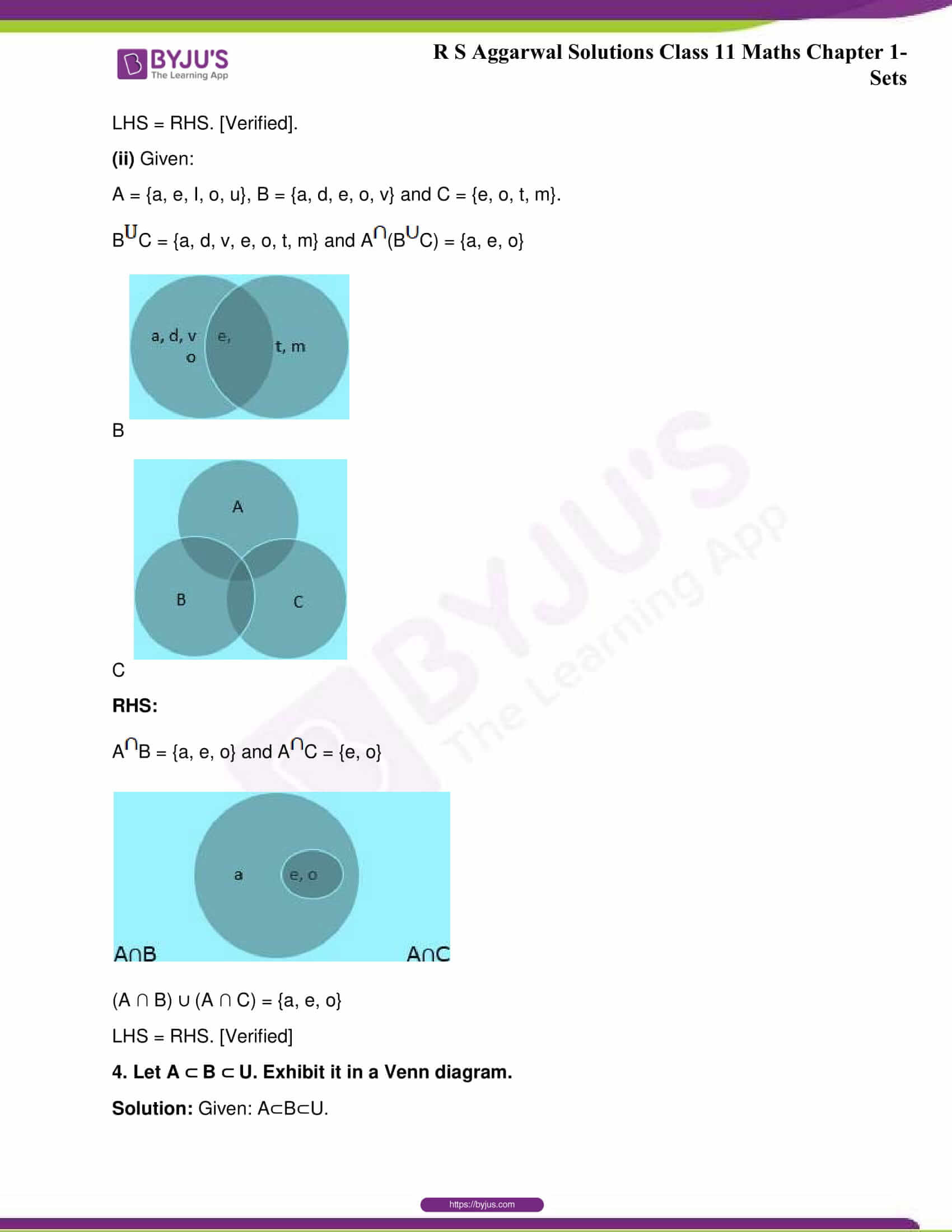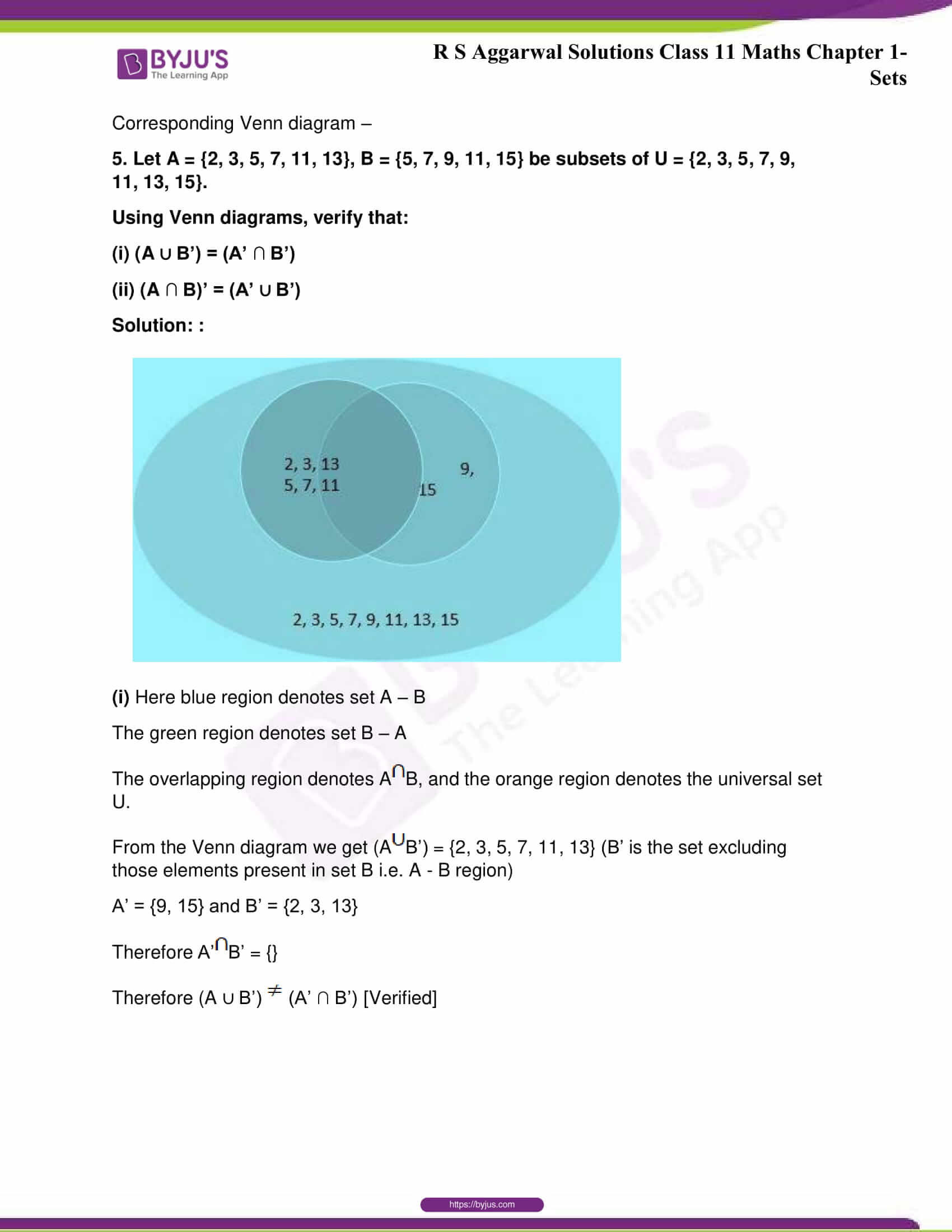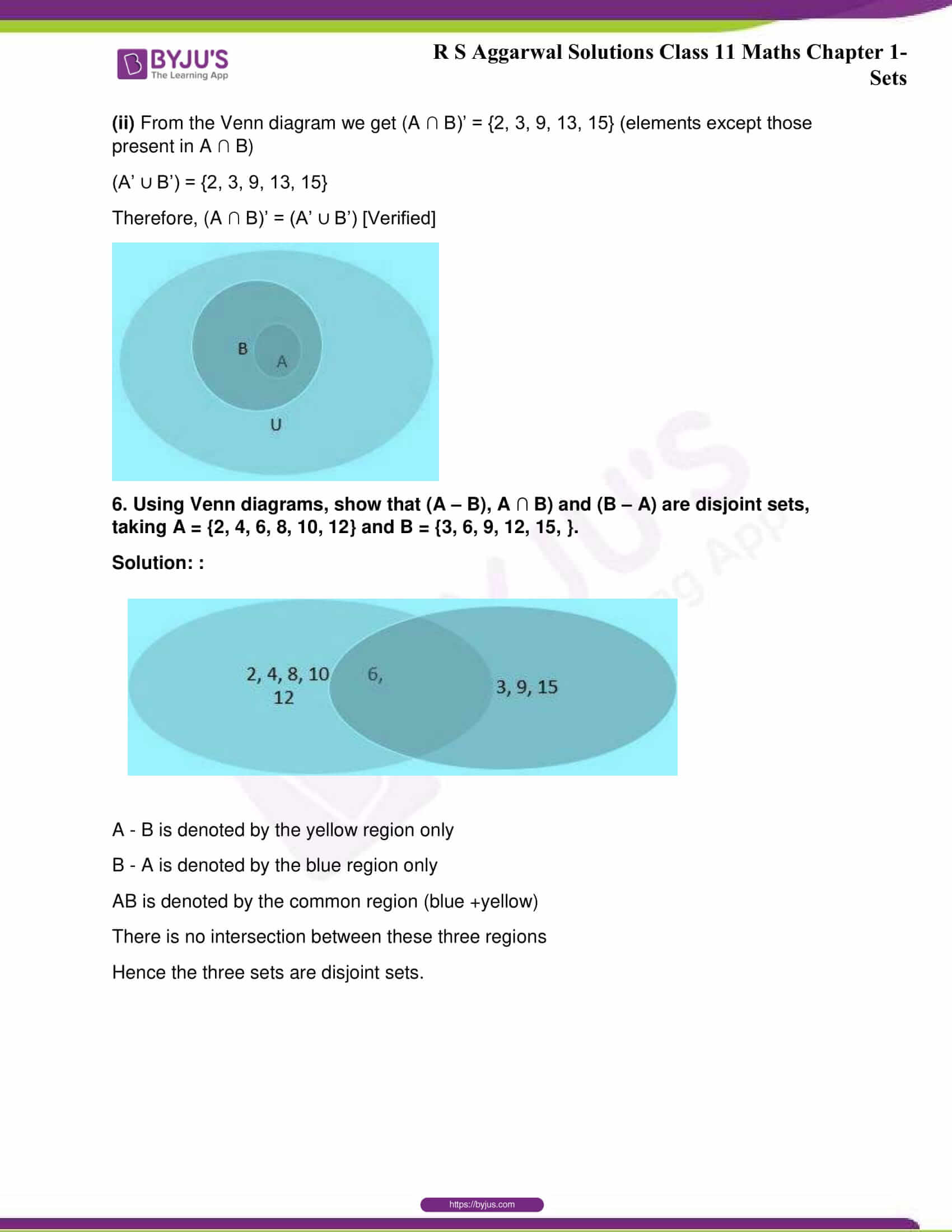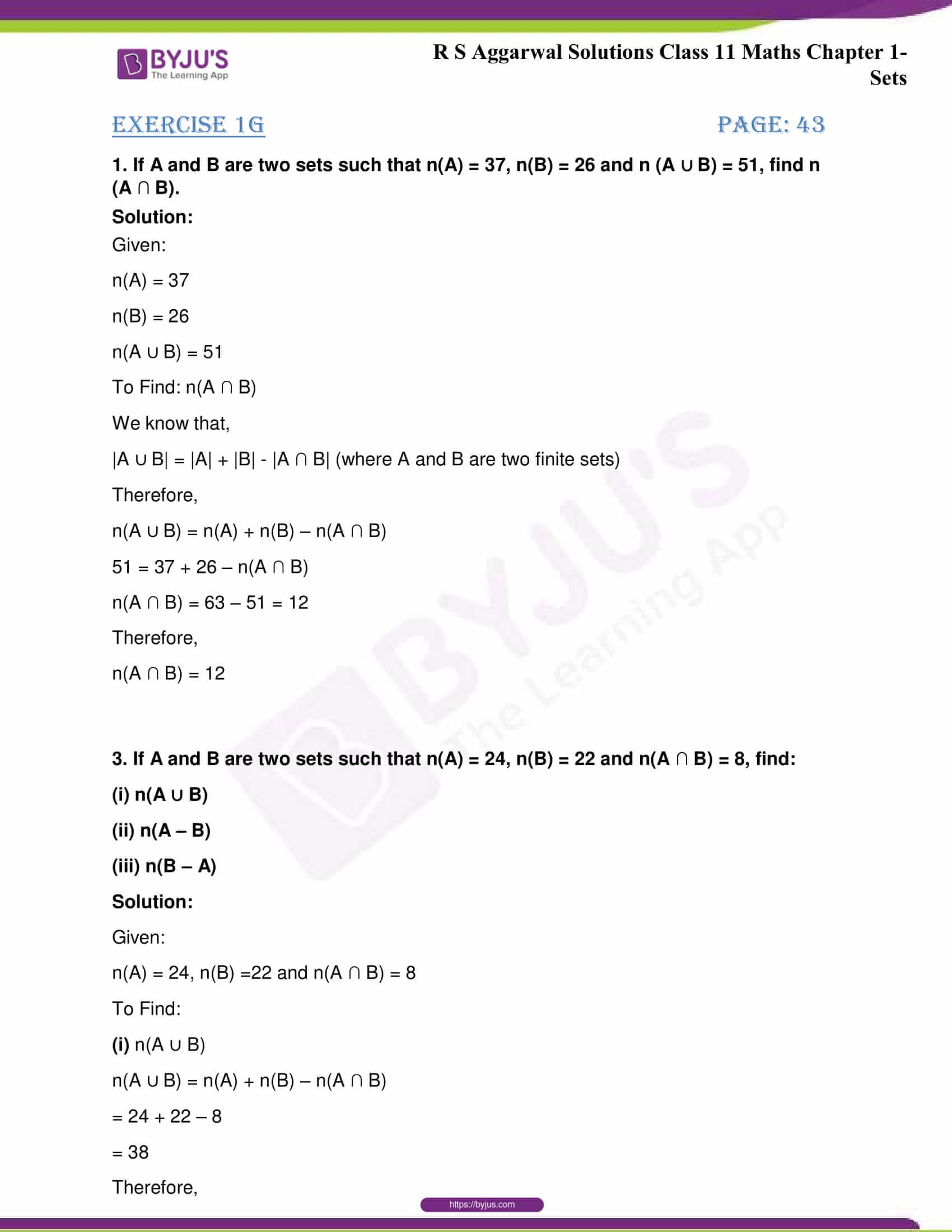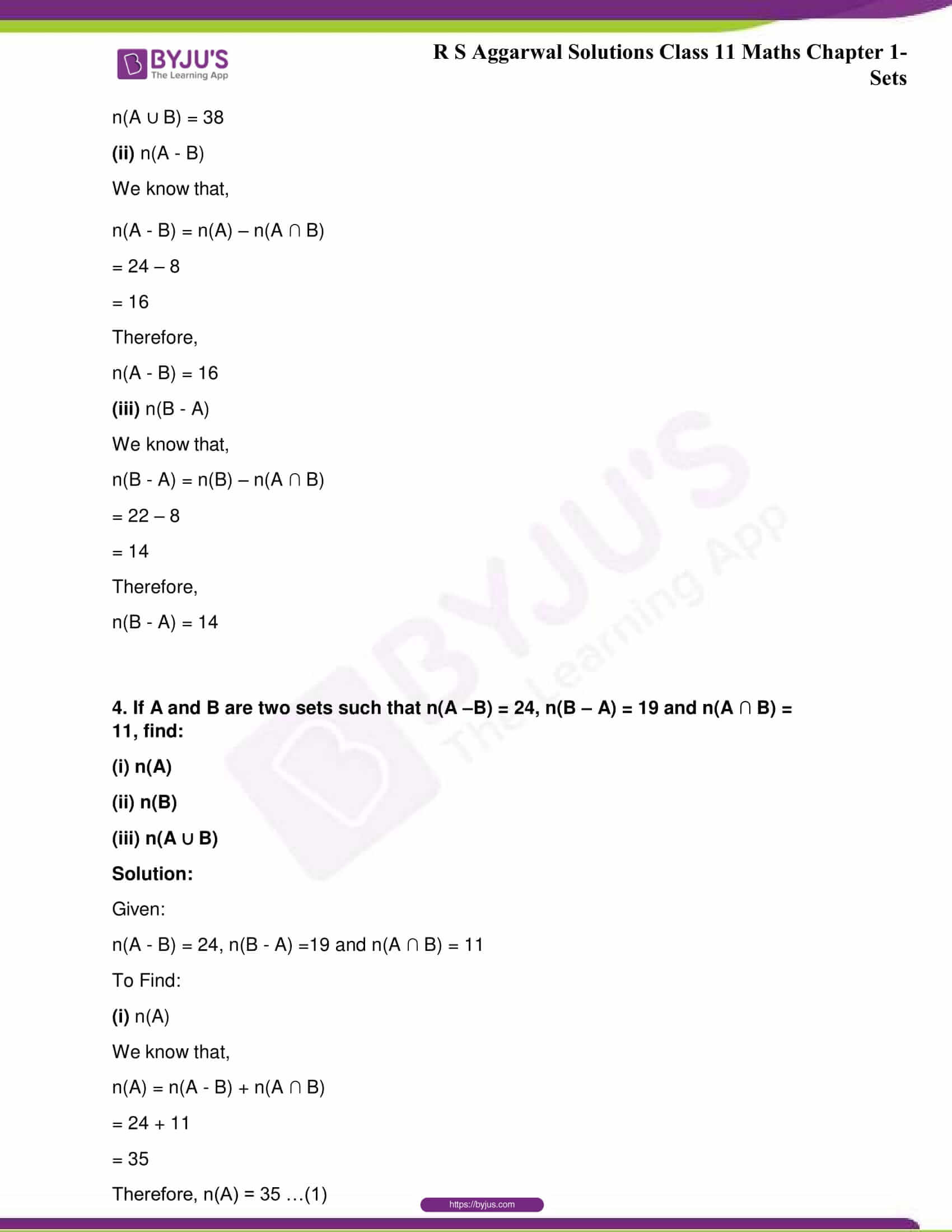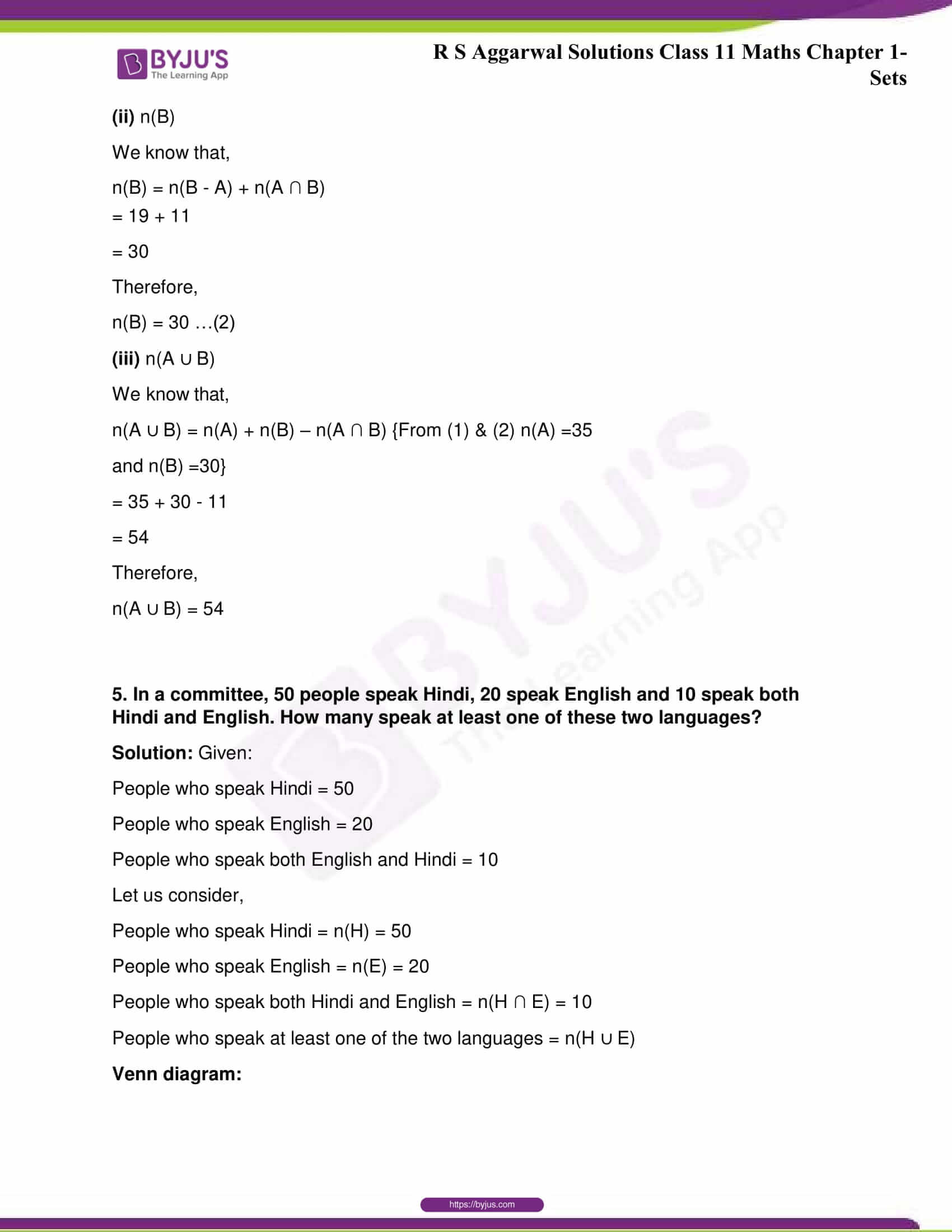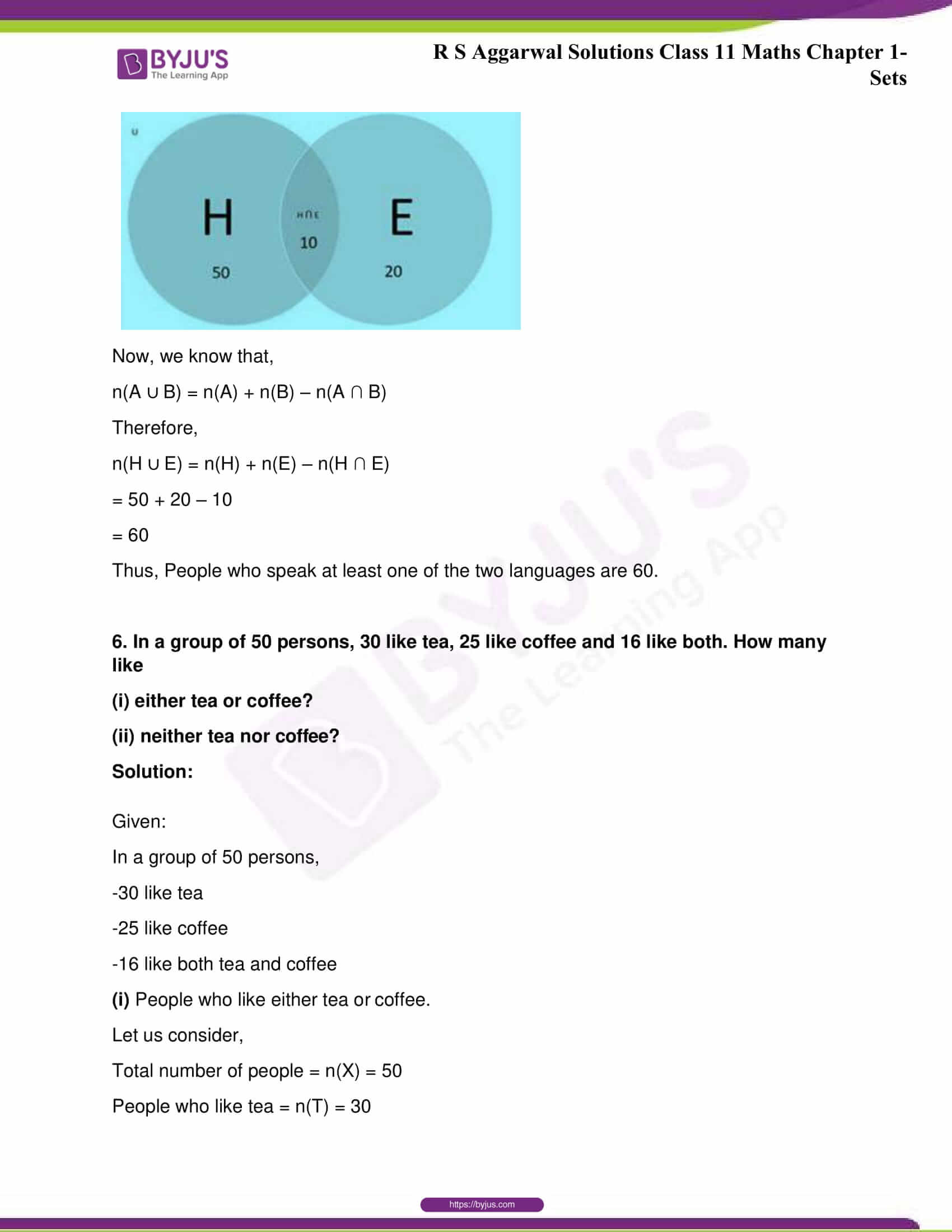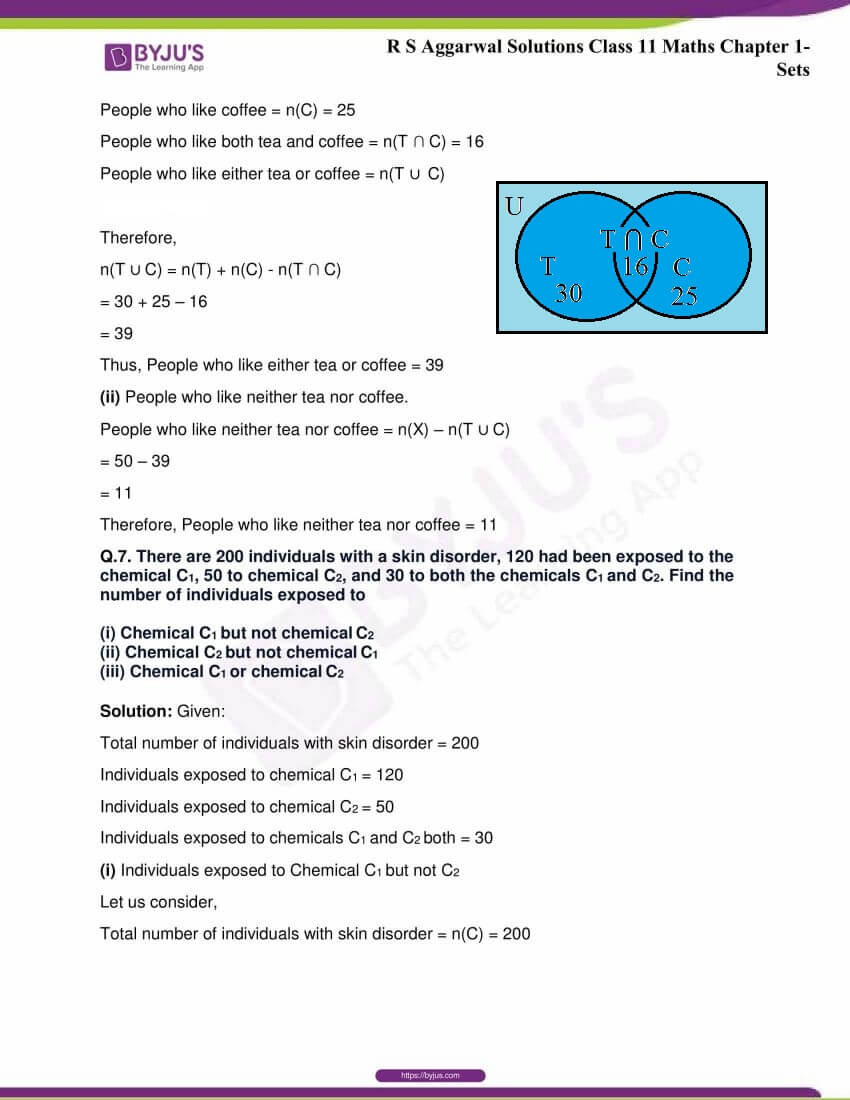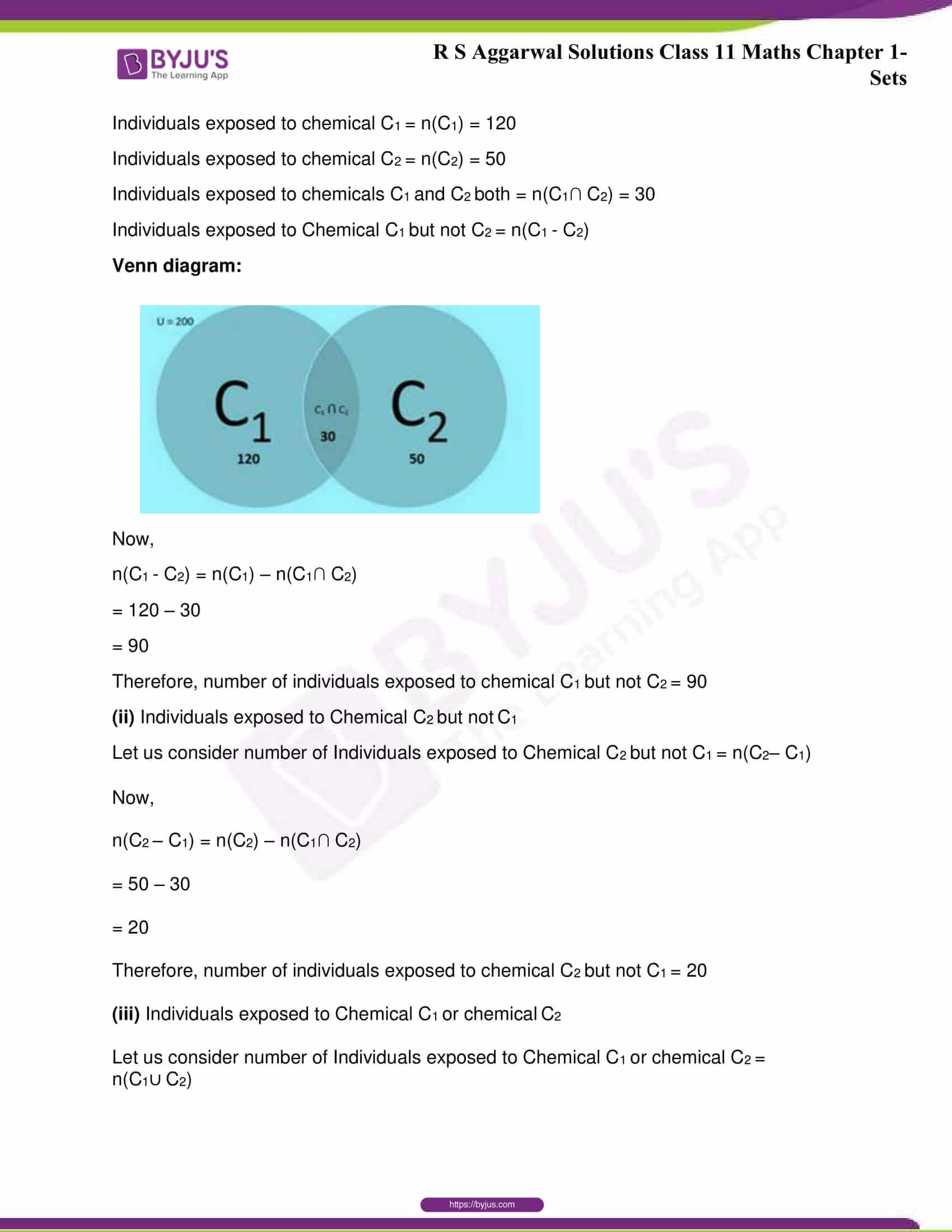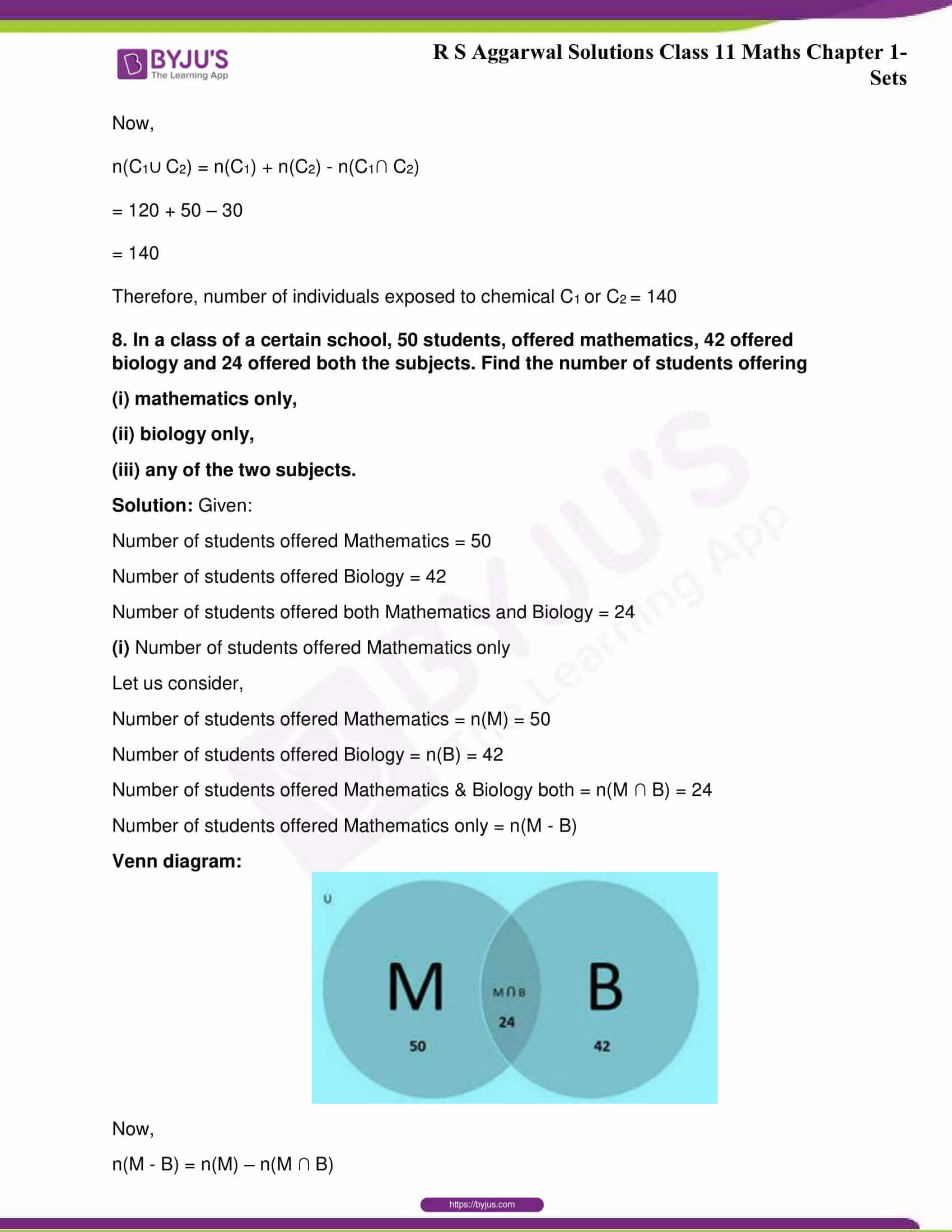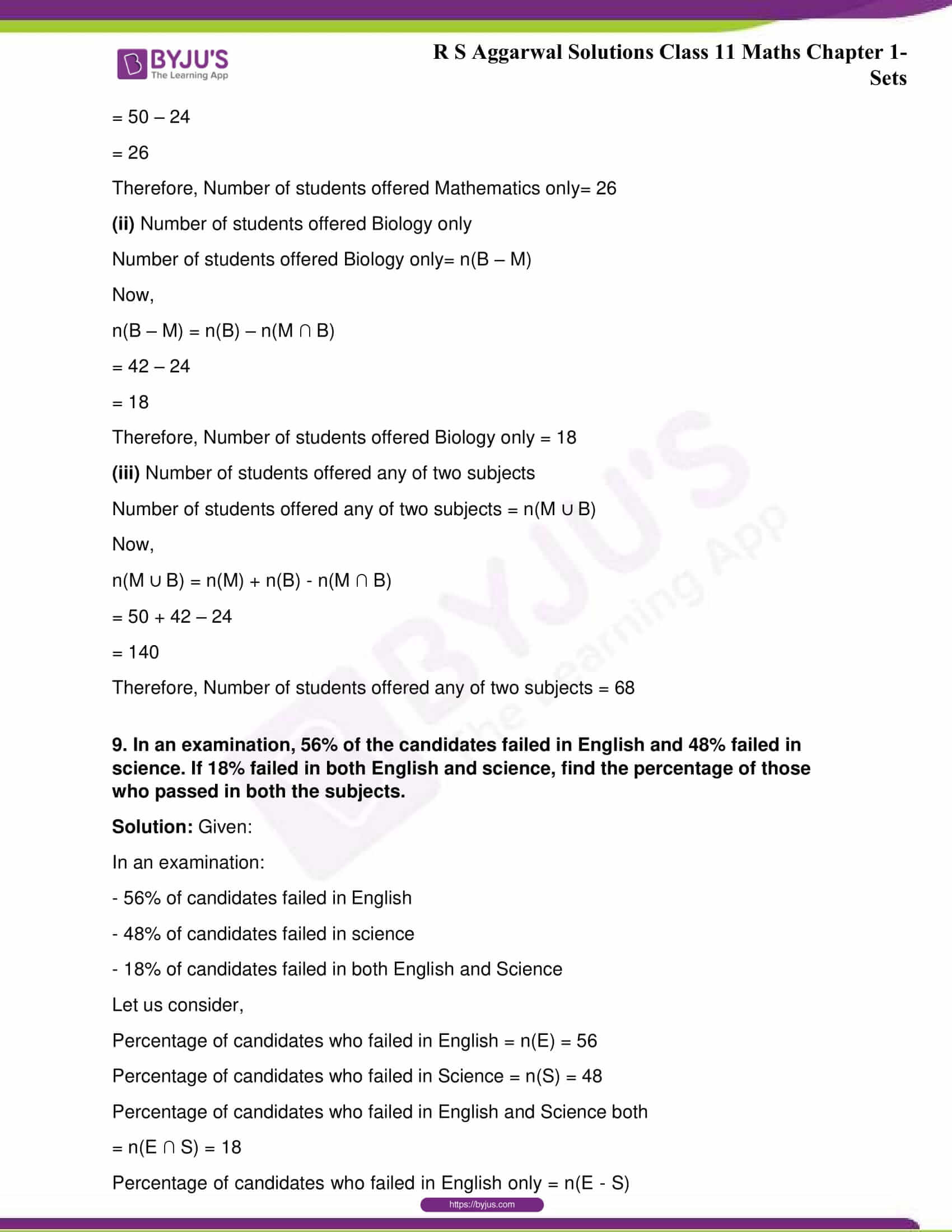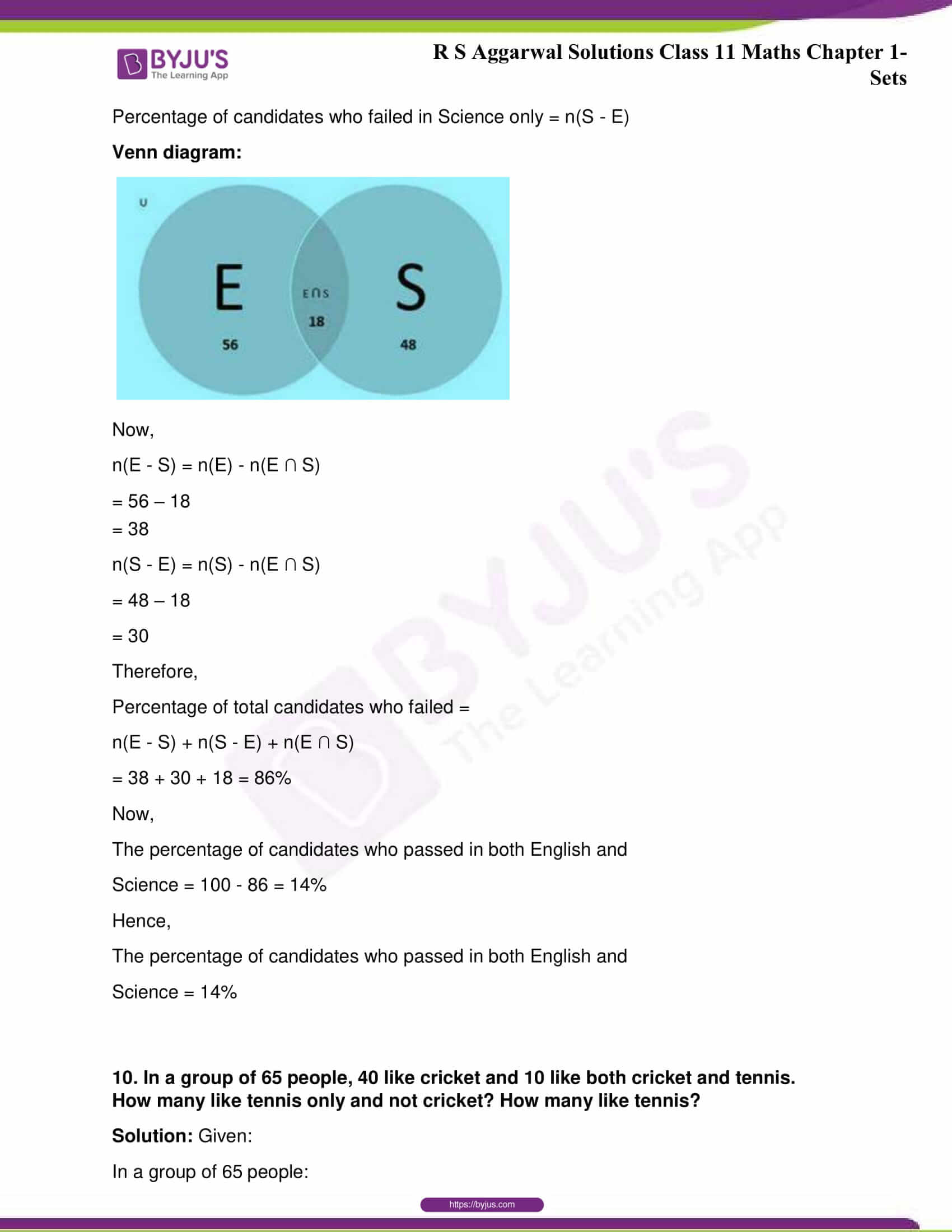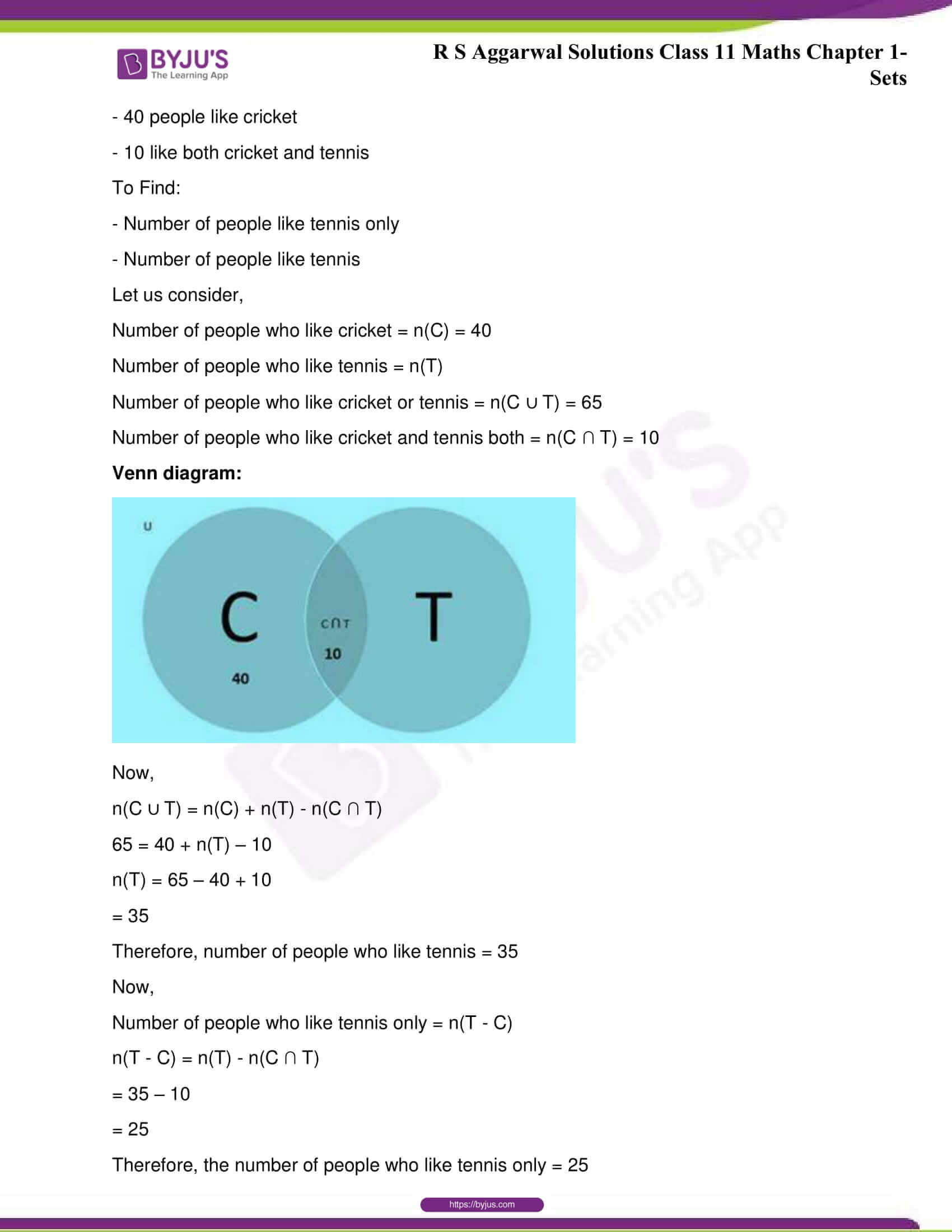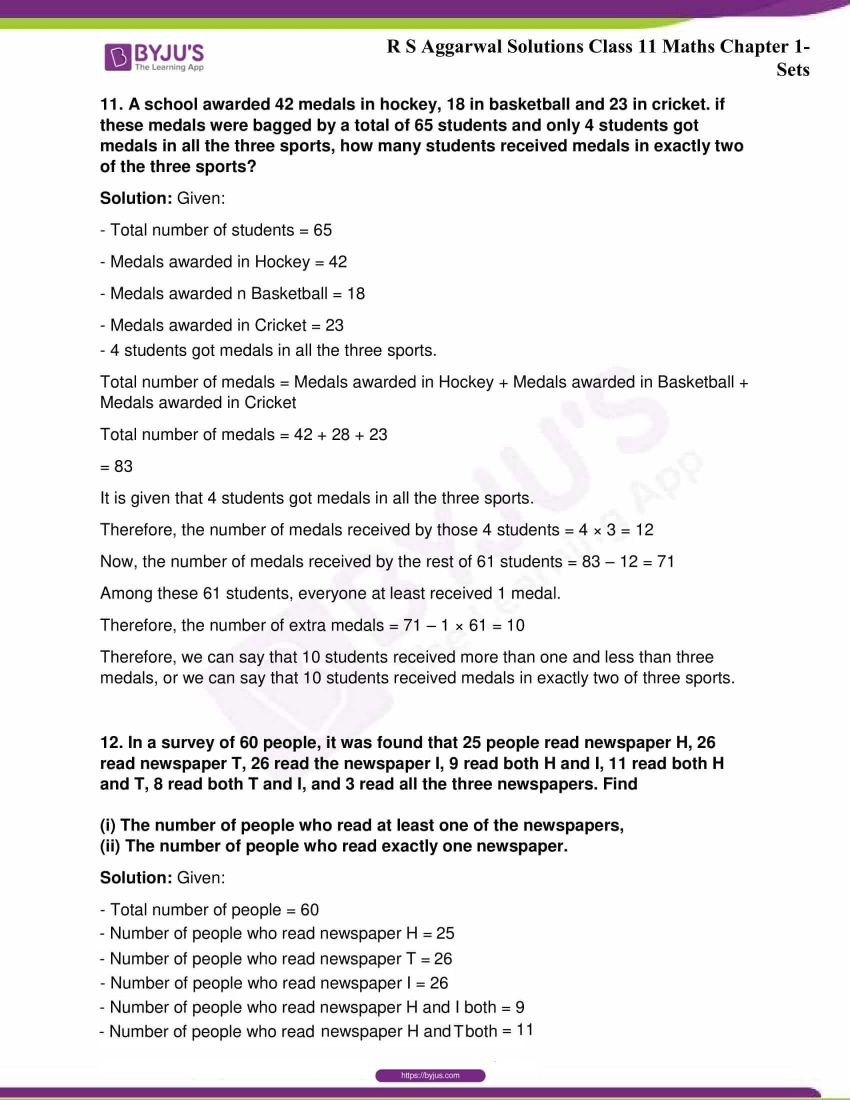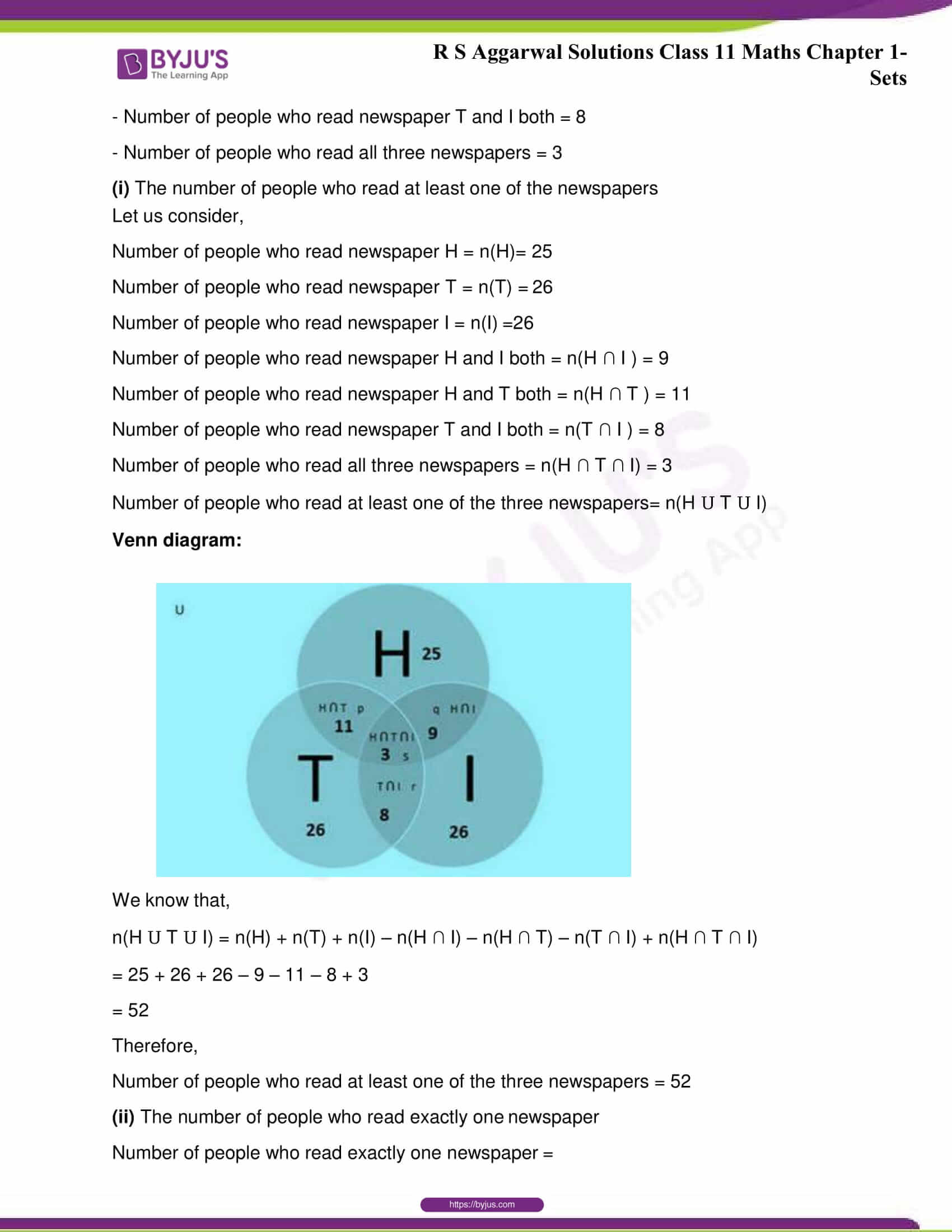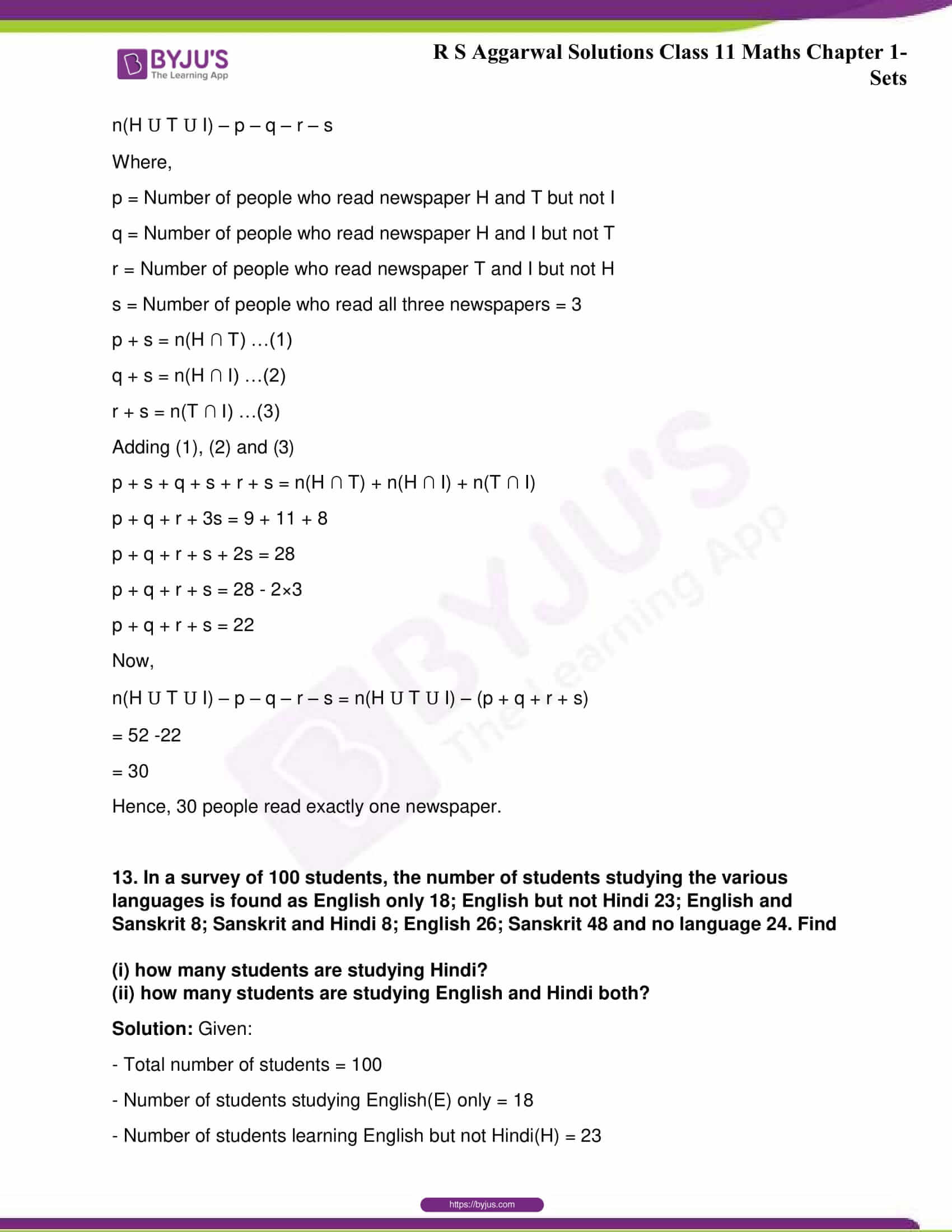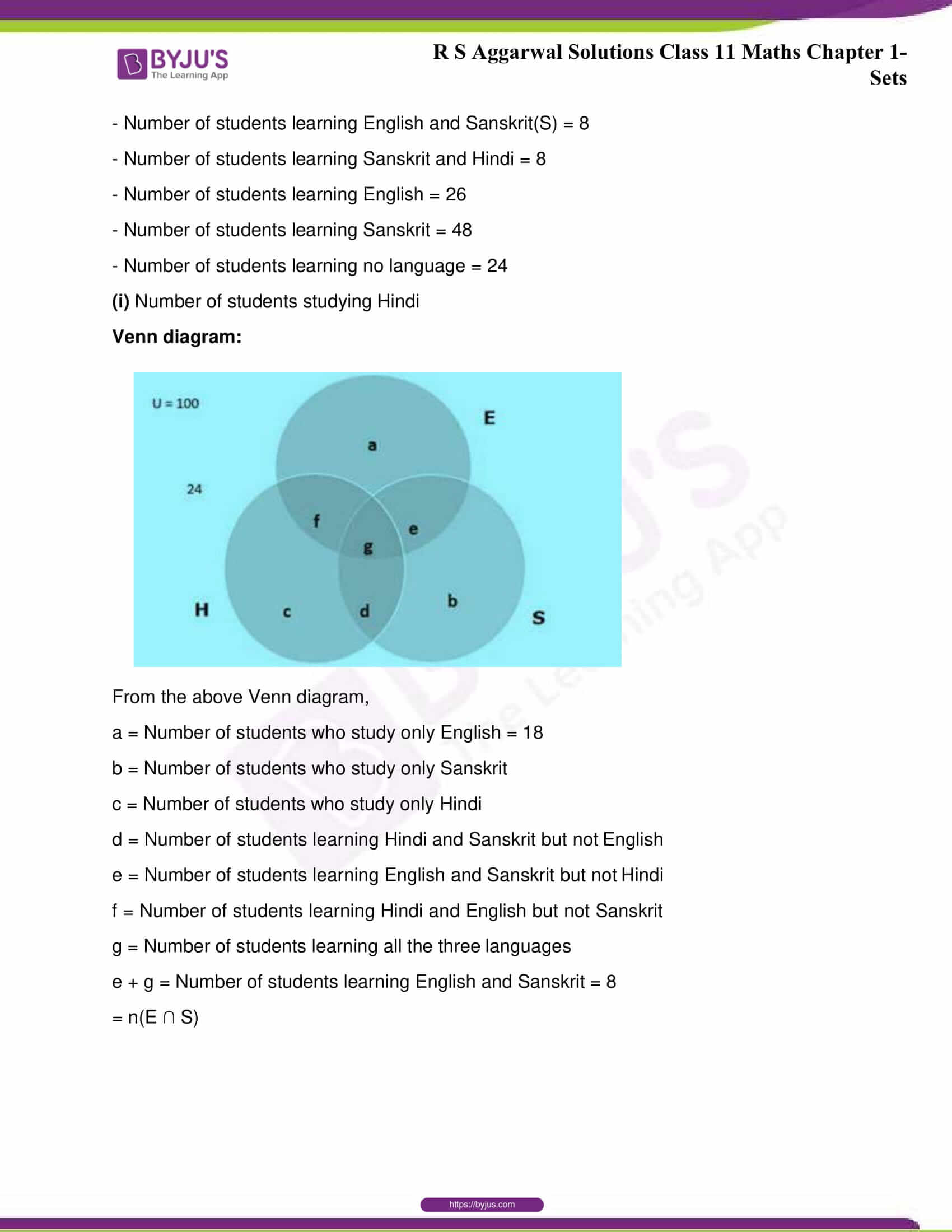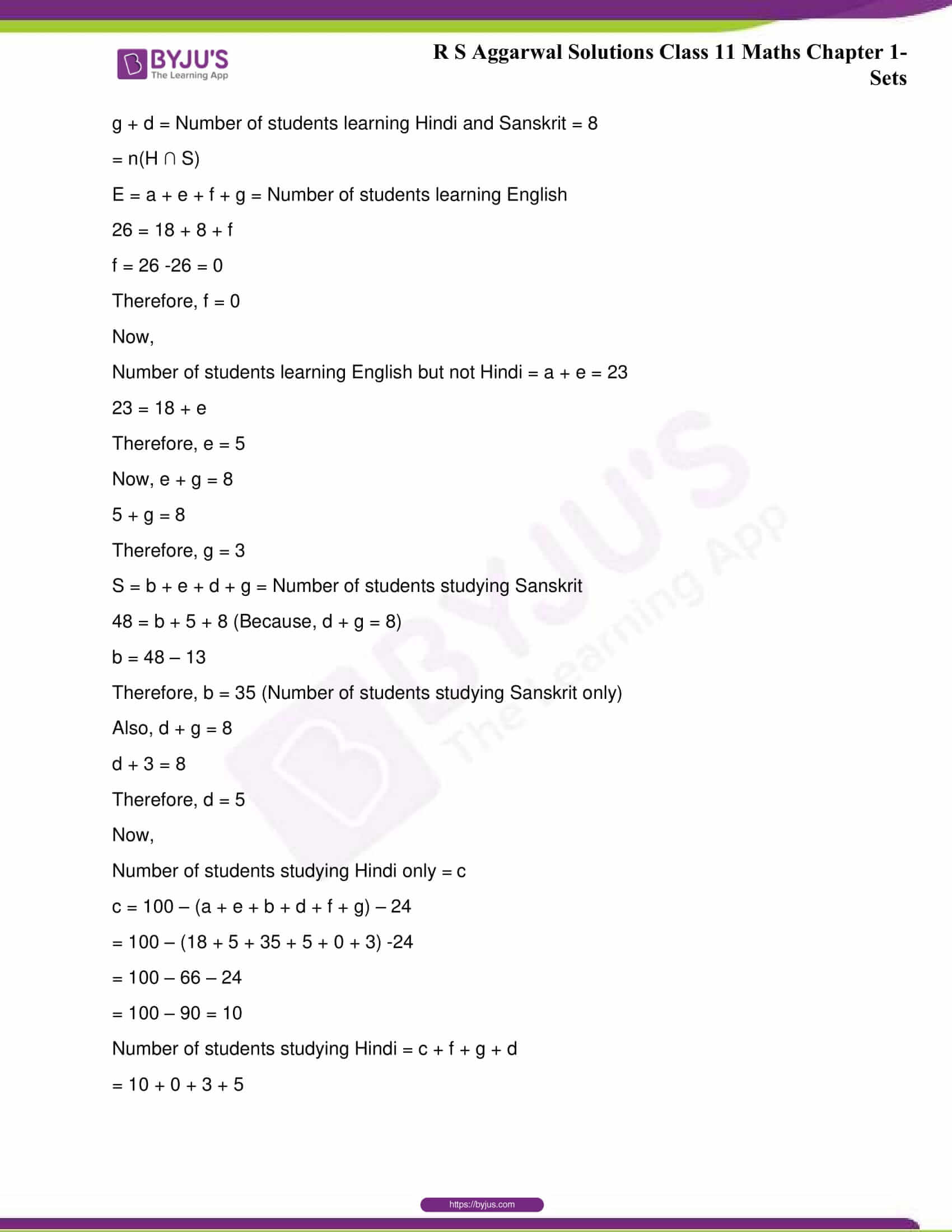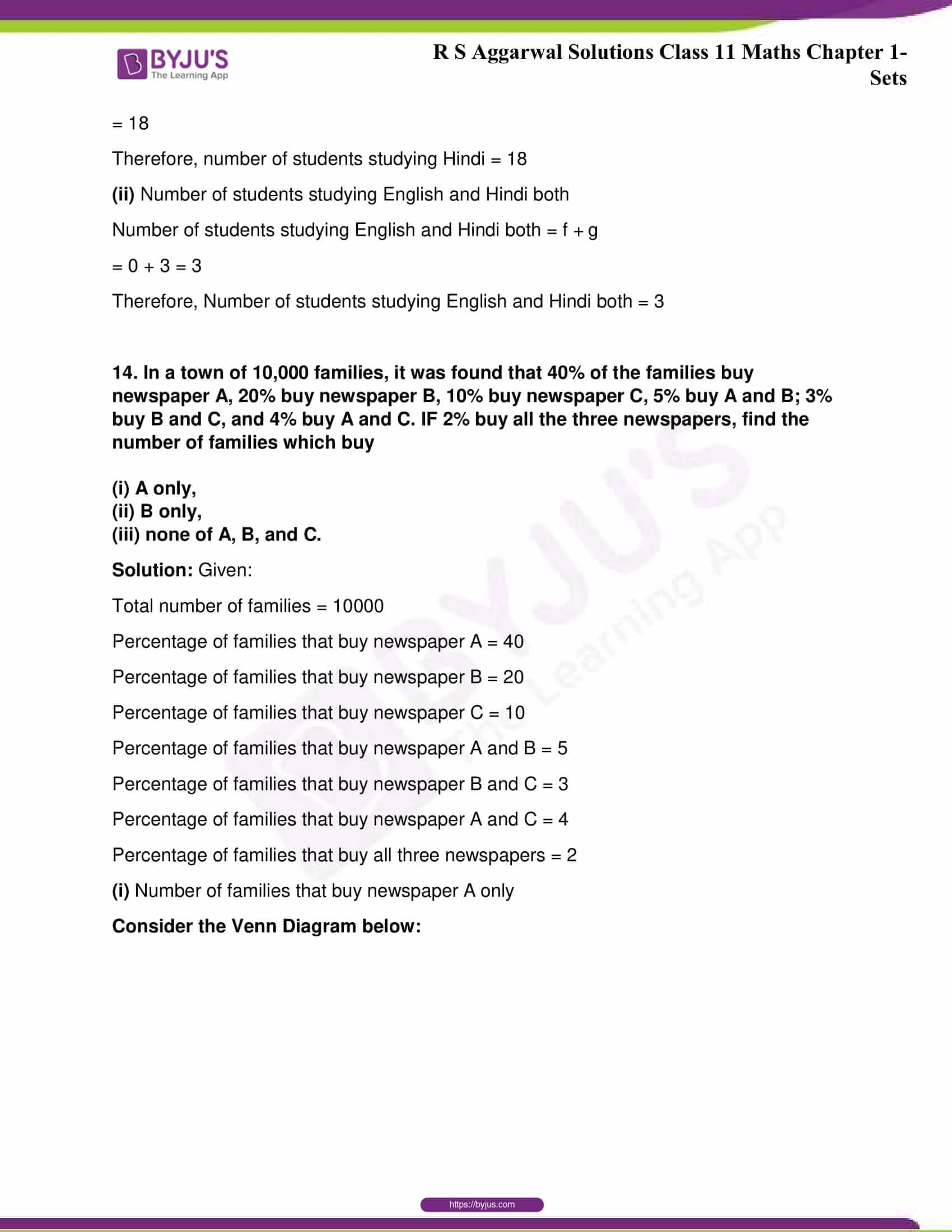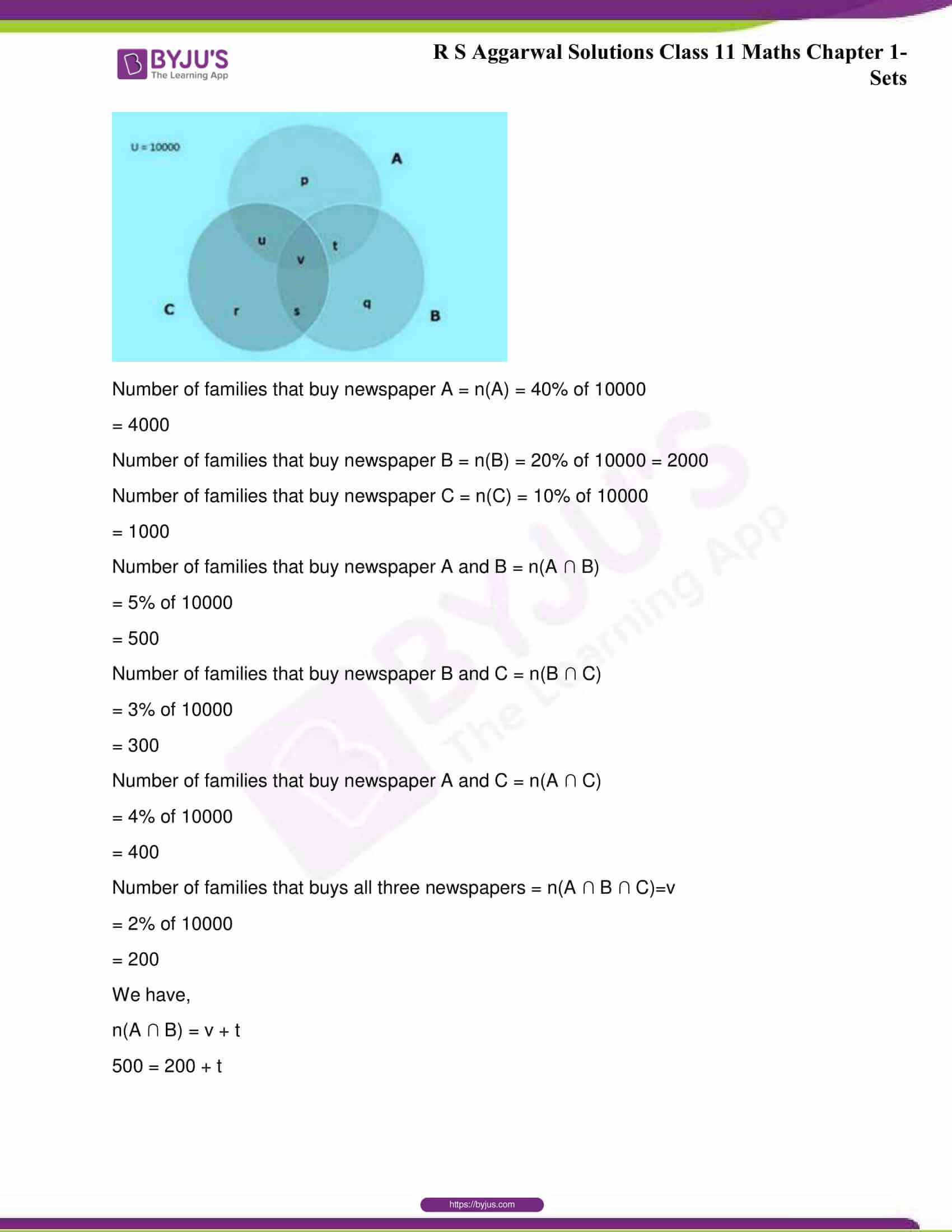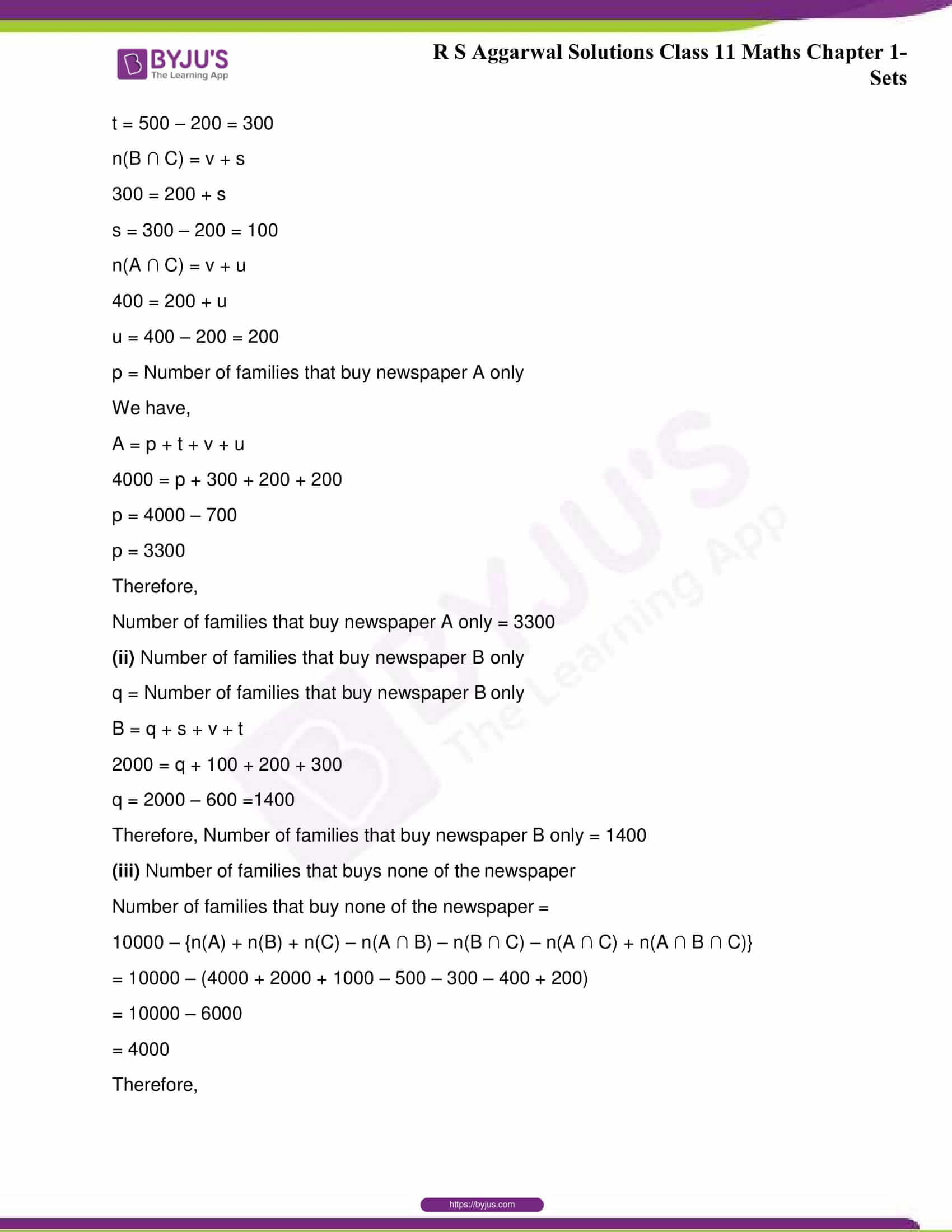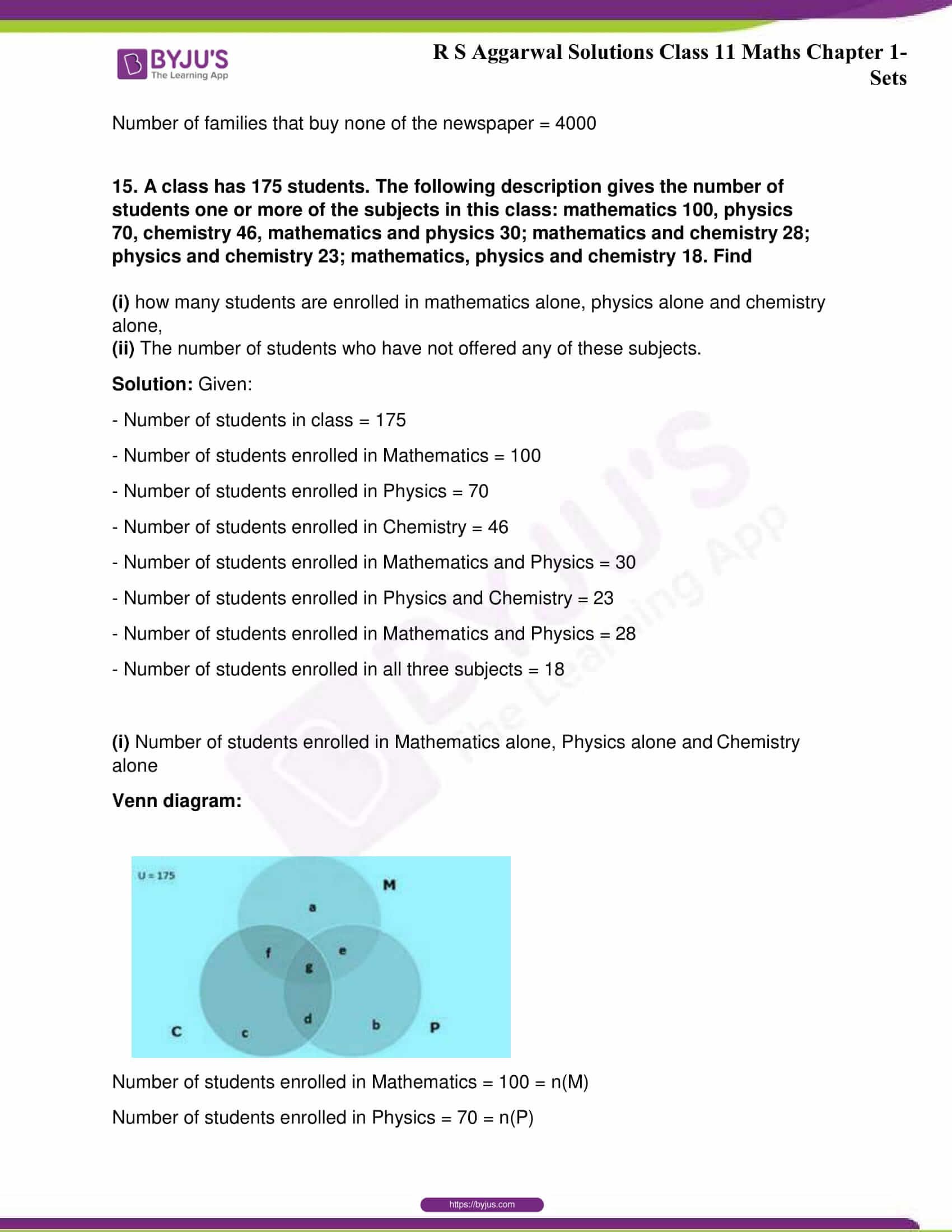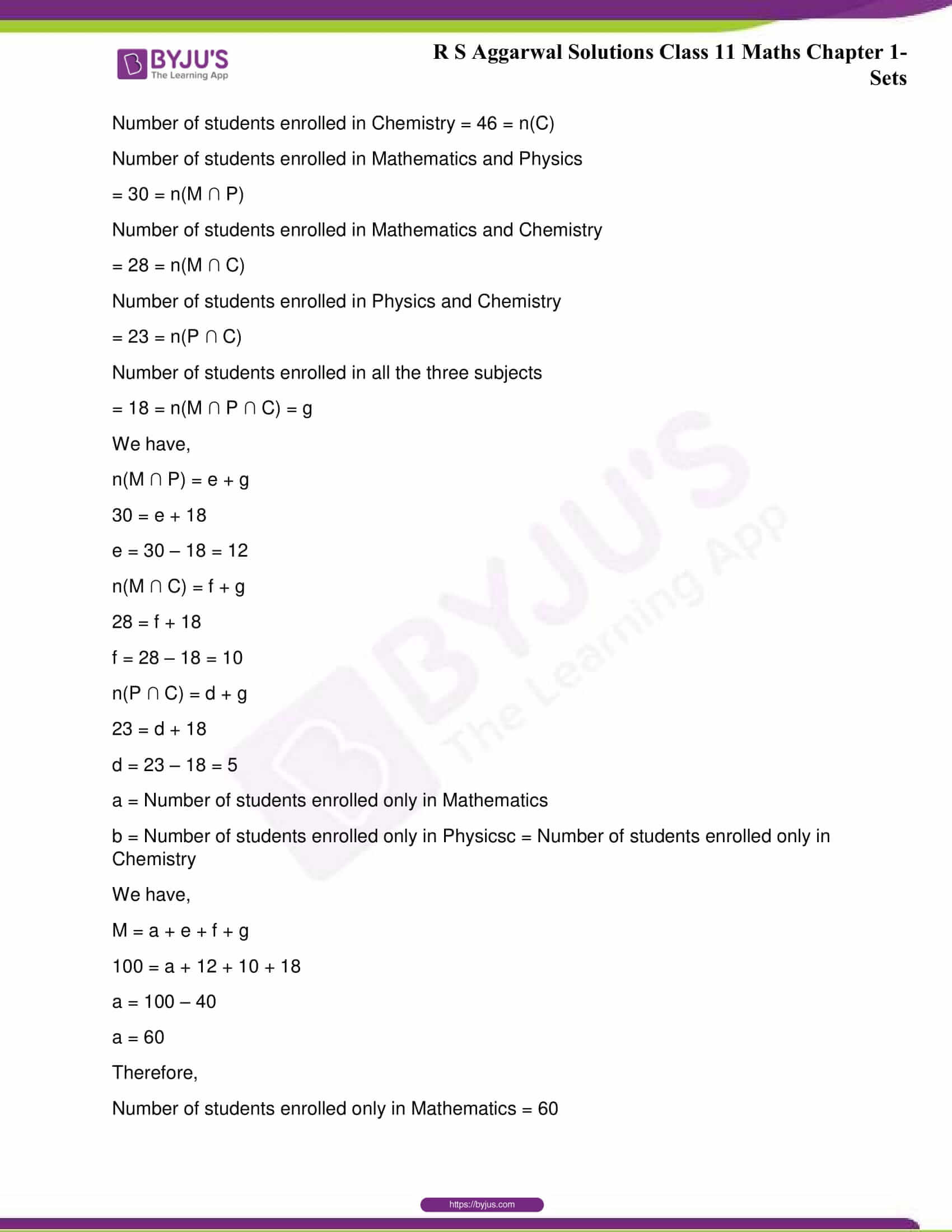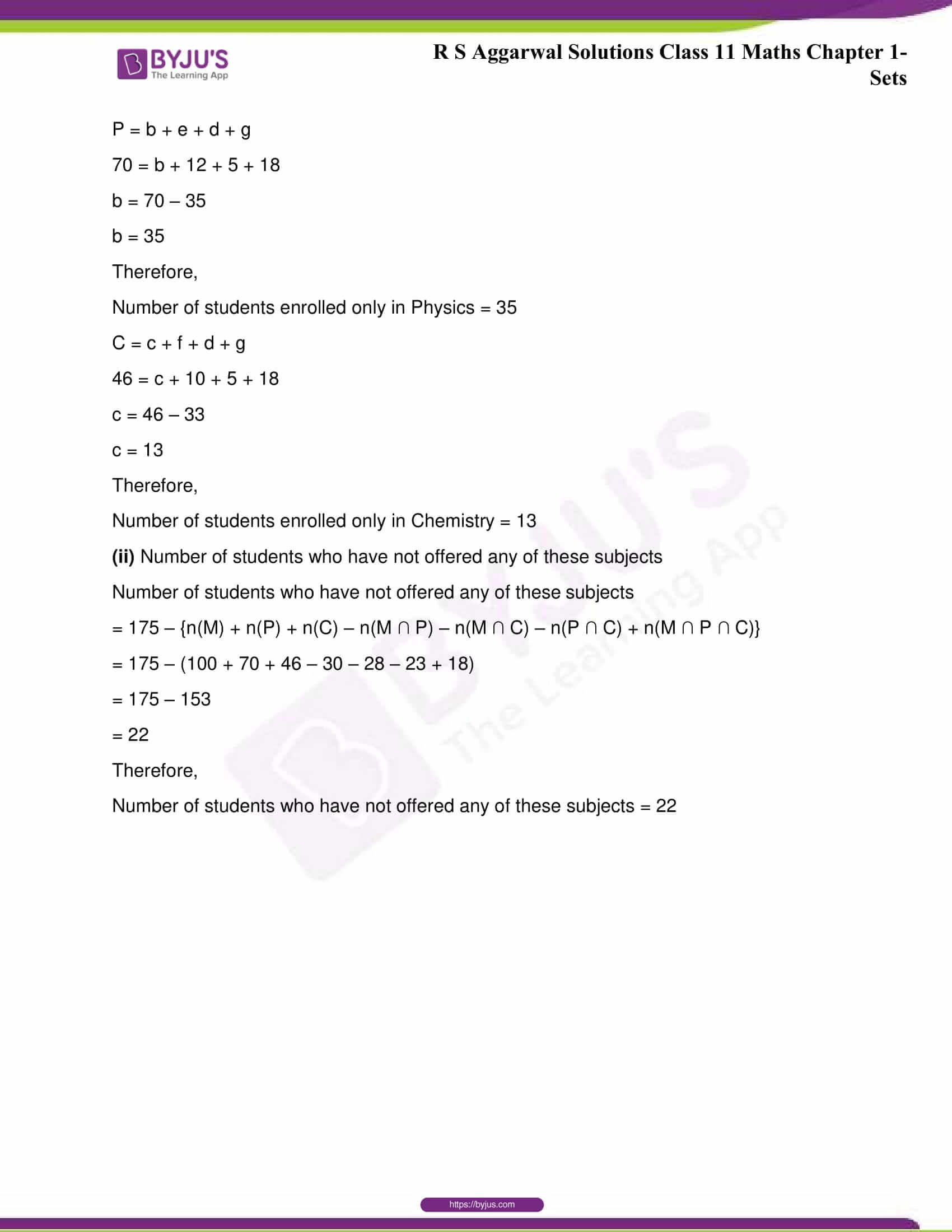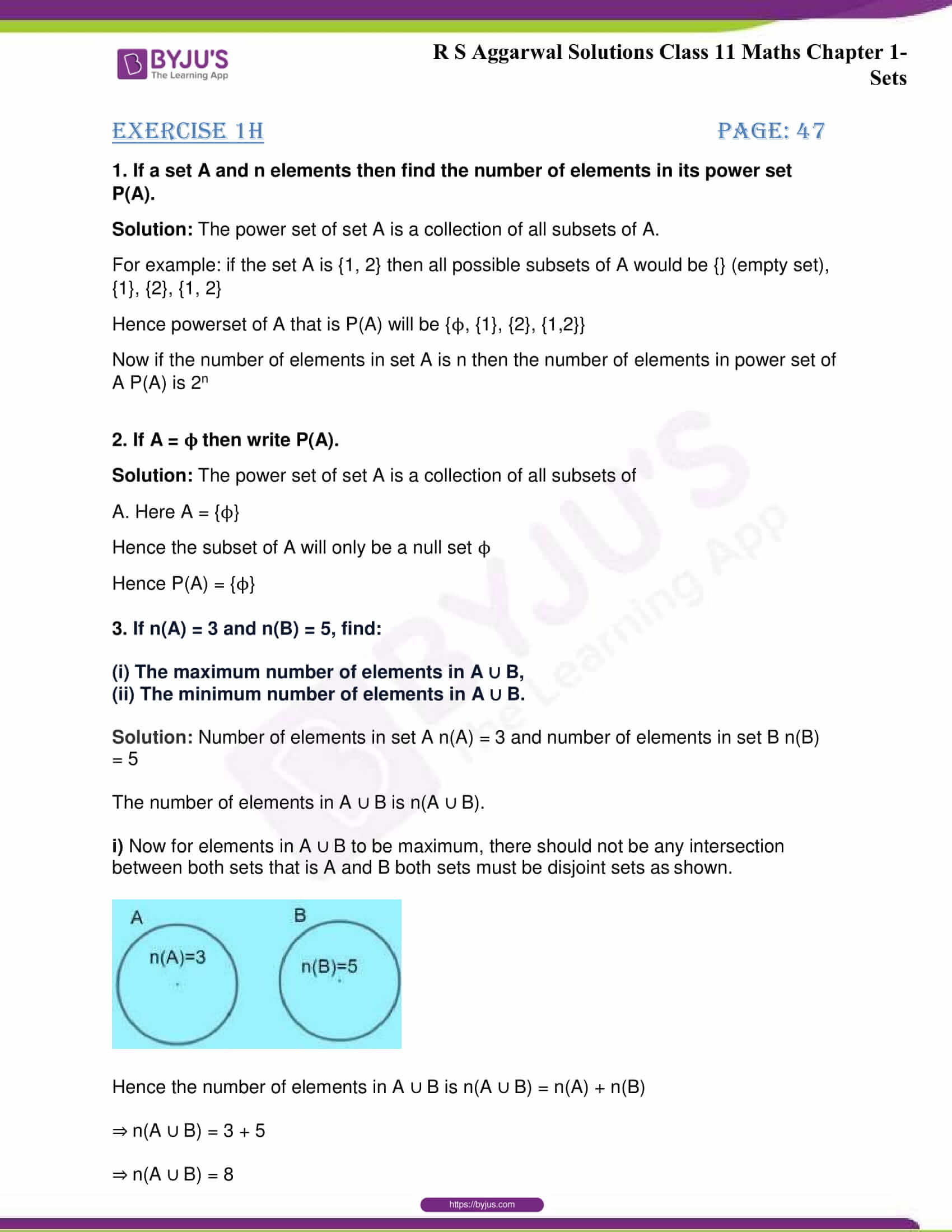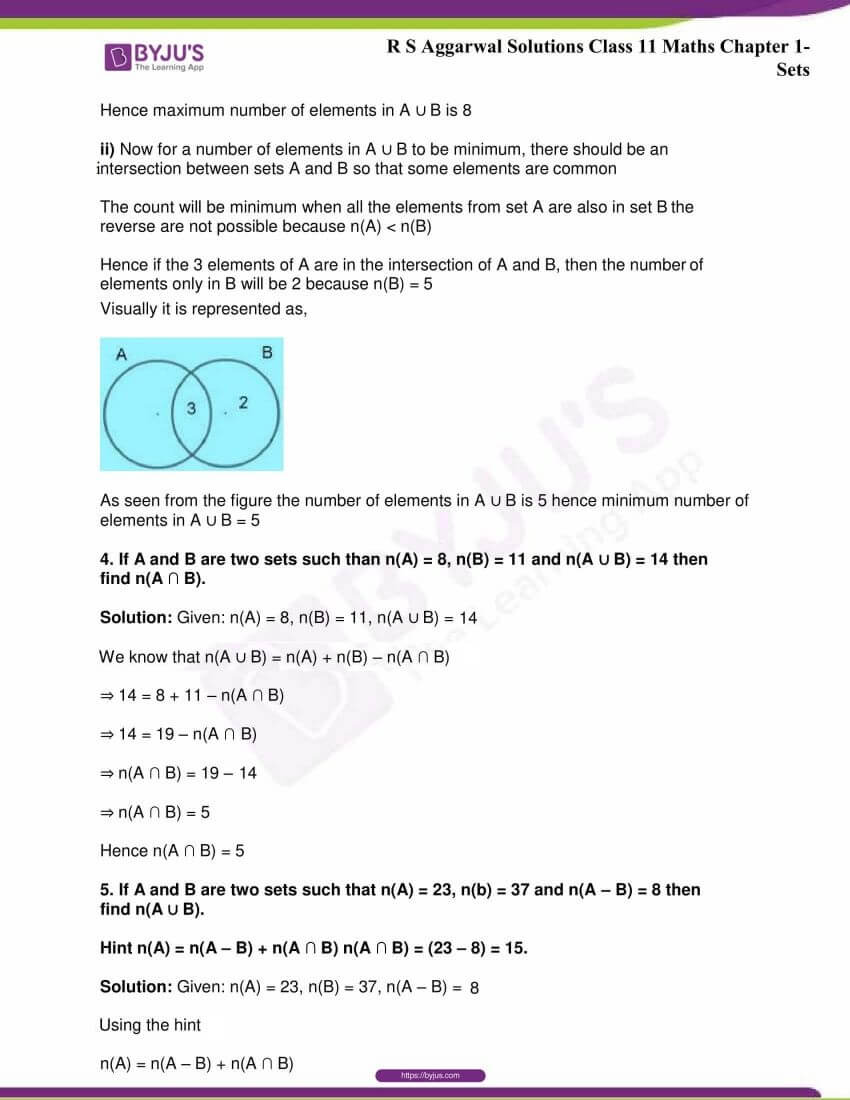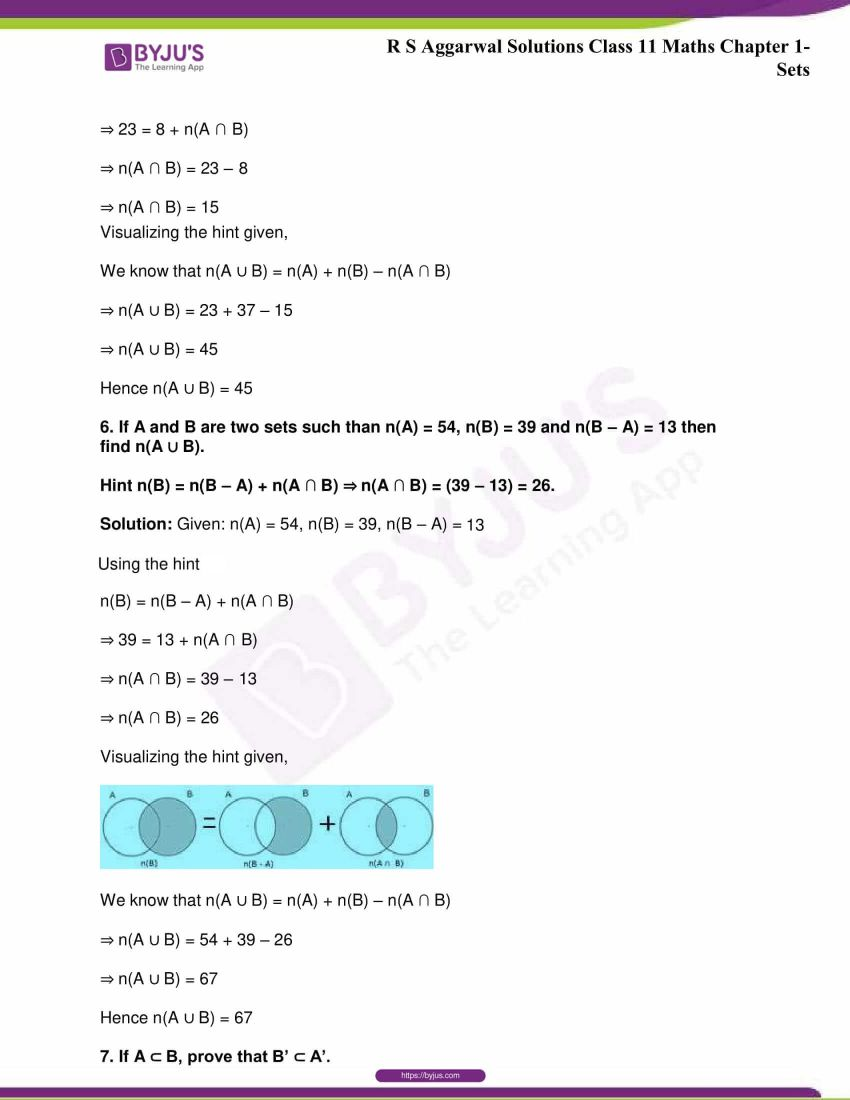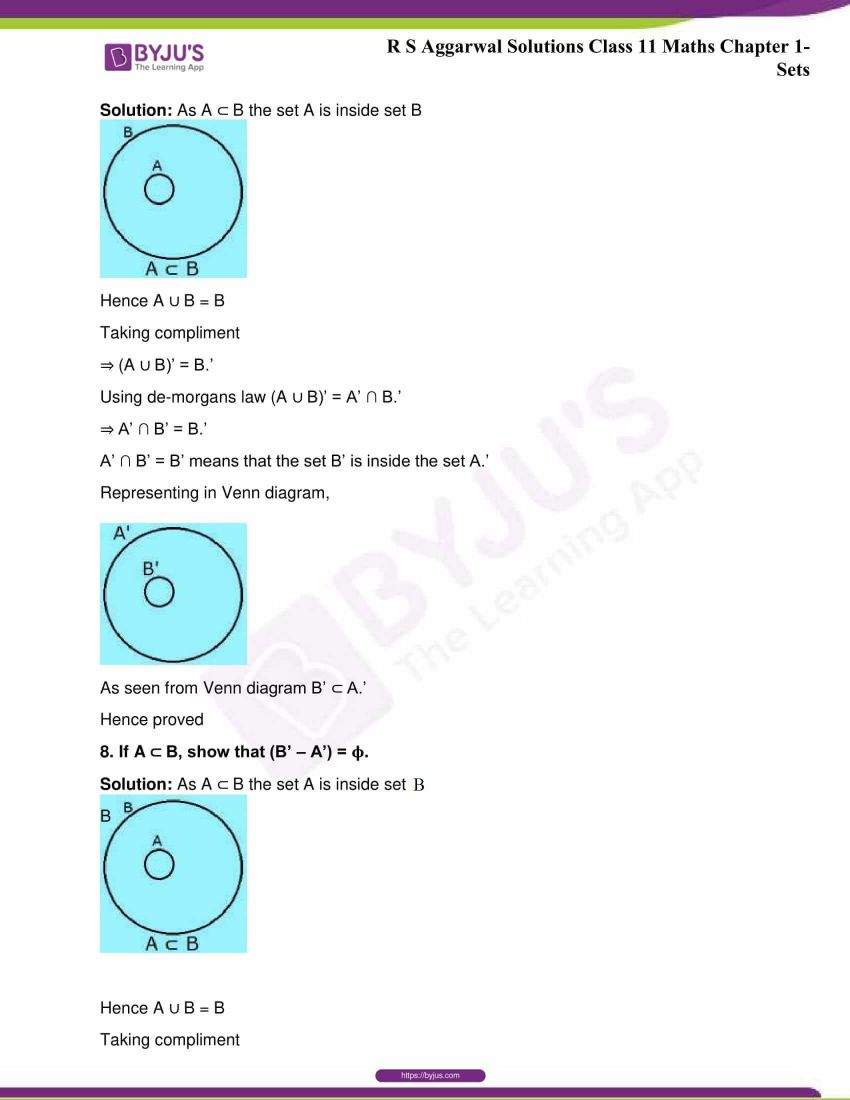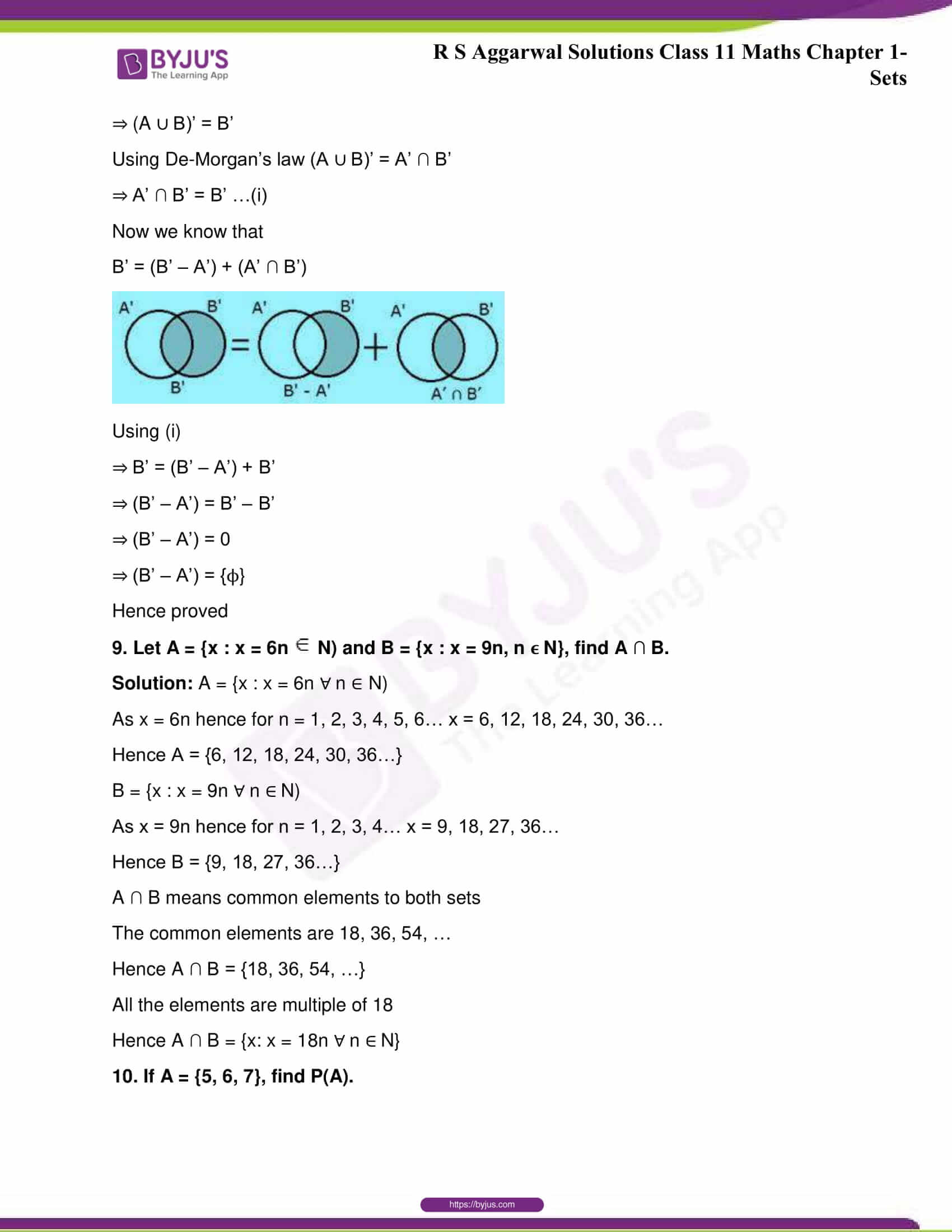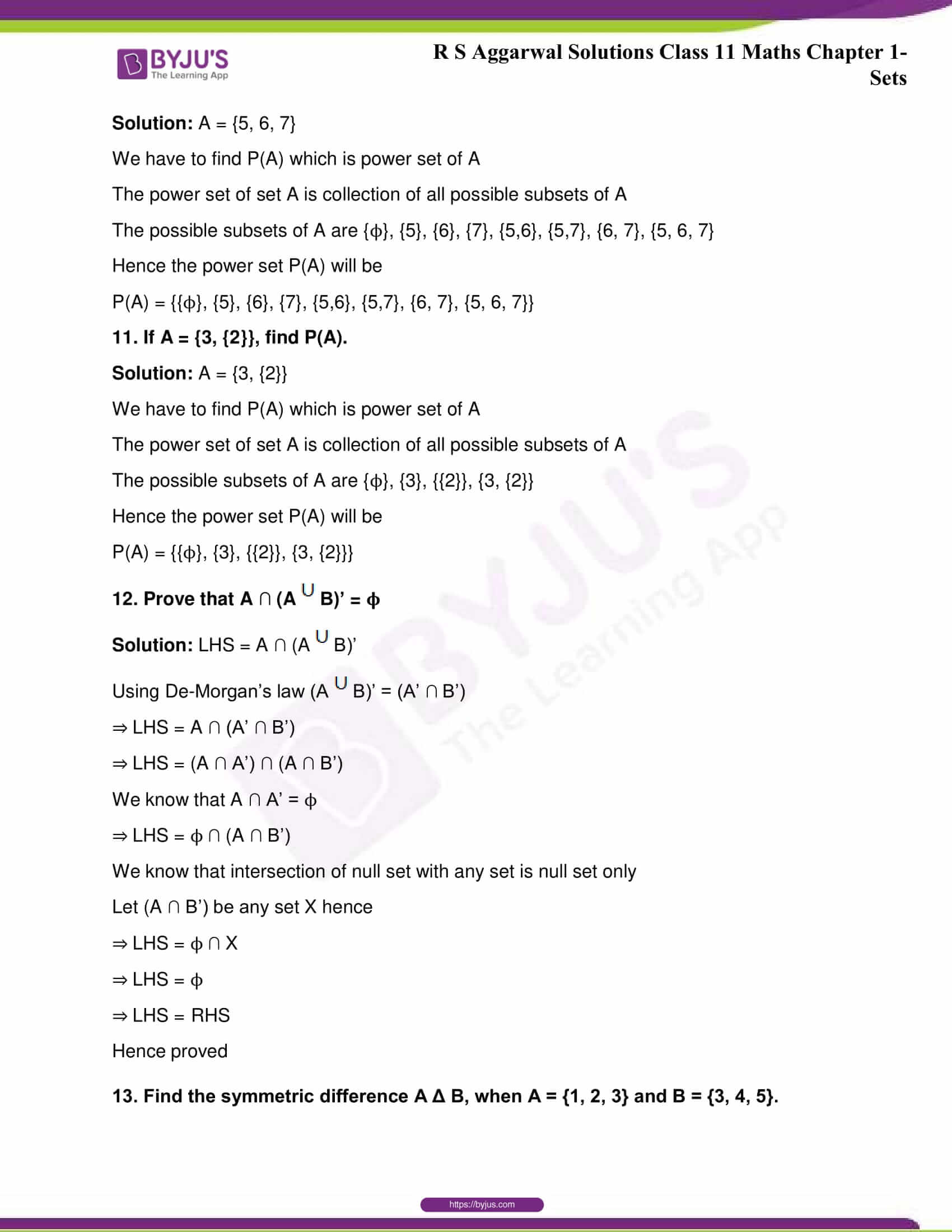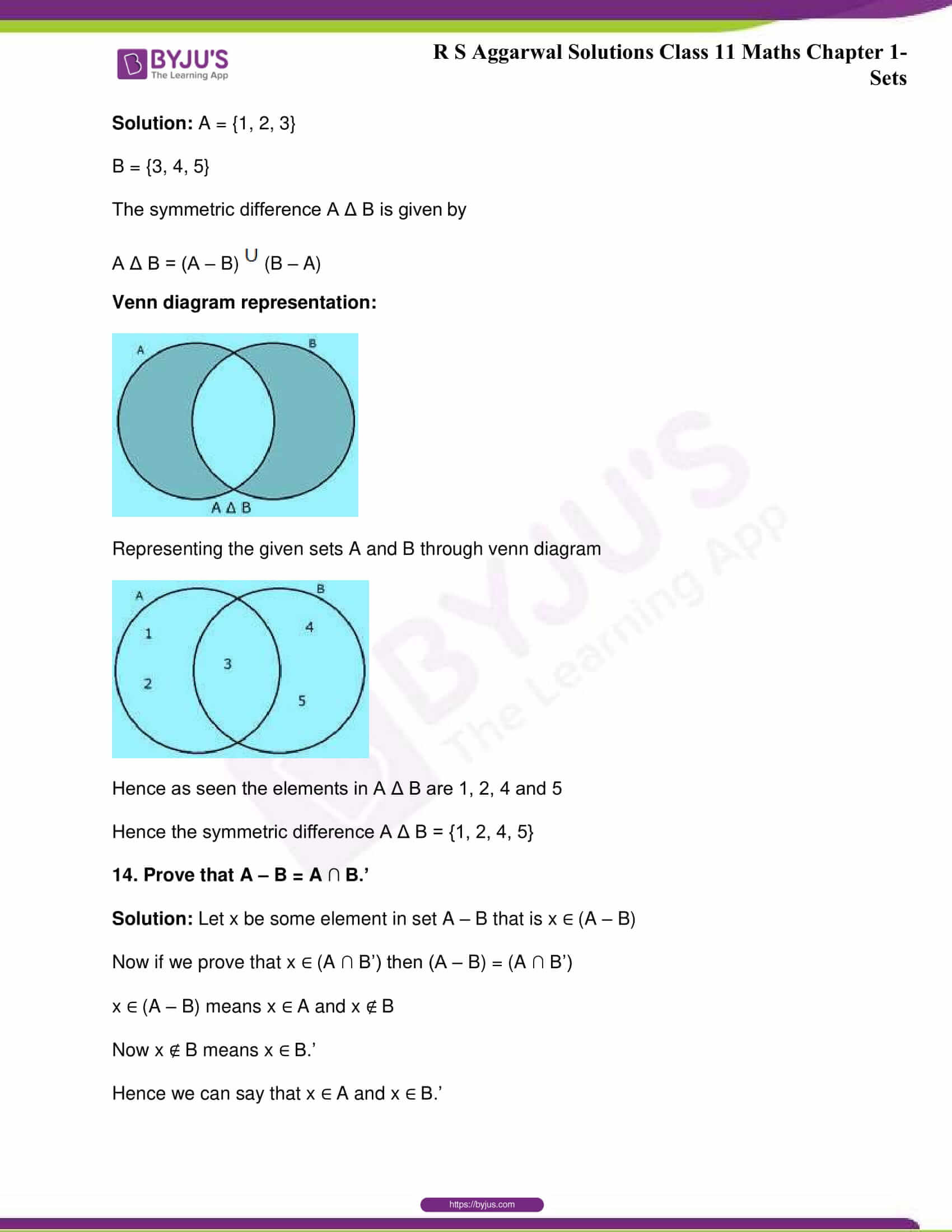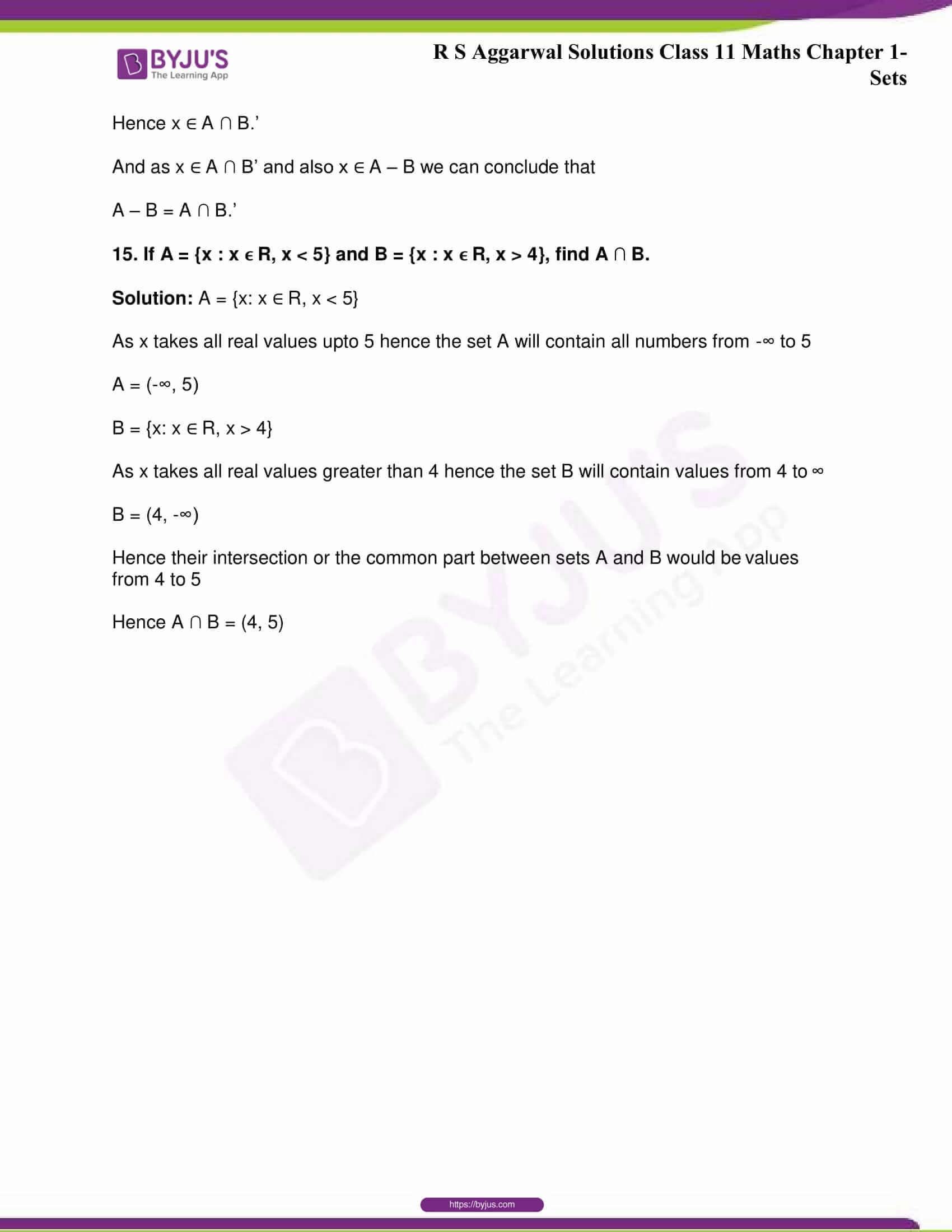## R S Aggarwal Solutions for Class 11 Maths Chapter 1- Sets

Chapter 1, Sets, has 8 exercises and the R S Aggarwal Solutions given here contains the answers to all the questions present in these exercises. Let us have a look at some of the topics that are being discussed in this chapter.

1.1 Introduction

1.2 How to describe a set?

1.3 Some terms related to sets

1.4 Finite and Infinite sets

1.5 Subsets

1.6 Operations on sets

1.7 Laws of operations on sets

1.8 Venn Diagrams

## R S Aggarwal Solutions for Class 11 Maths Chapter 1- Sets

A well-defined collection of objects is called a set. The objects in a set are called its members or elements or points. Chapter 1 of class 11 gives the students an overview of the concepts related to sets, including subsets, Venn diagrams, and so on. Read and learn Chapter 1 of R S Aggarwal textbook to learn more about Sets, along with the concepts covered in it. Solve and practice the R S Aggarwal Solutions for Class 11 to score high in the examination.